# R S Aggarwal Solutions for Class 11 Maths Chapter 11- Arithmetic Progression

R S Aggarwal Solutions are considered to be very useful when you are preparing for the ICSE Class 11 Maths exams. Here, we bring to you the R S Aggarwal Solutions for Class 11 Maths, providing detailed explanations to the questions present in the exercises of Chapter 11- Arithmetic Progression.

Here we have provided the complete solutions of Class 11 Maths Chapter 11- Arithmetic Progression in PDF format. You can avail these R S Aggarwal solutions and download it for free to practice them offline as well.

### Download PDF of R S Aggarwal Solutions for Class 11 Maths Chapter 11- Arithmetic Progression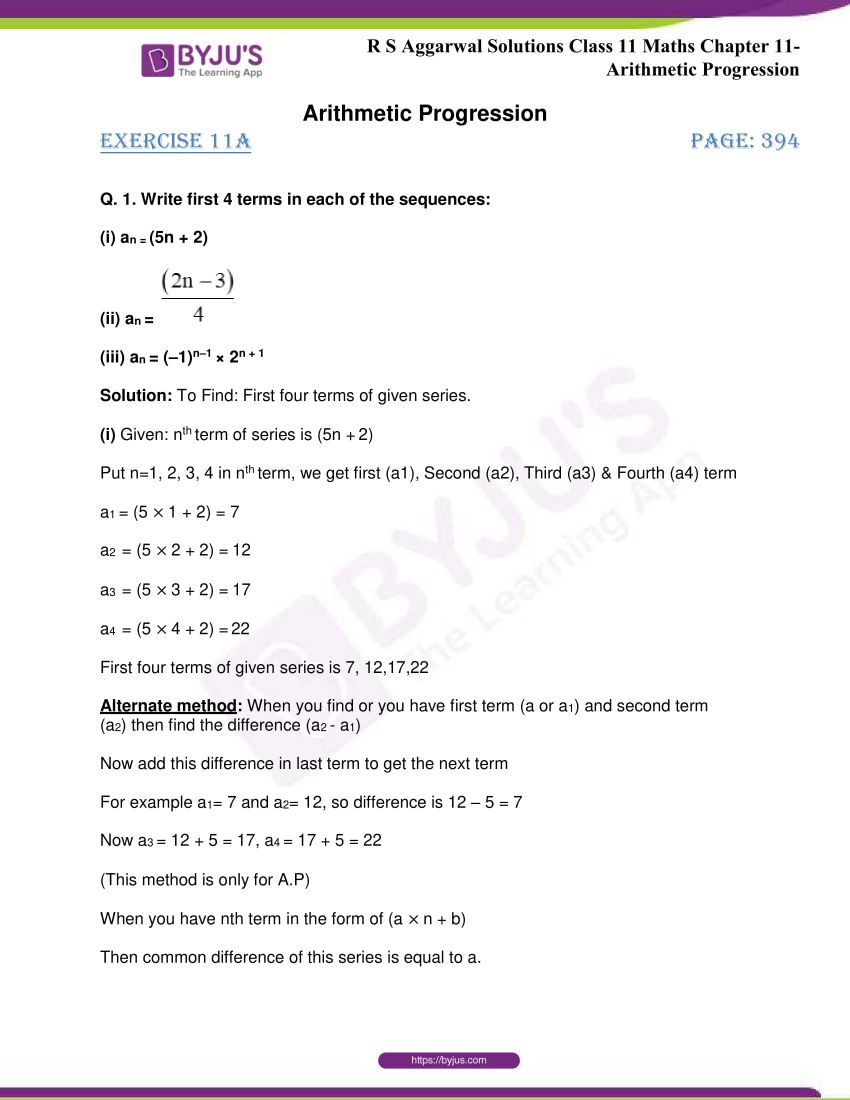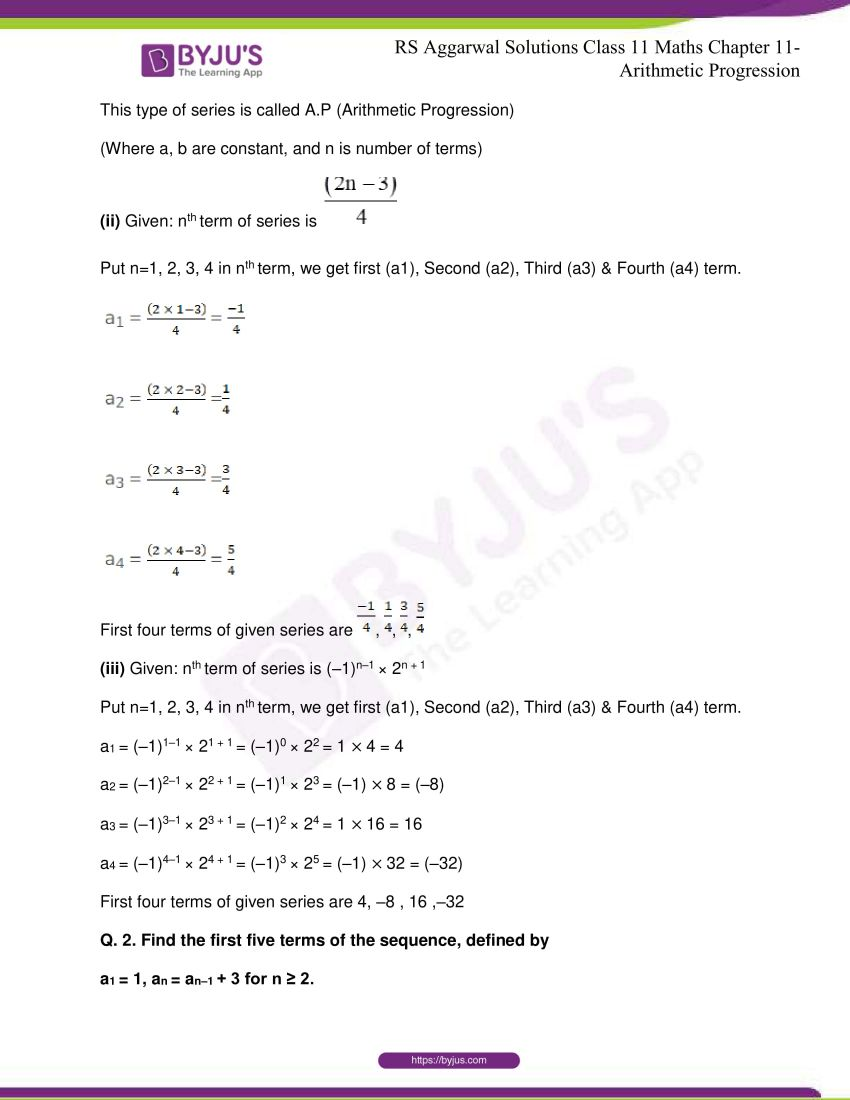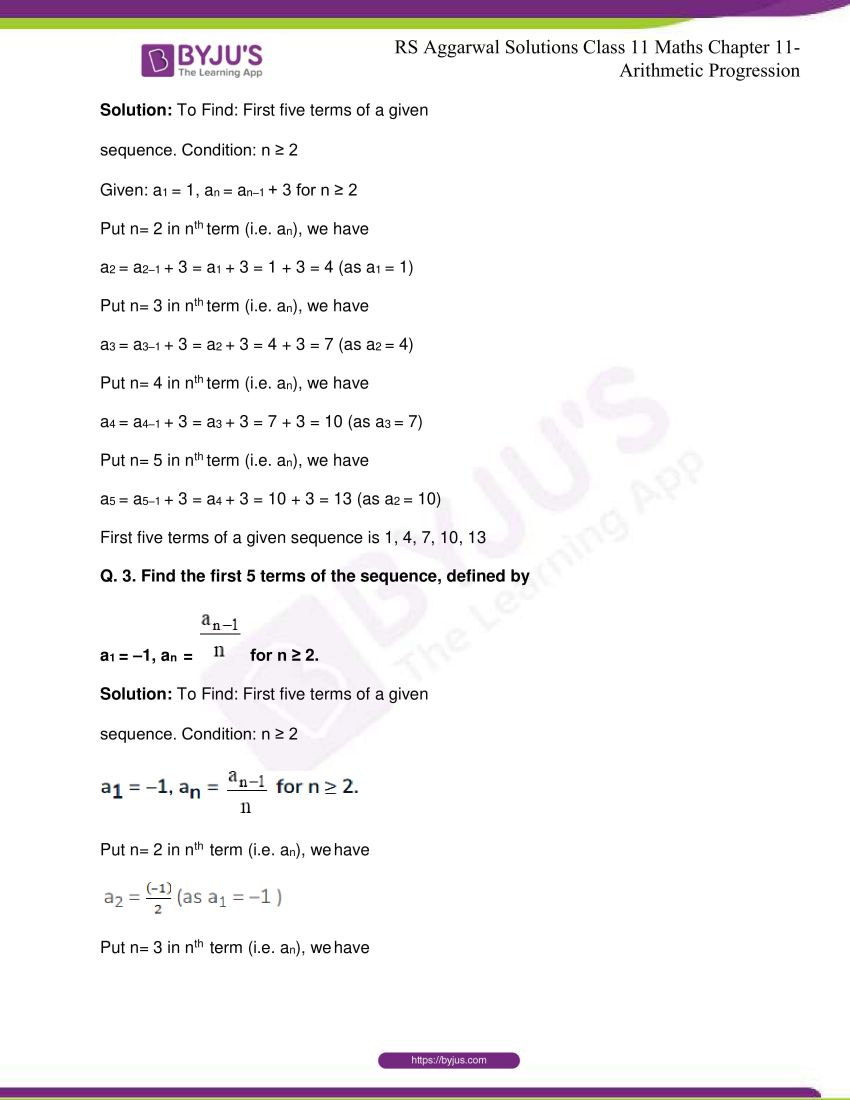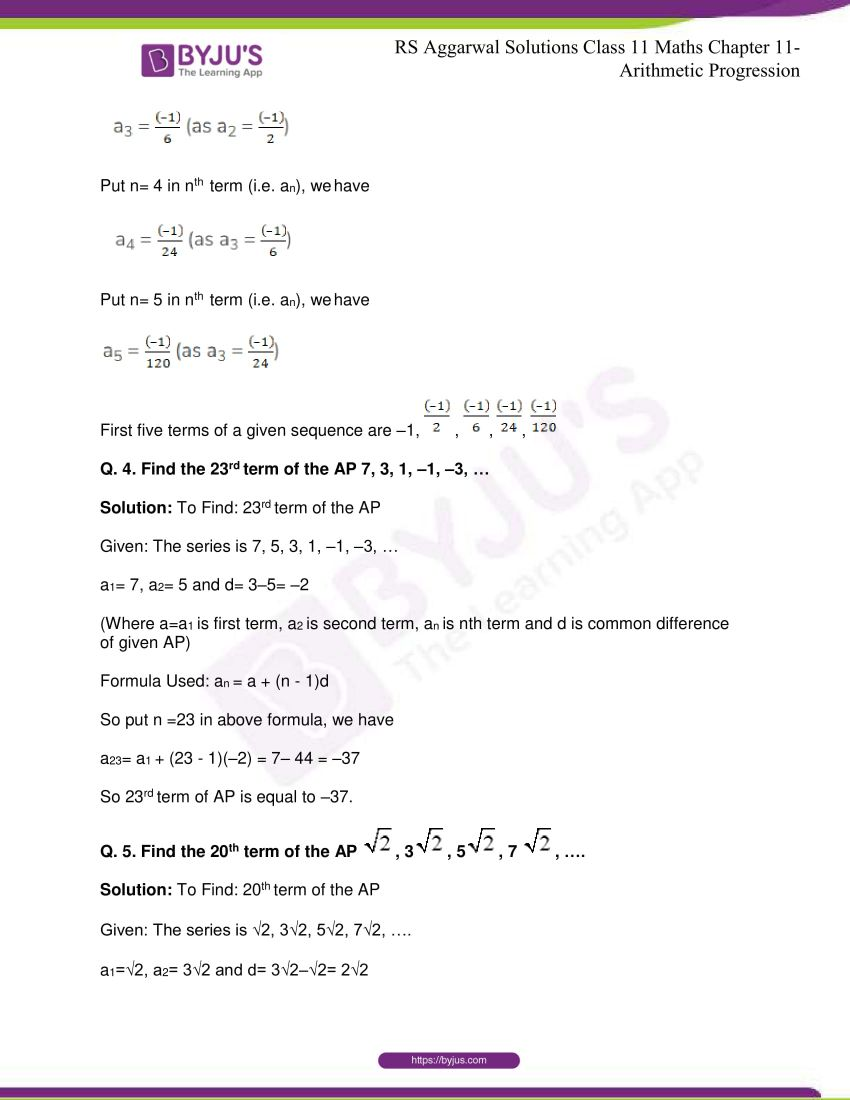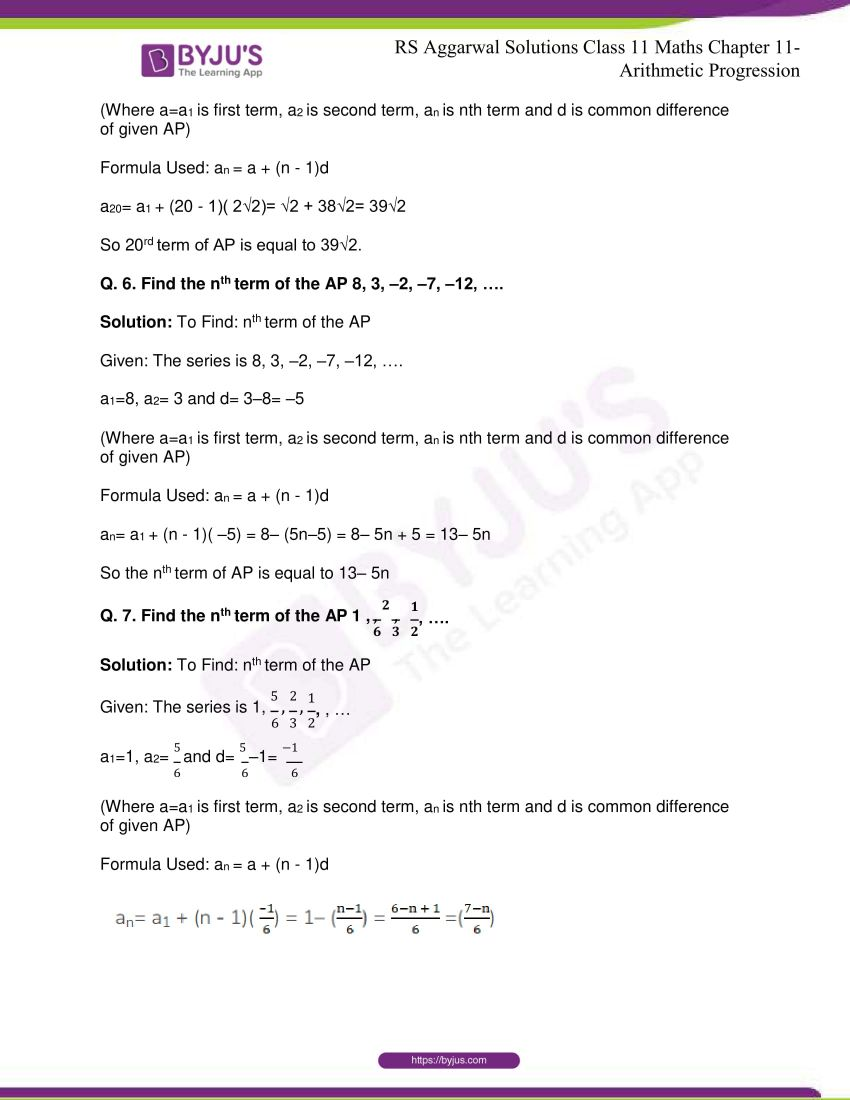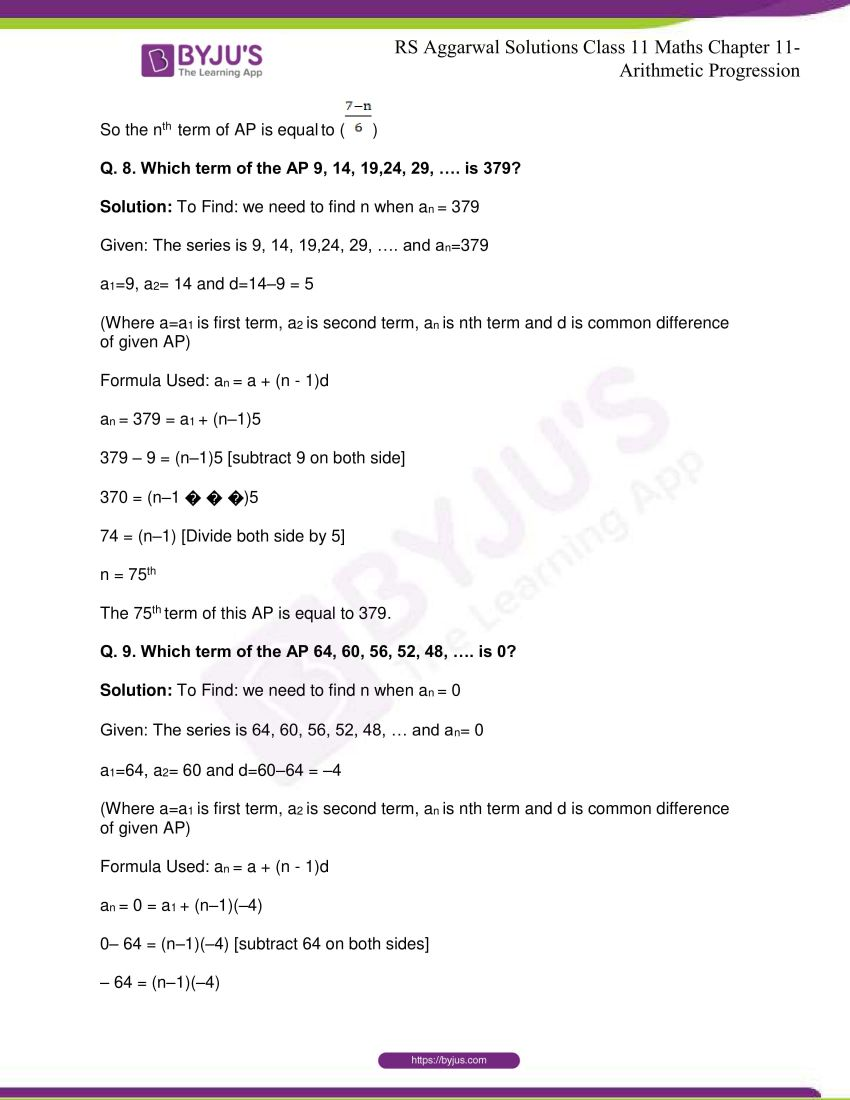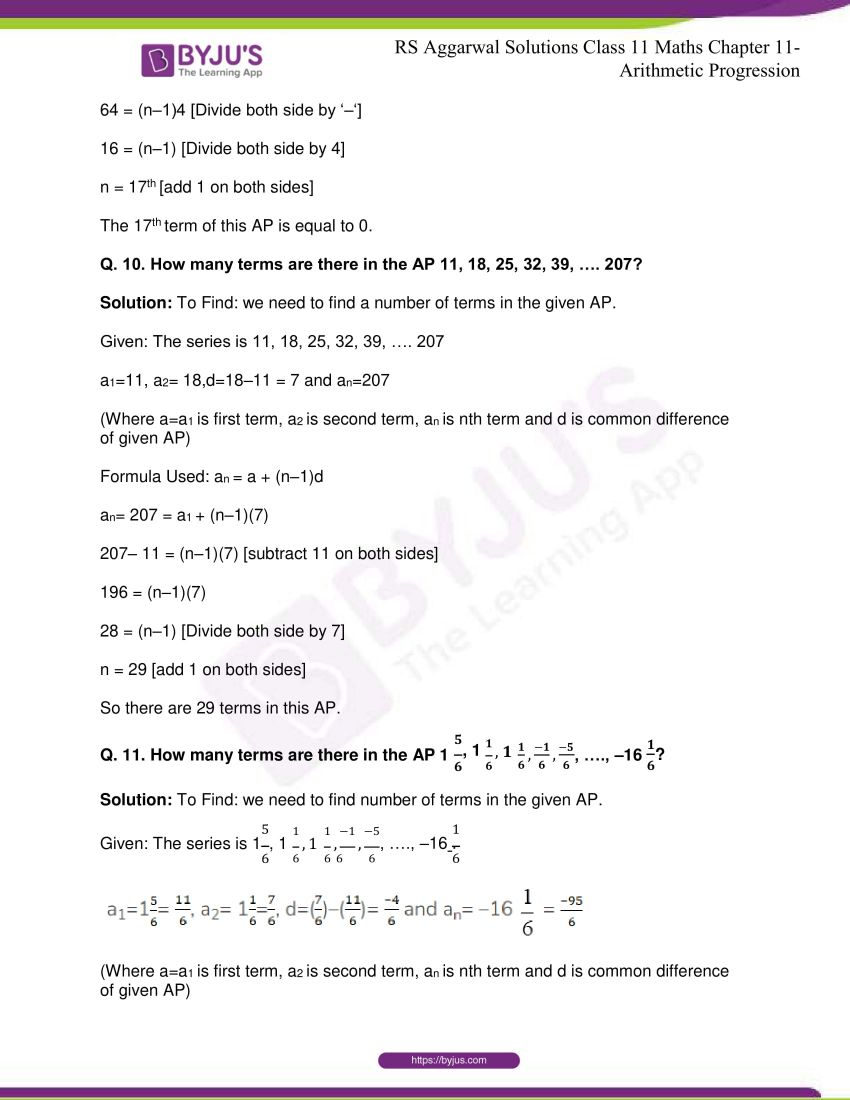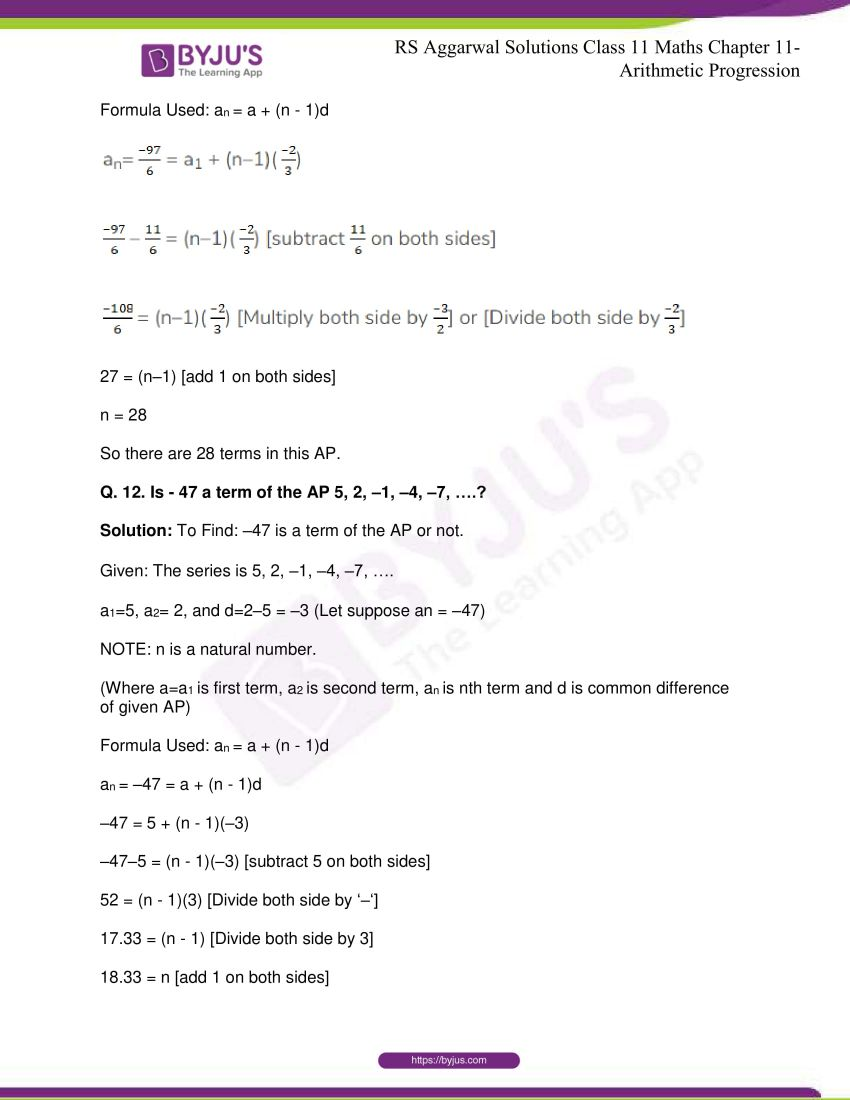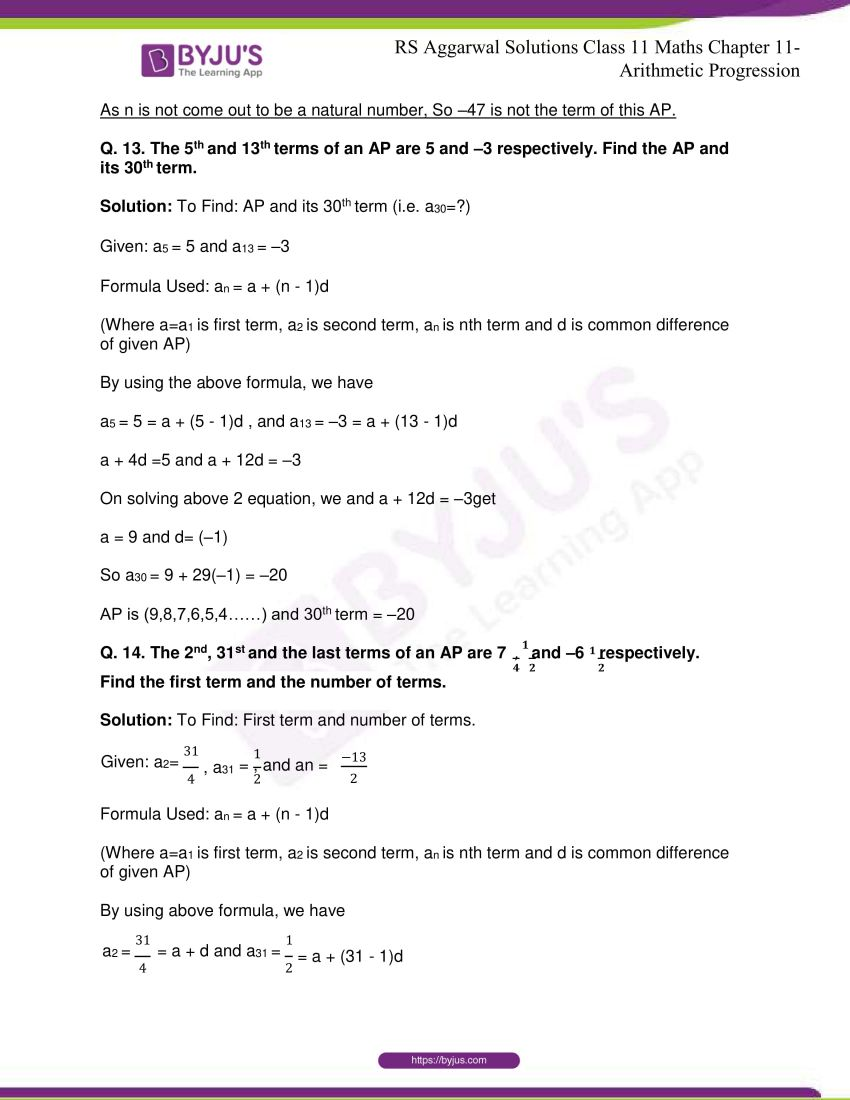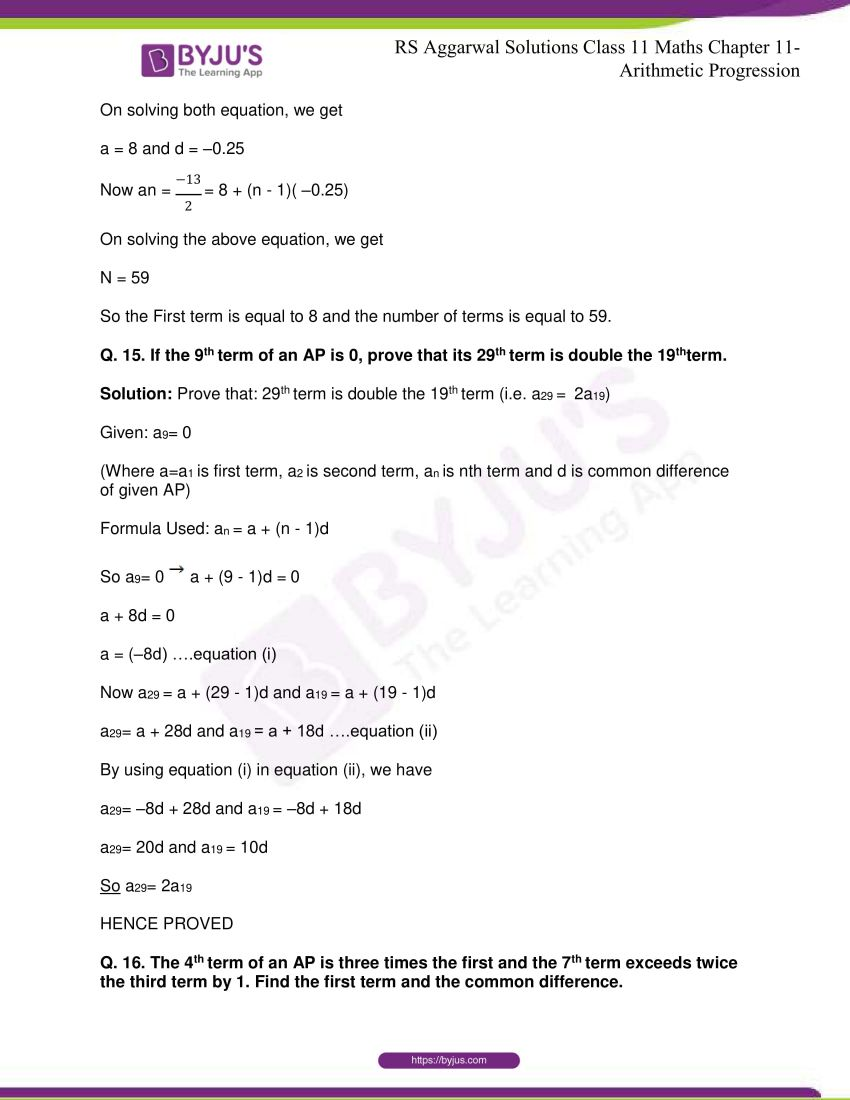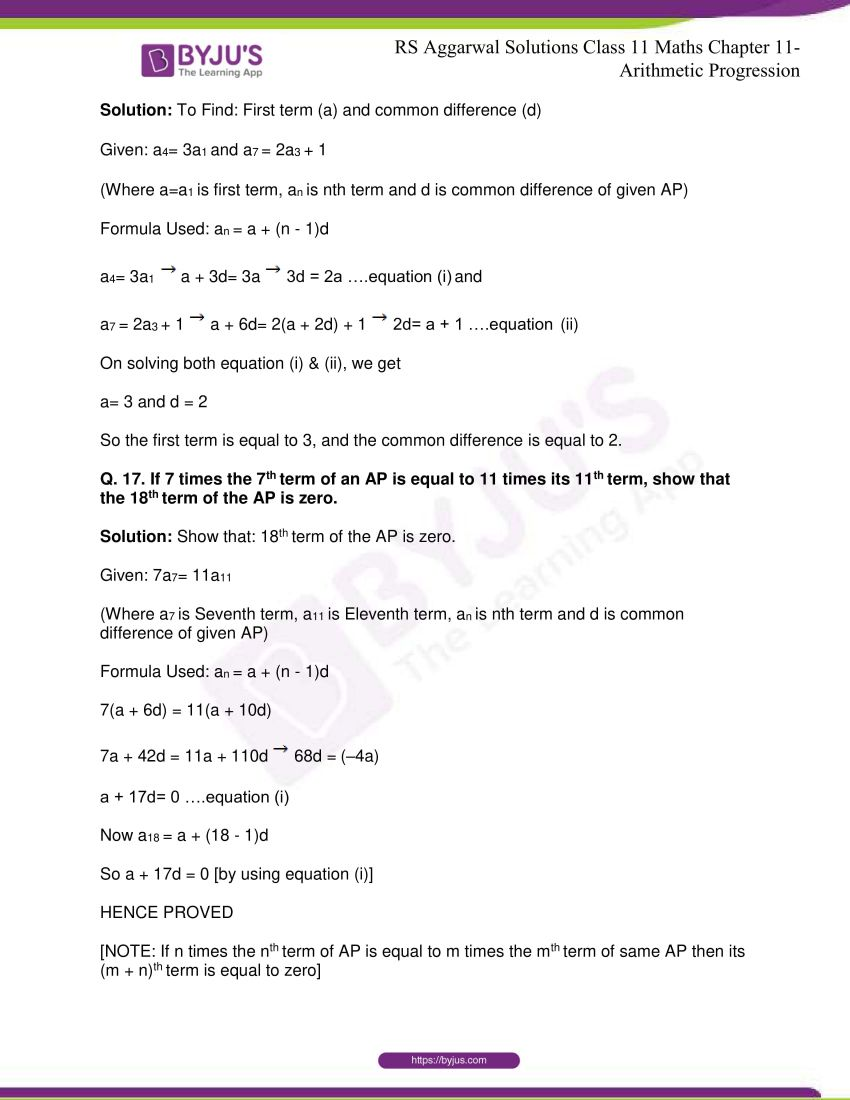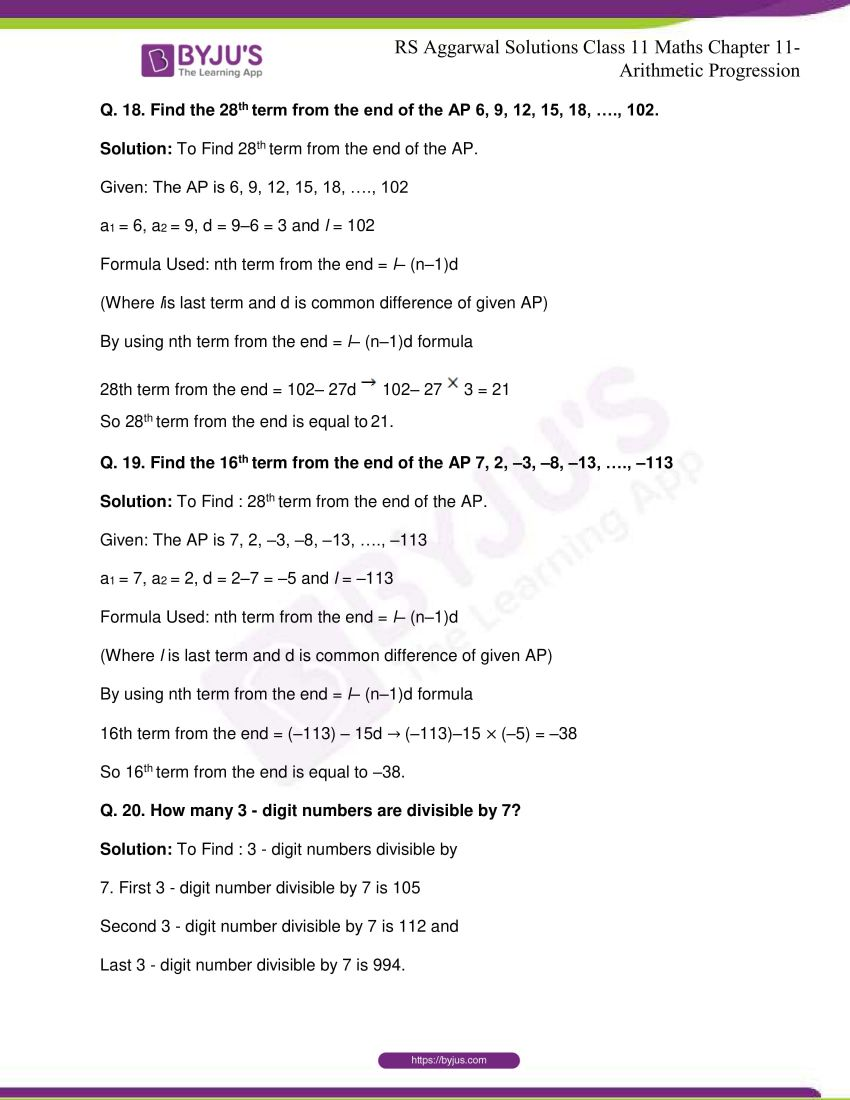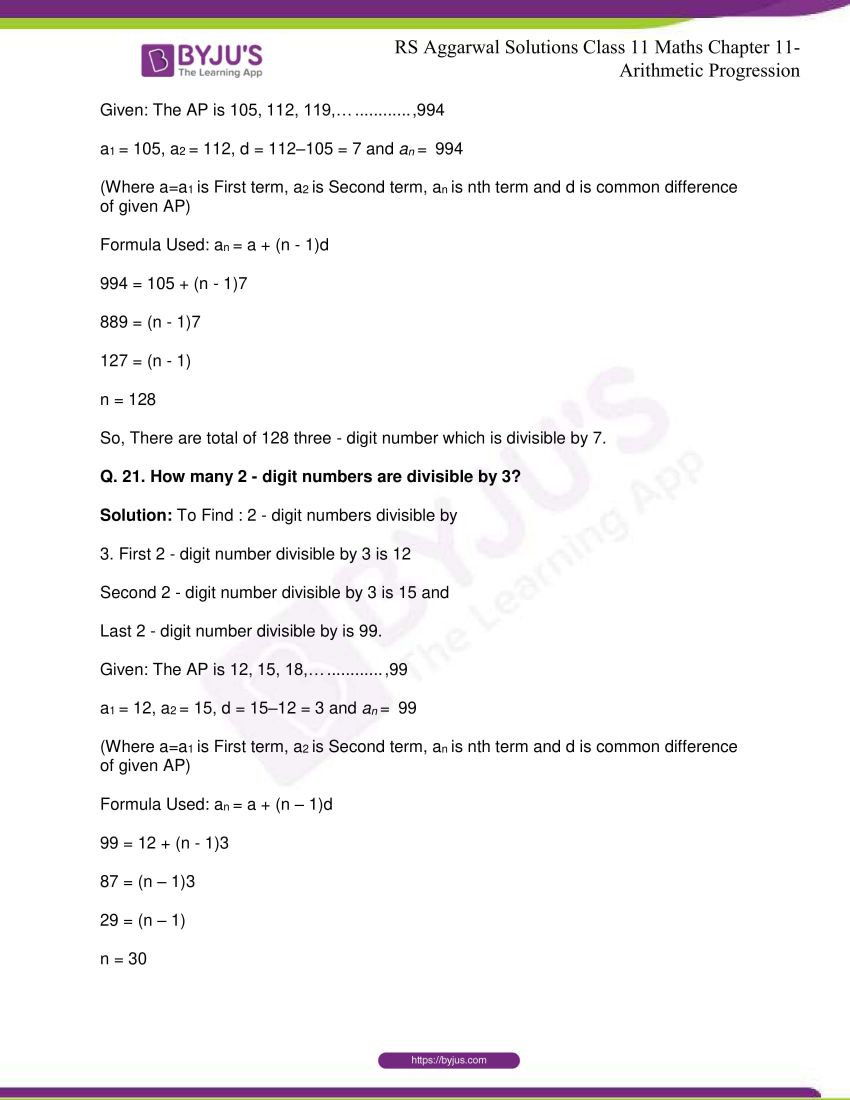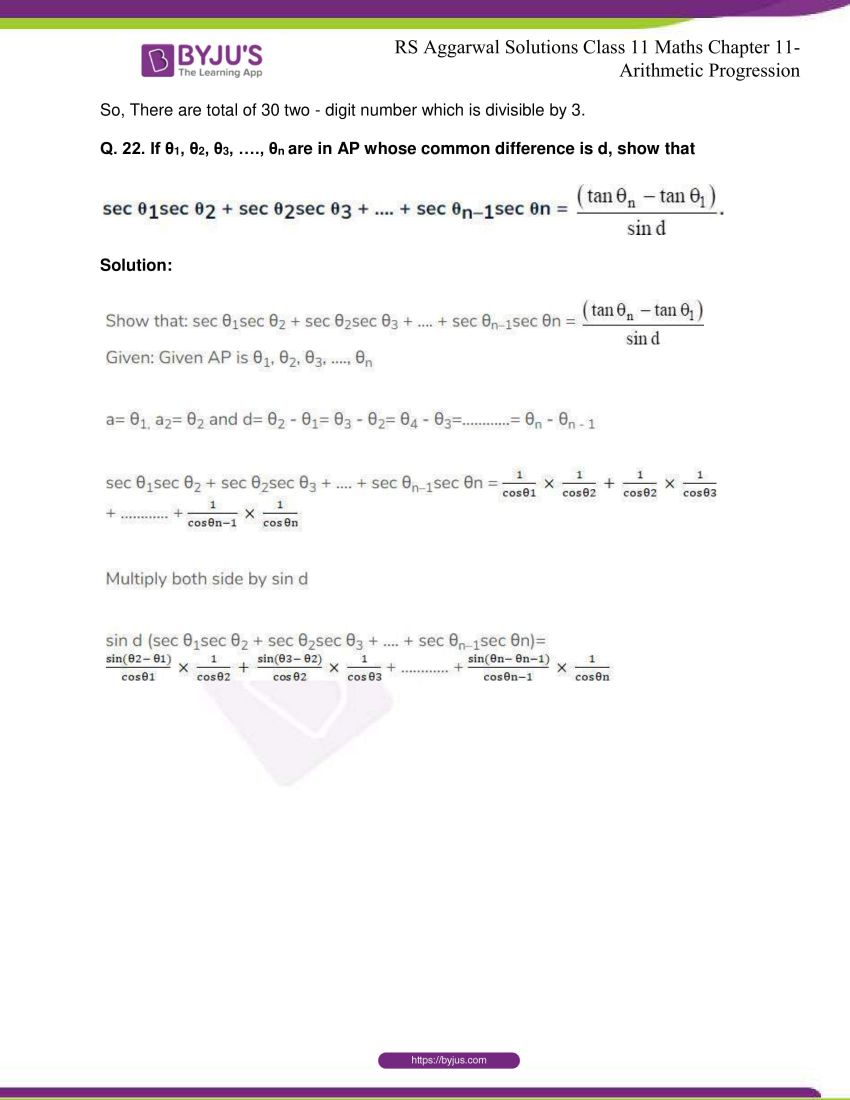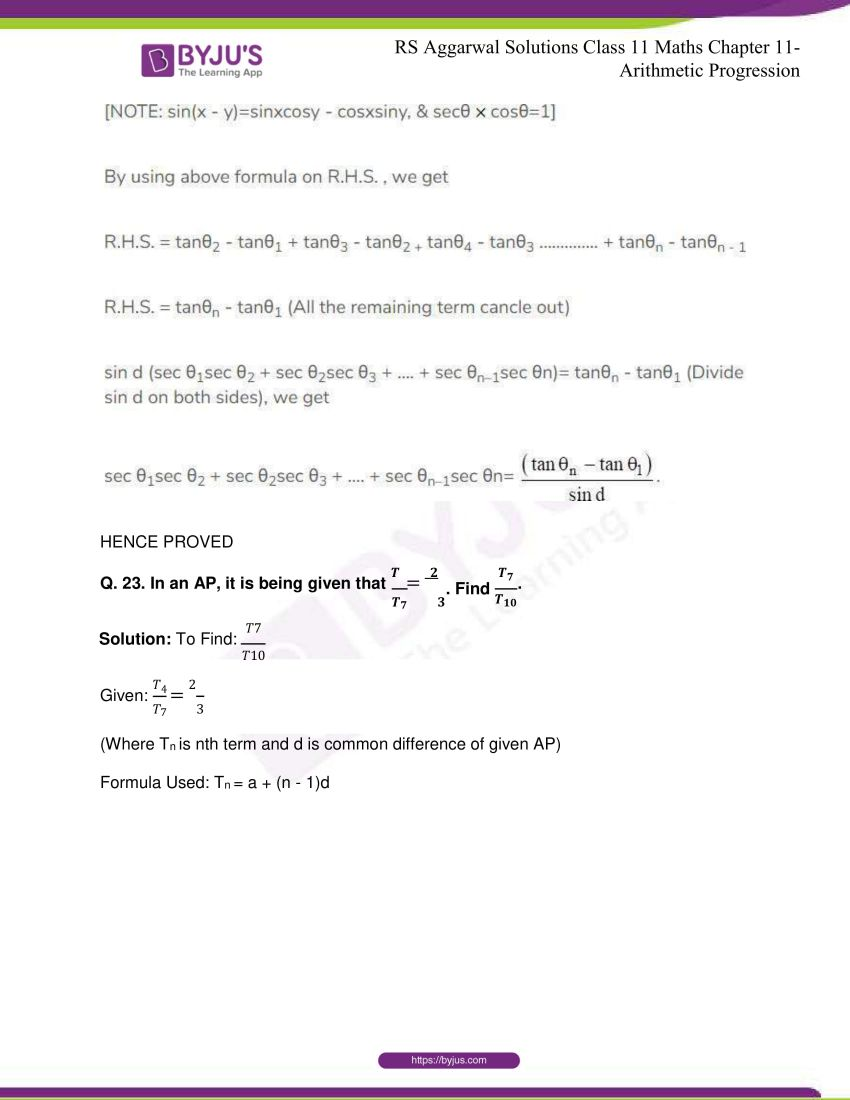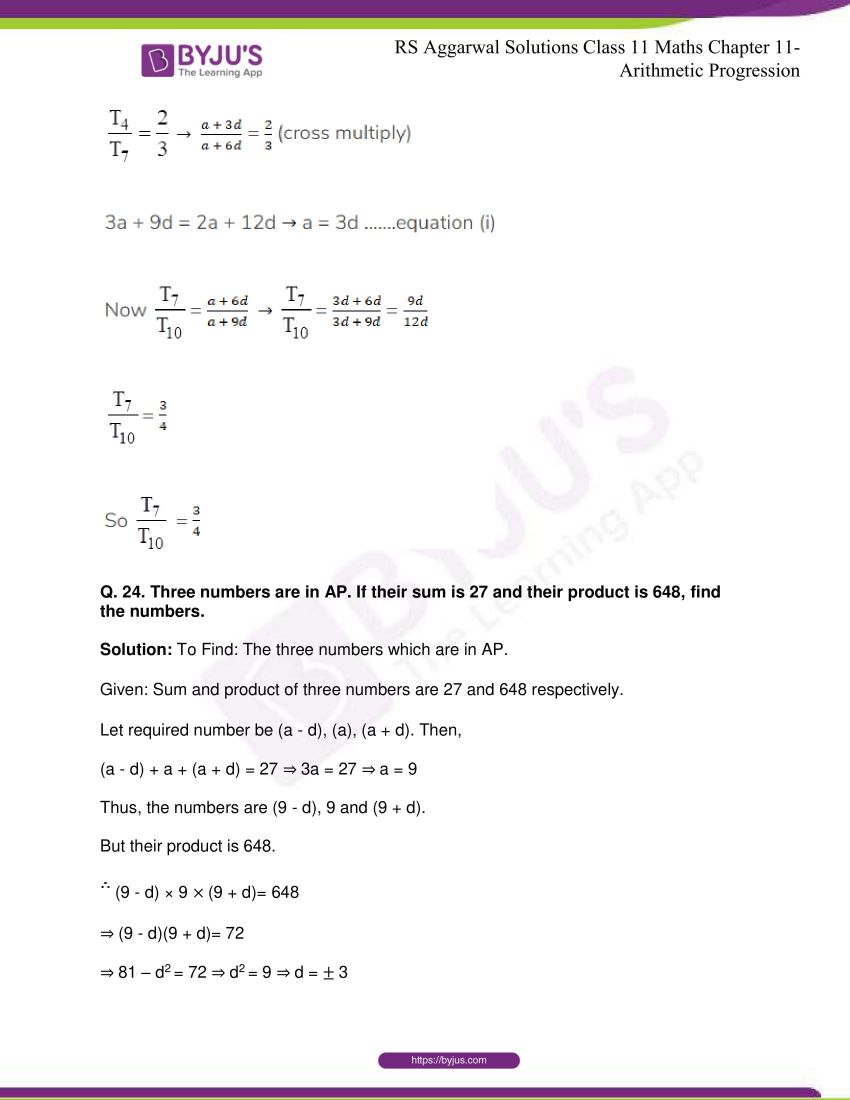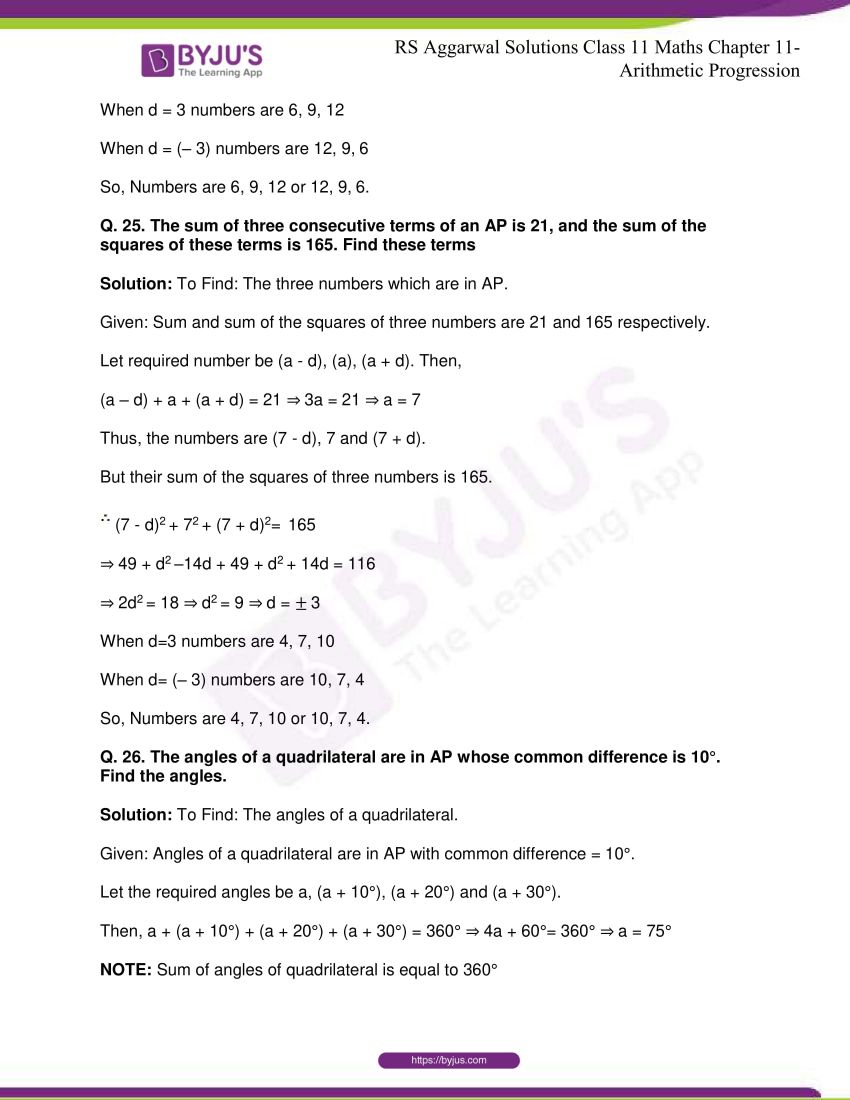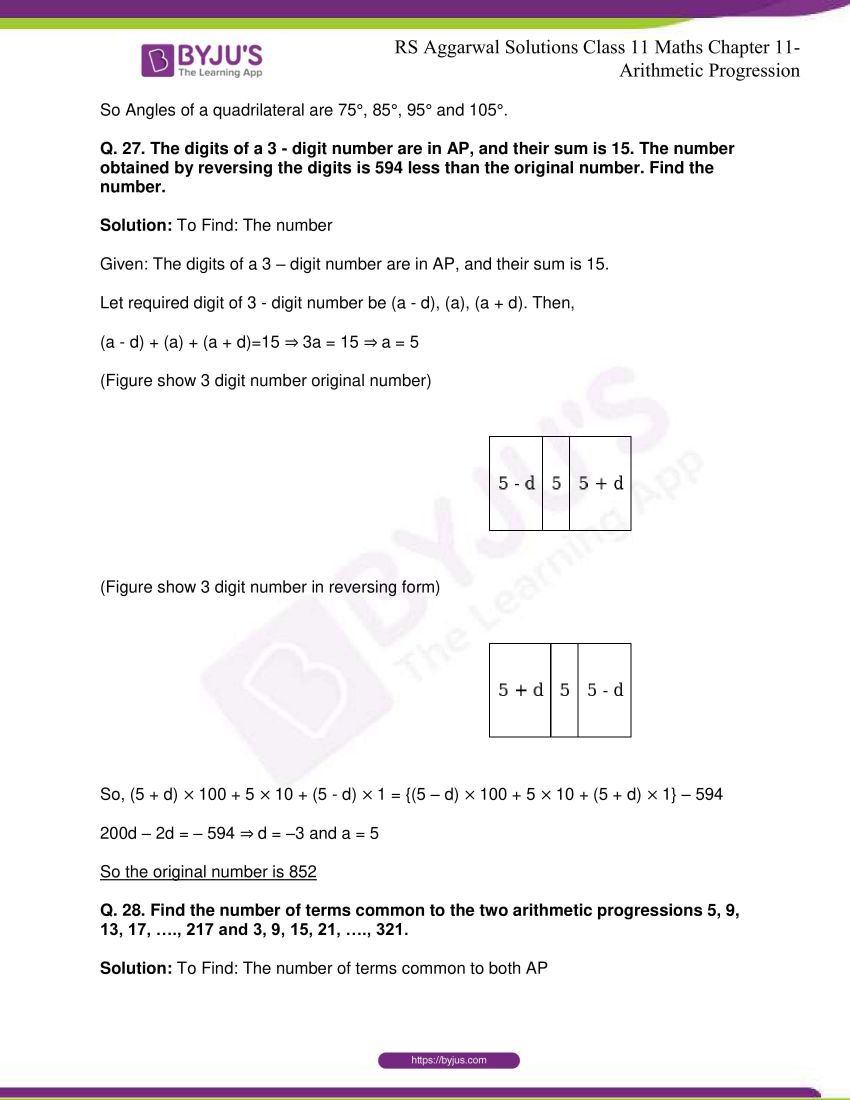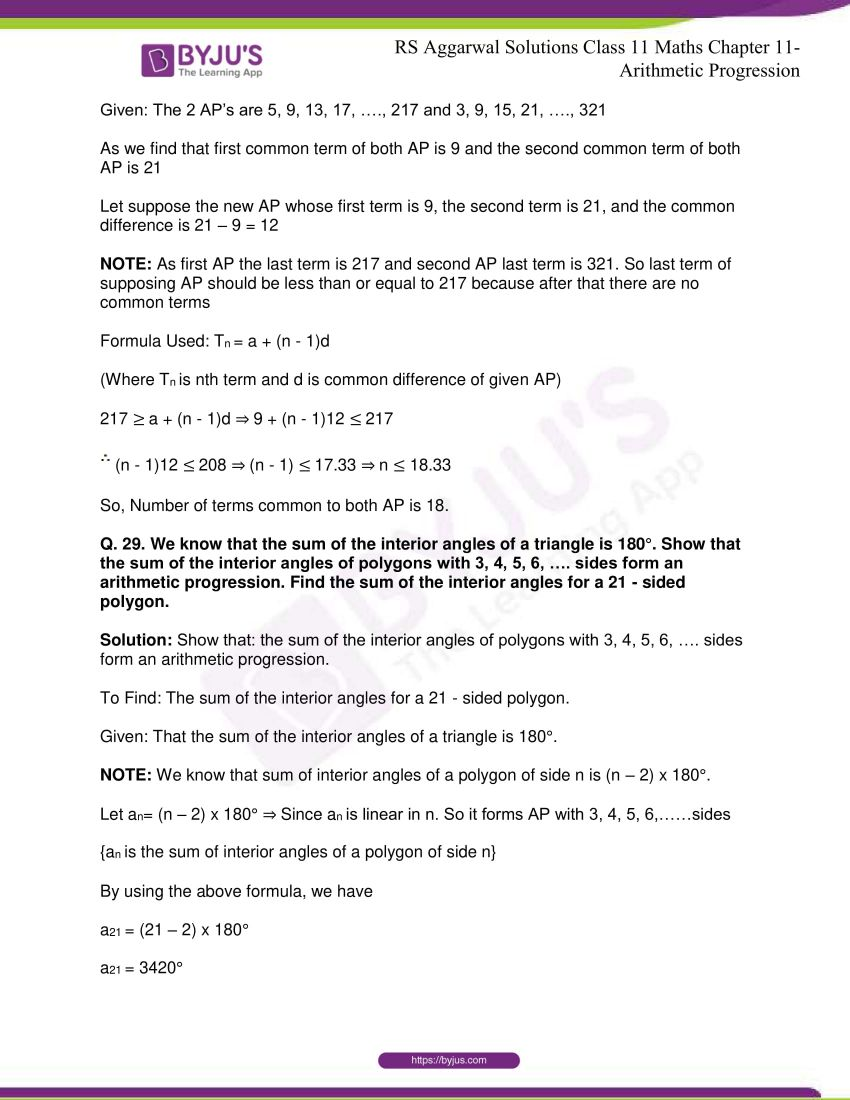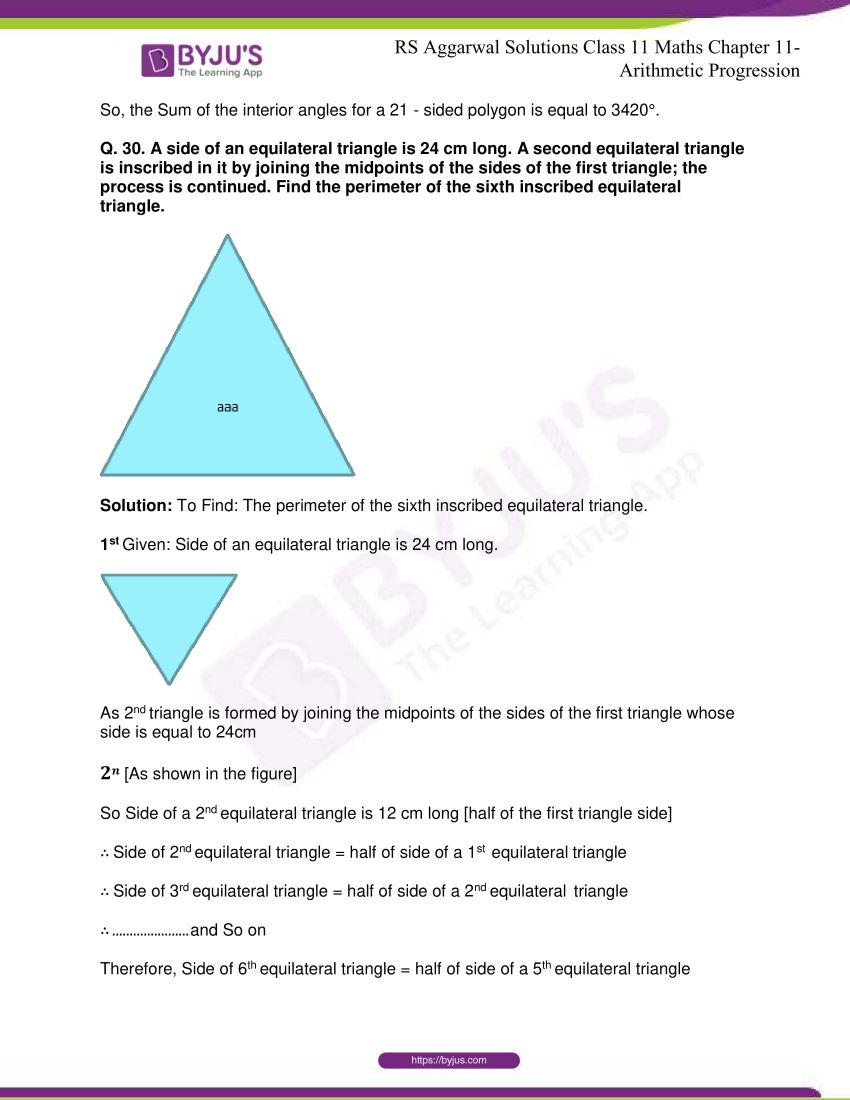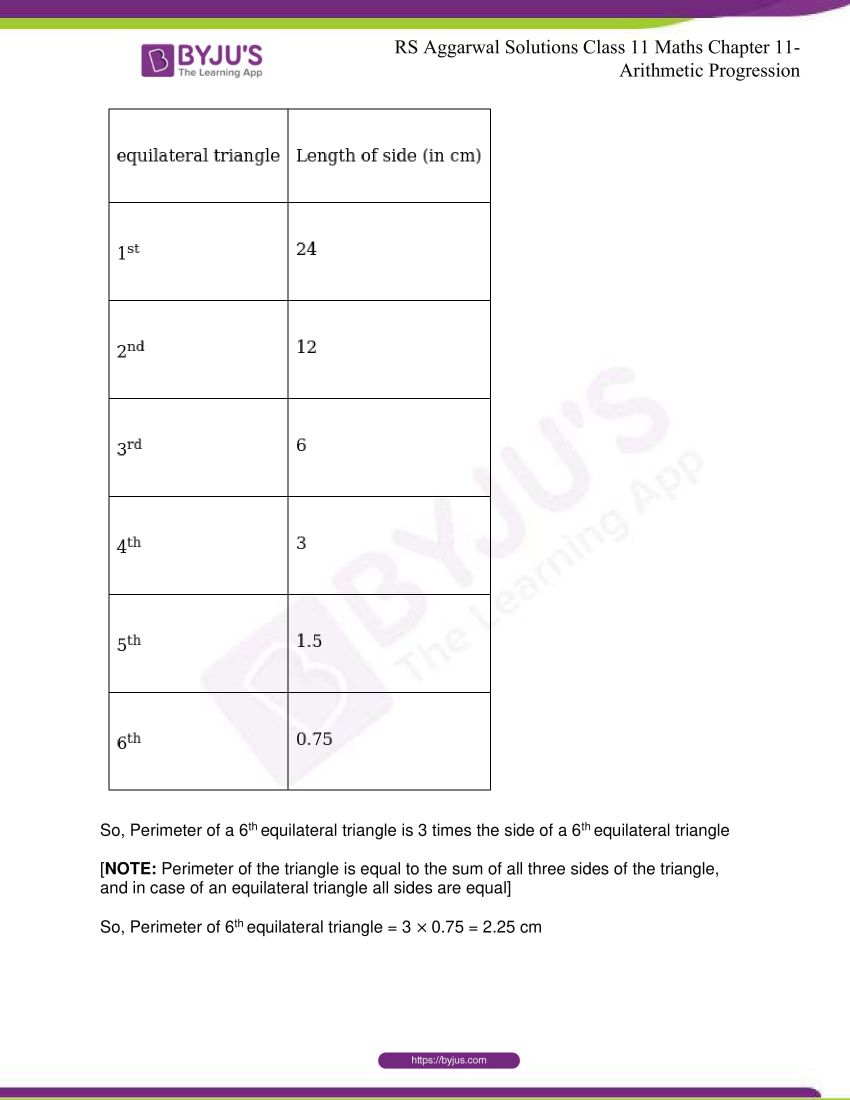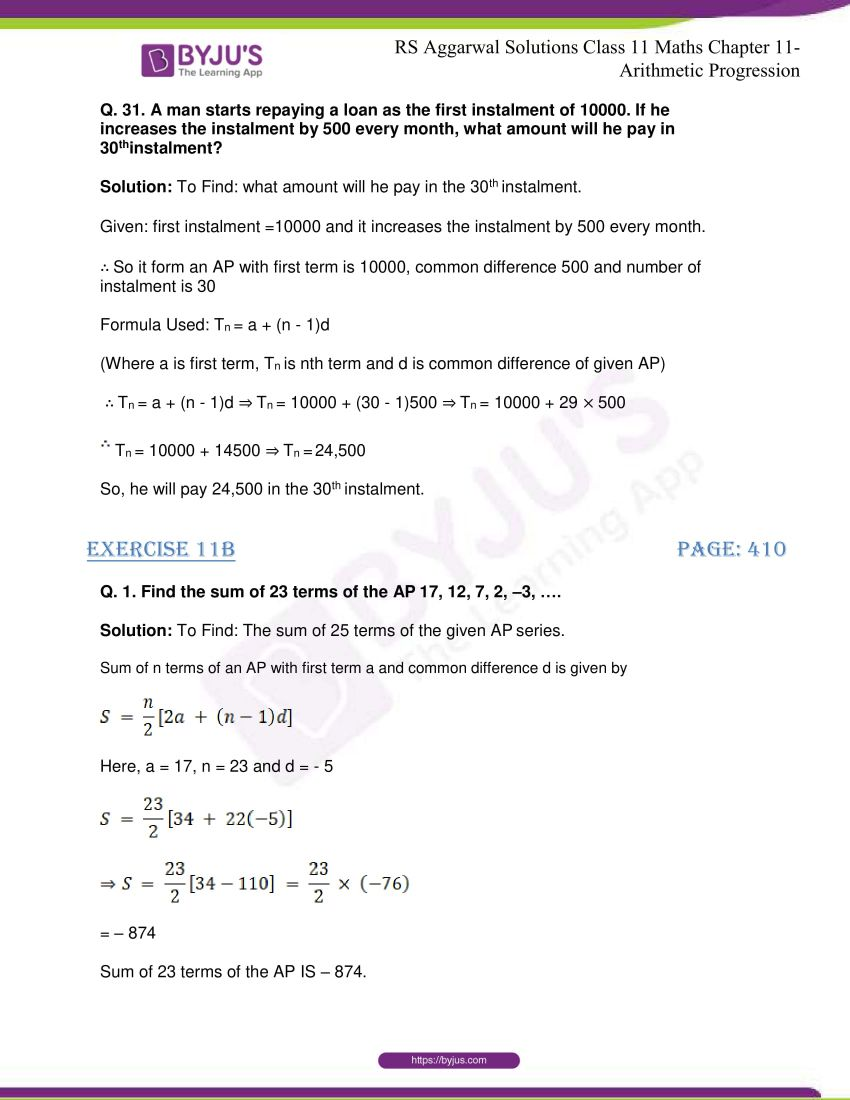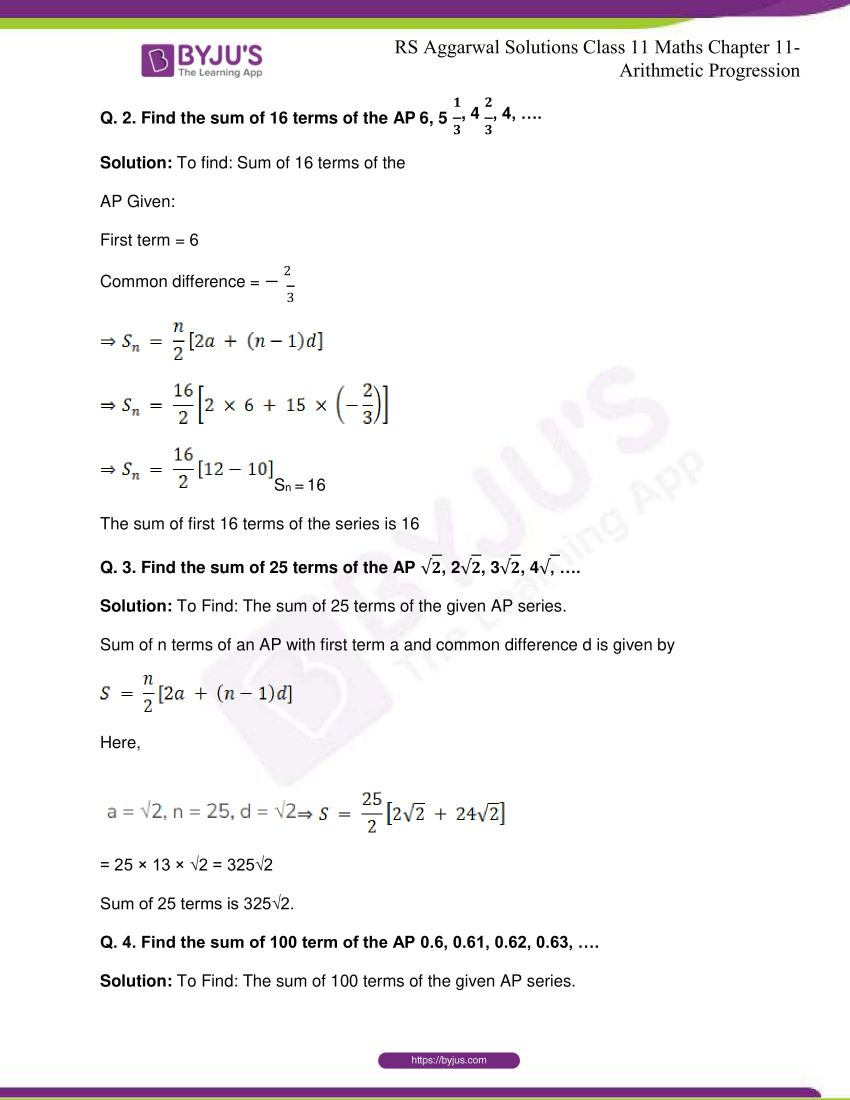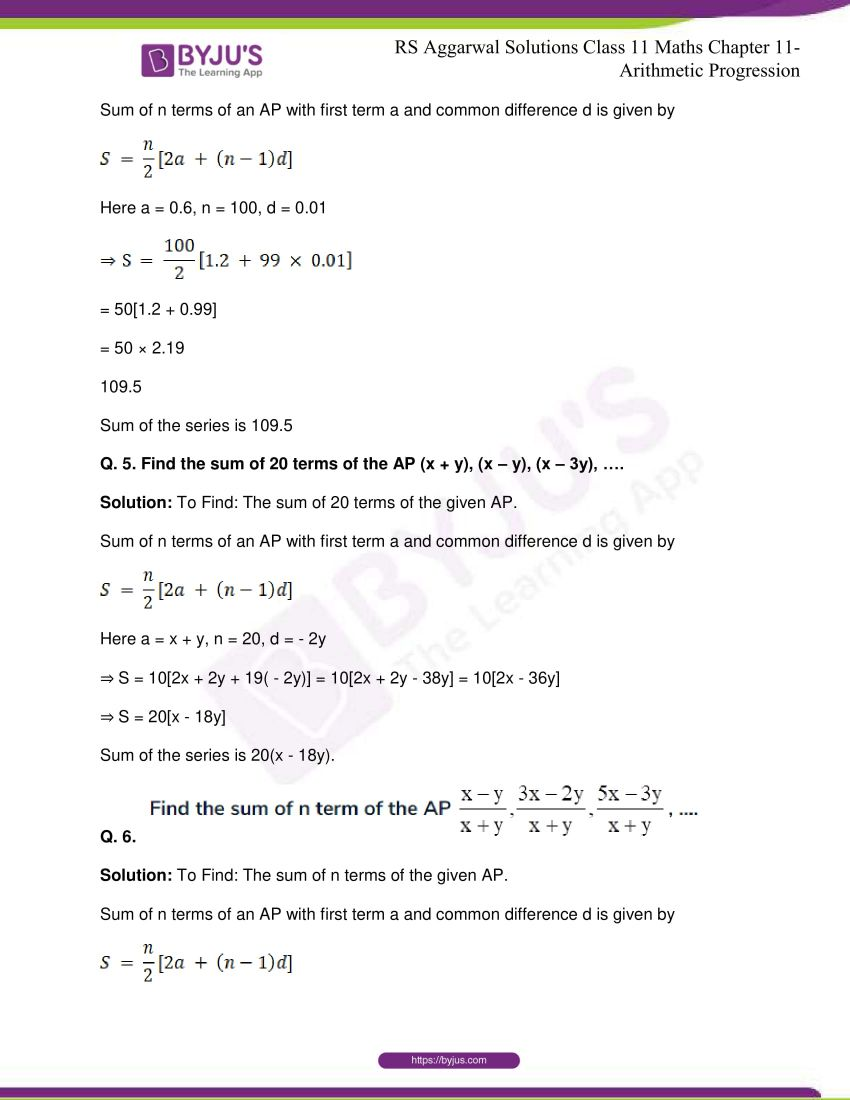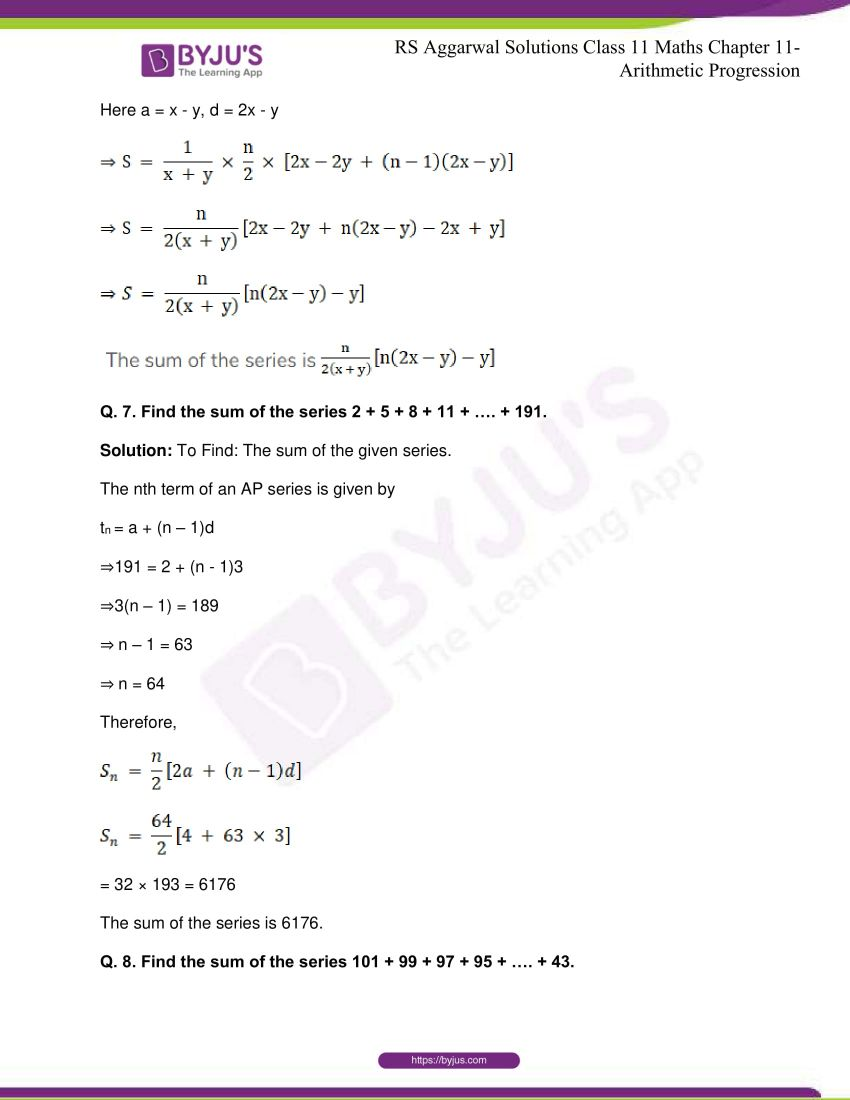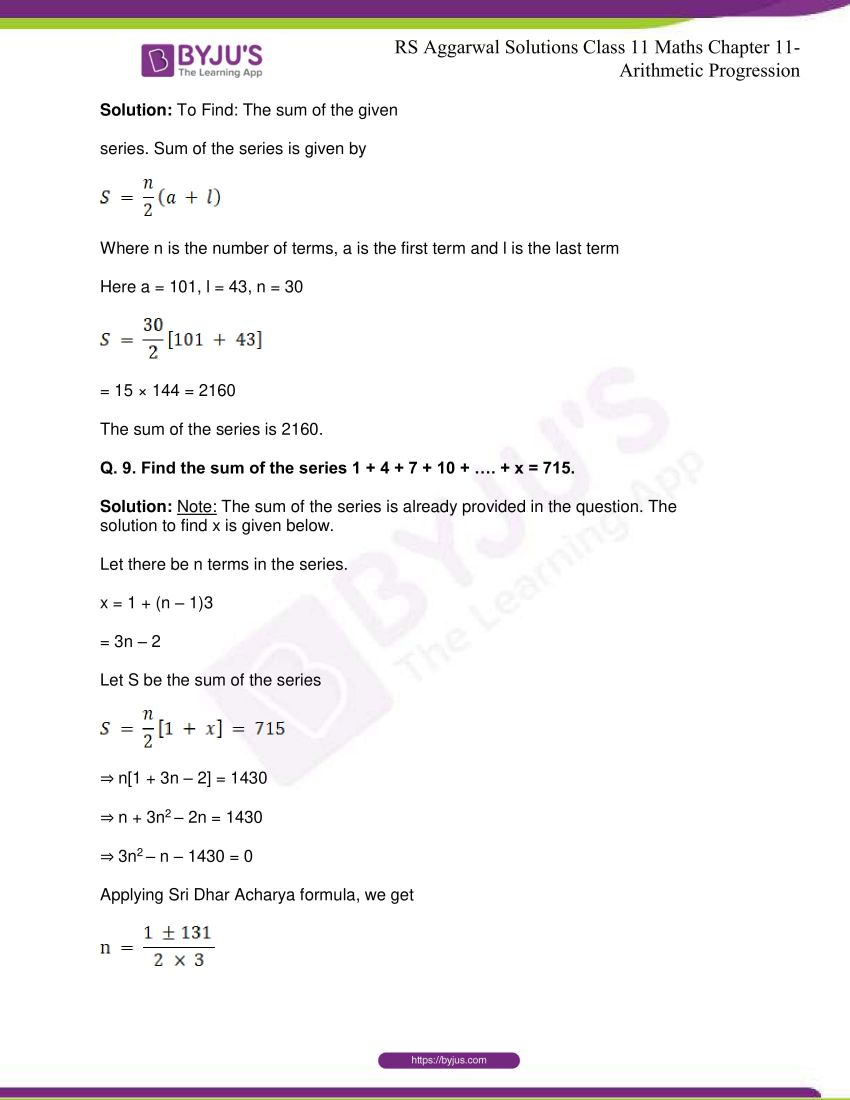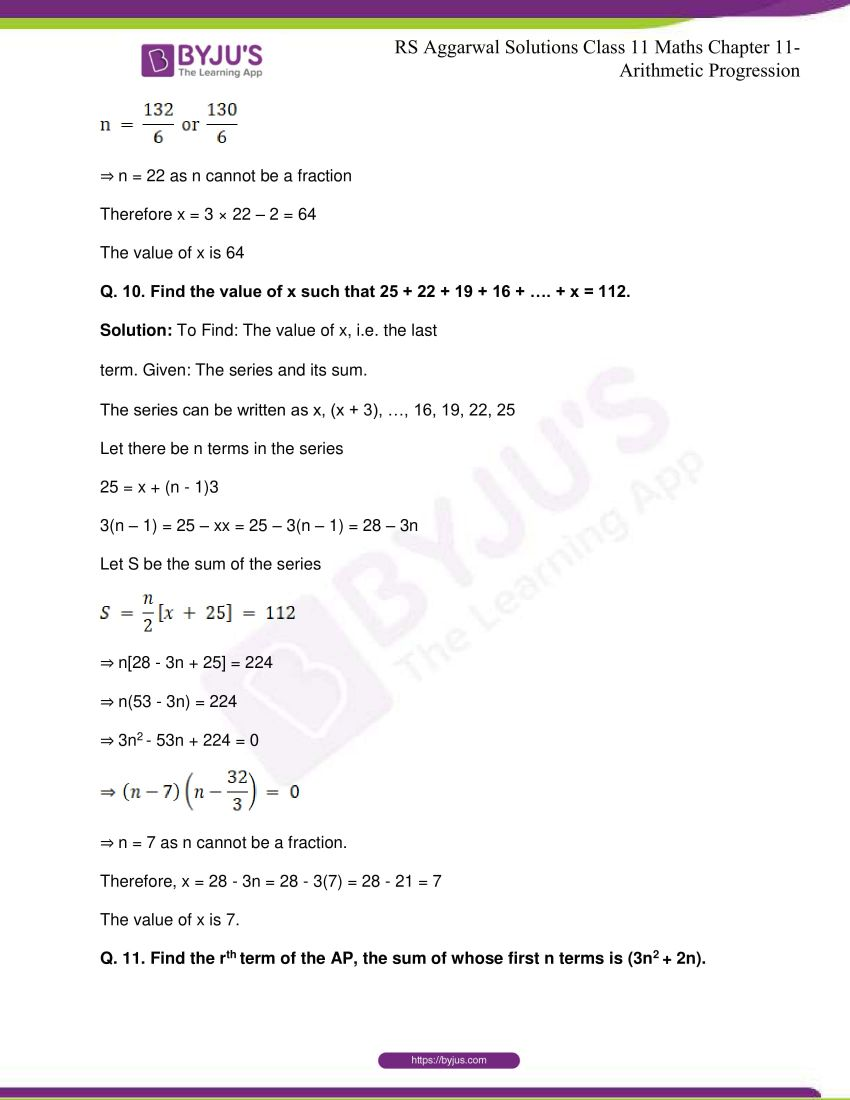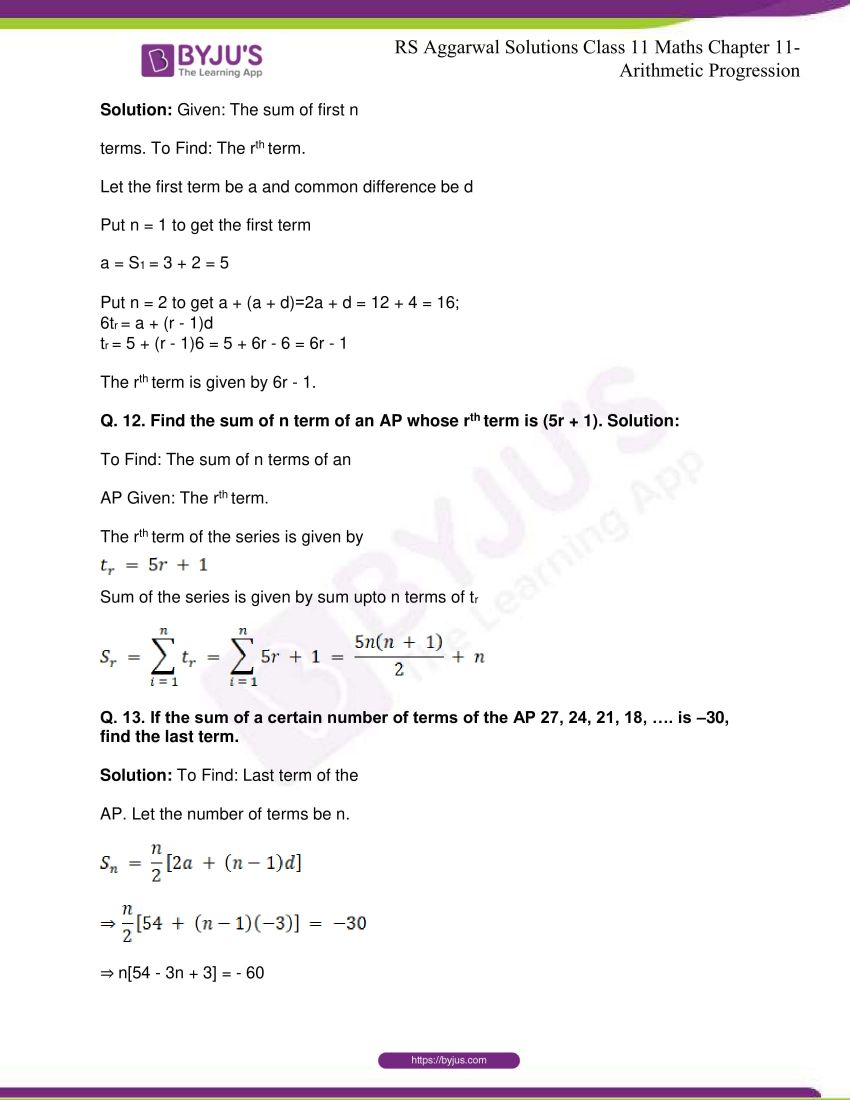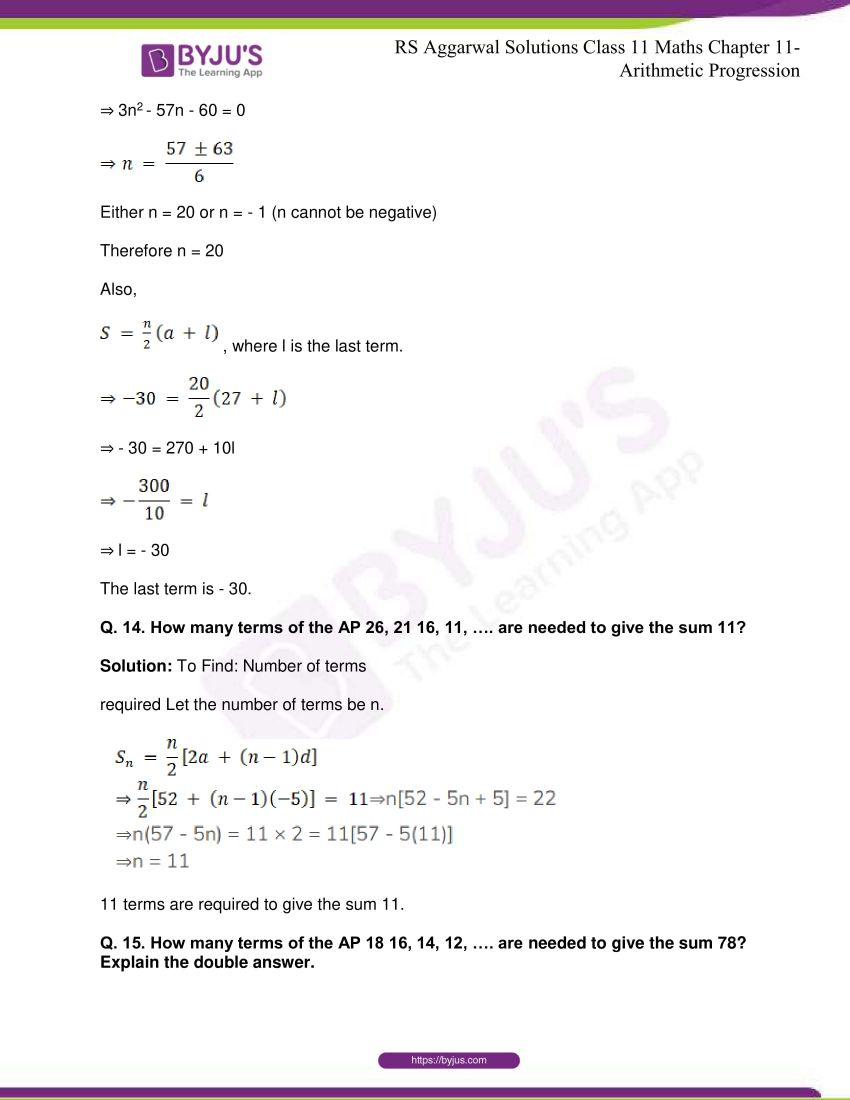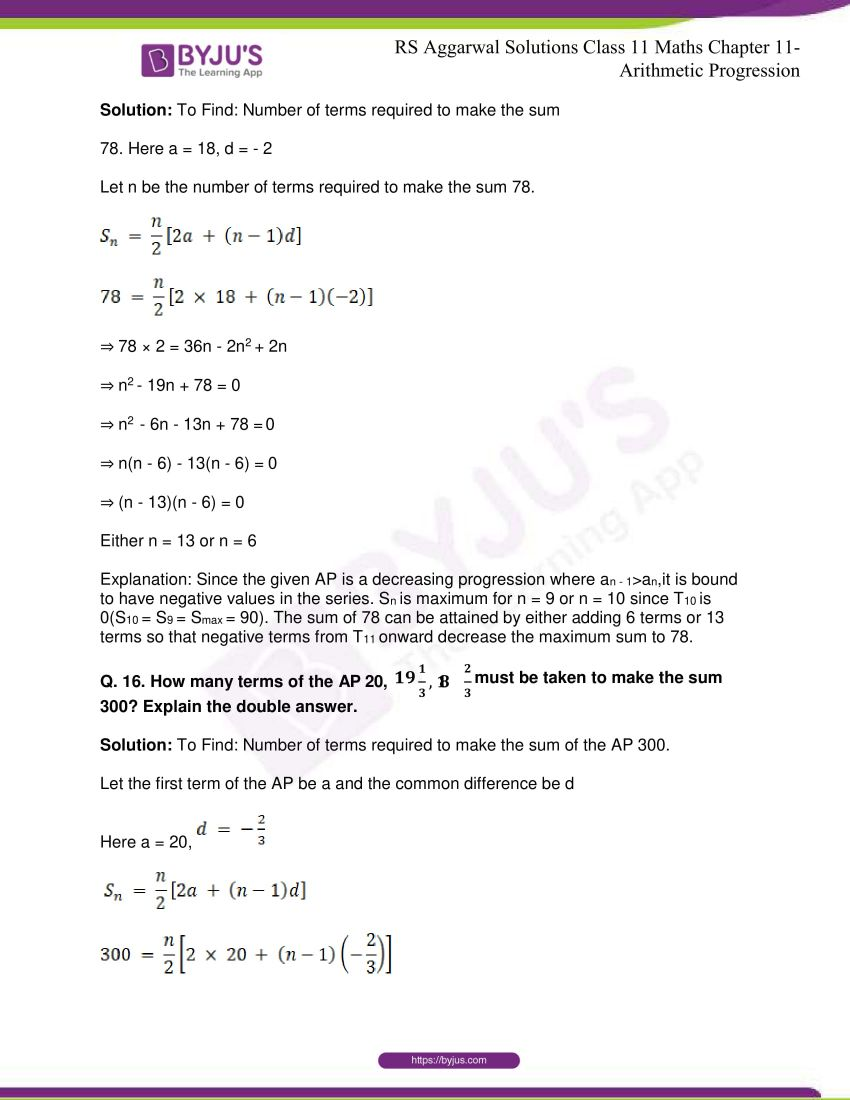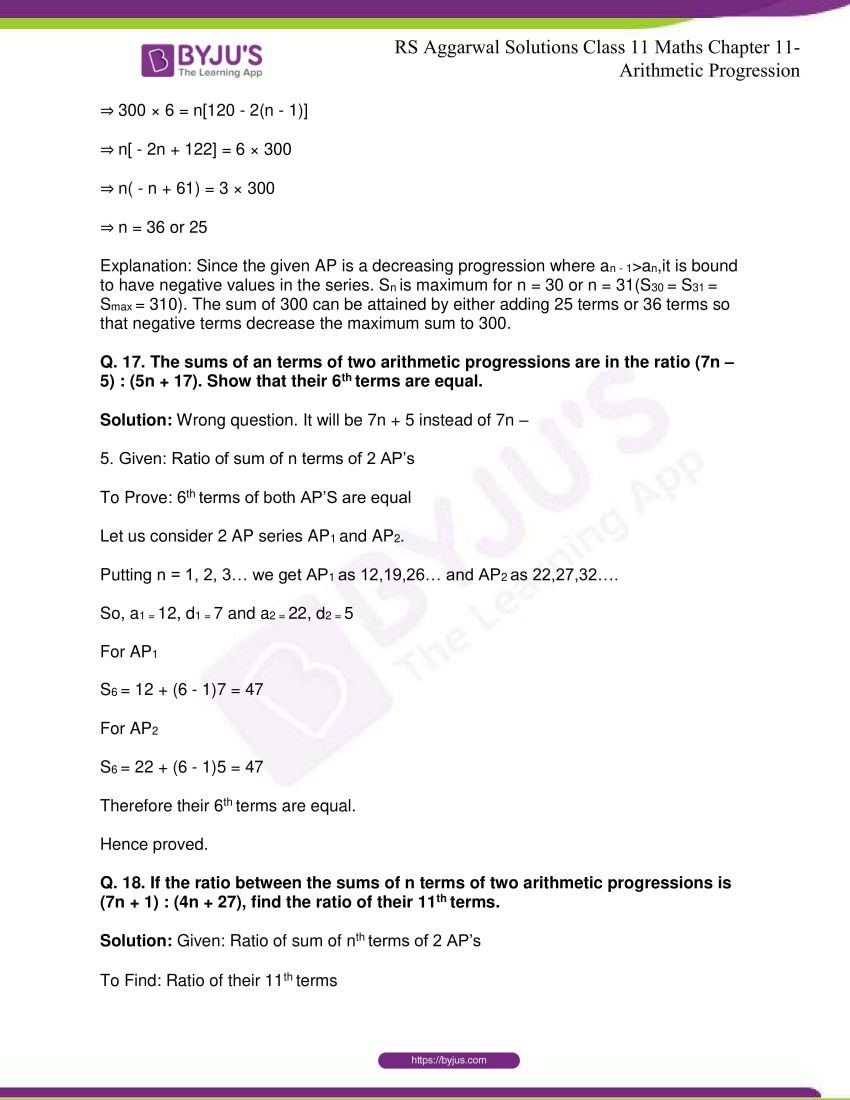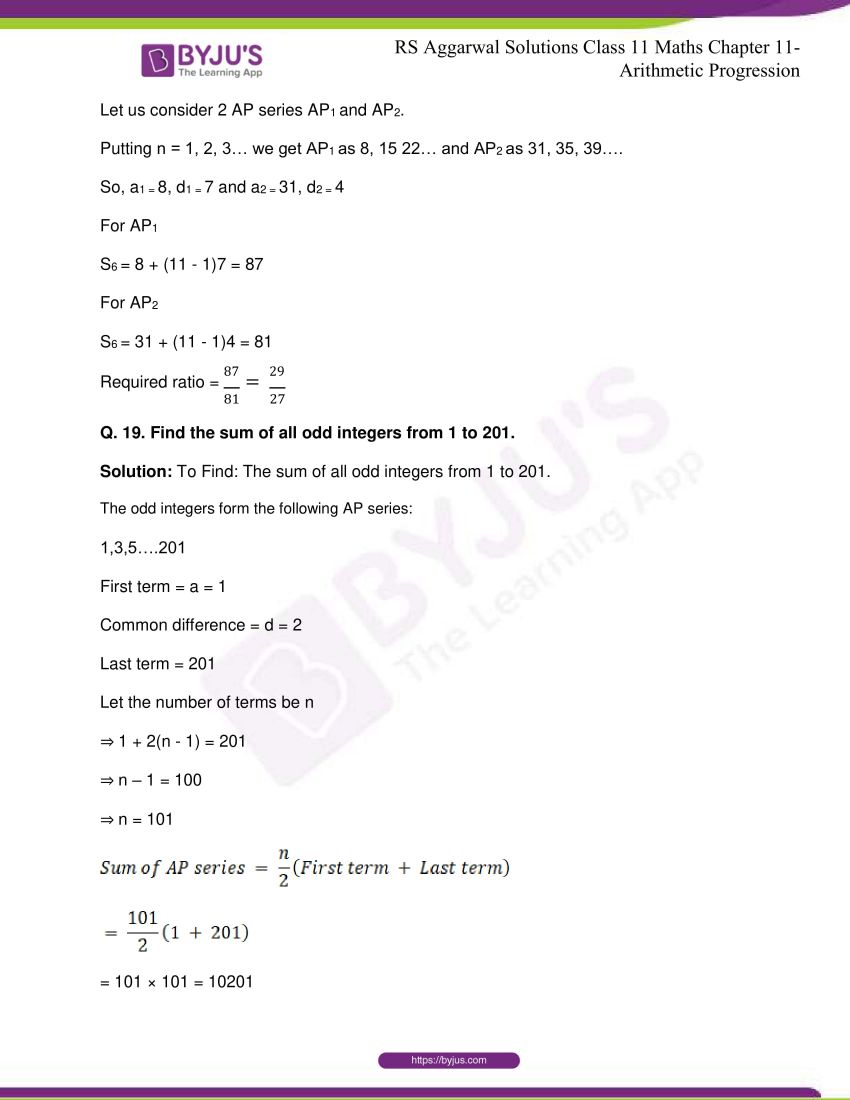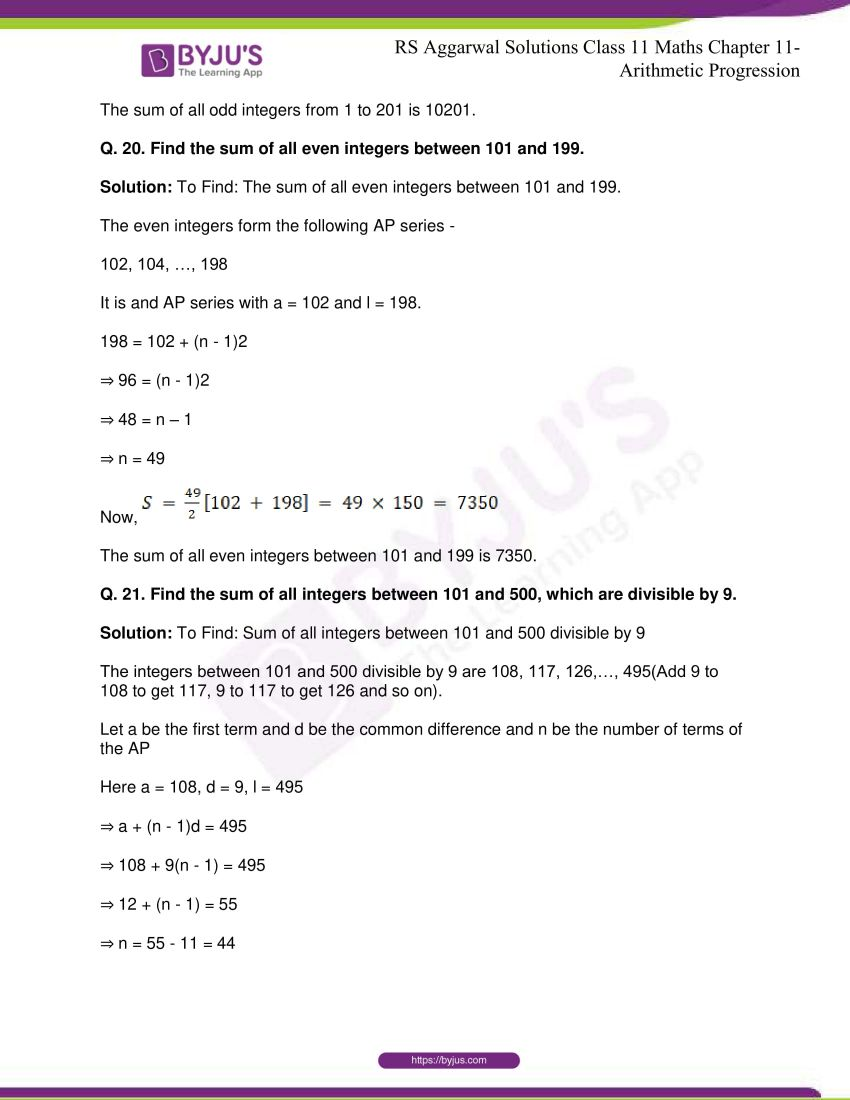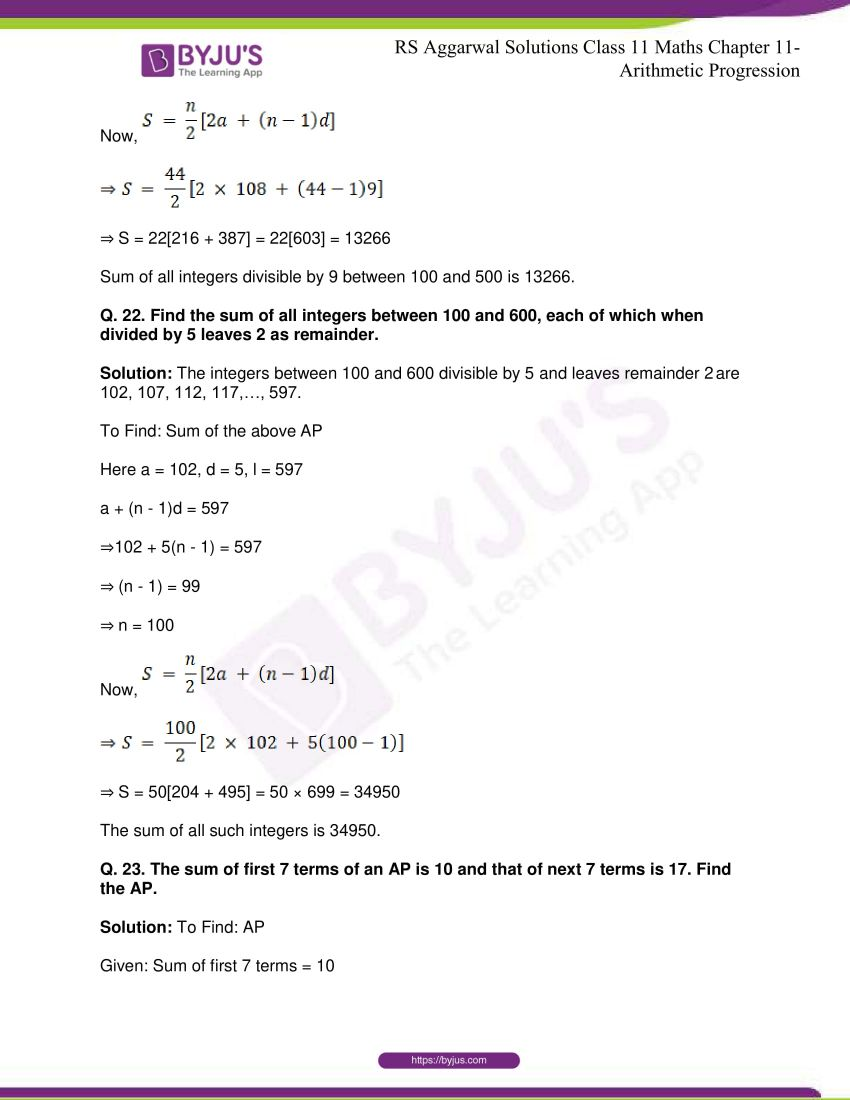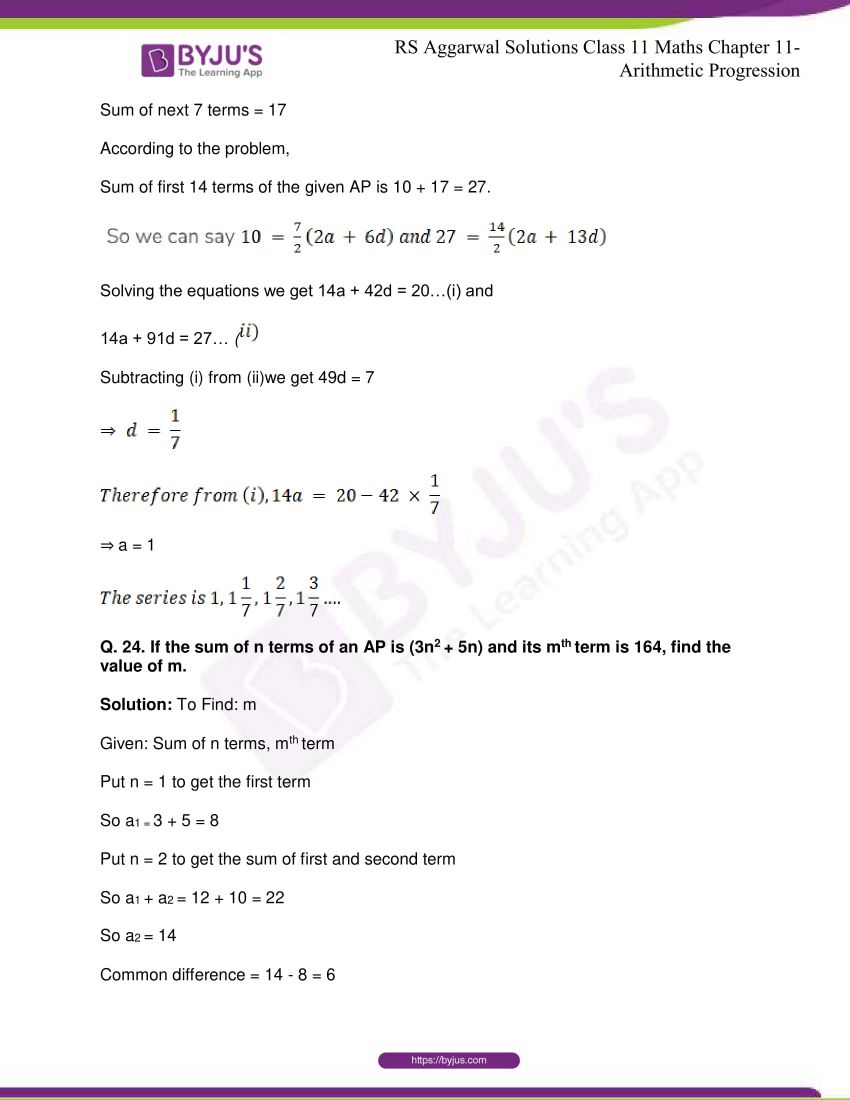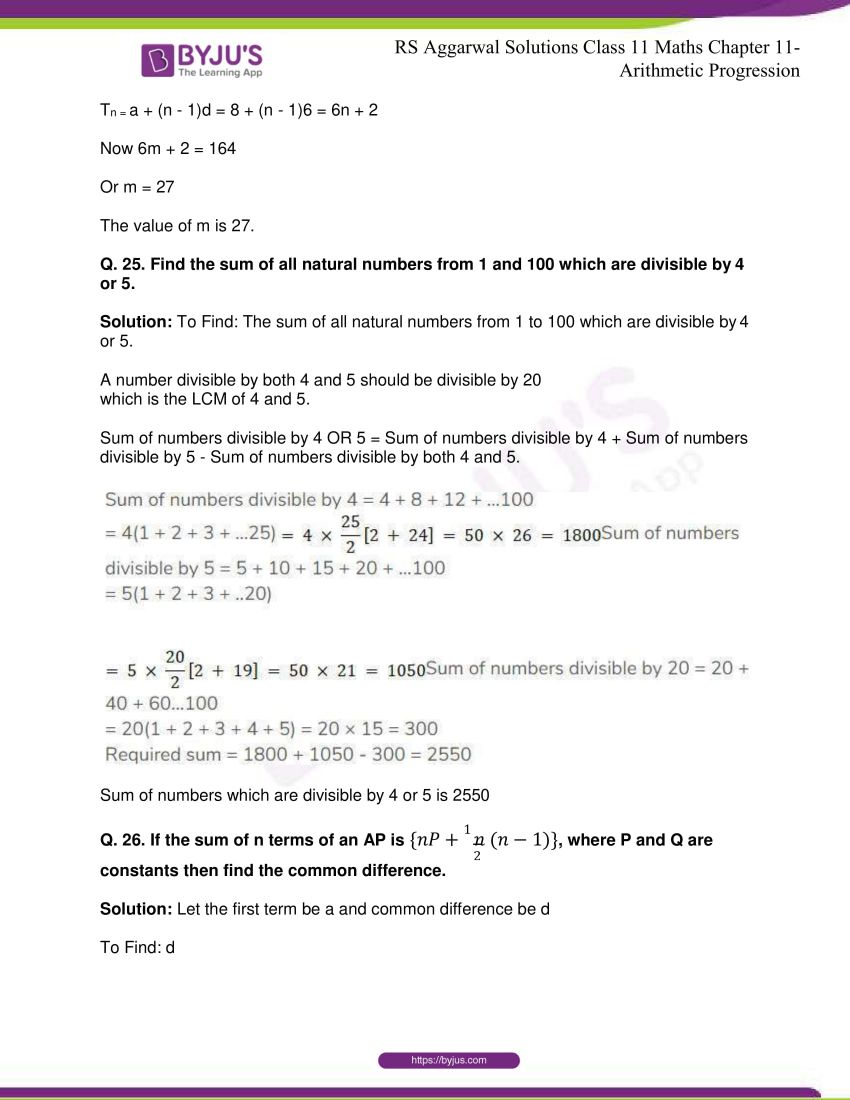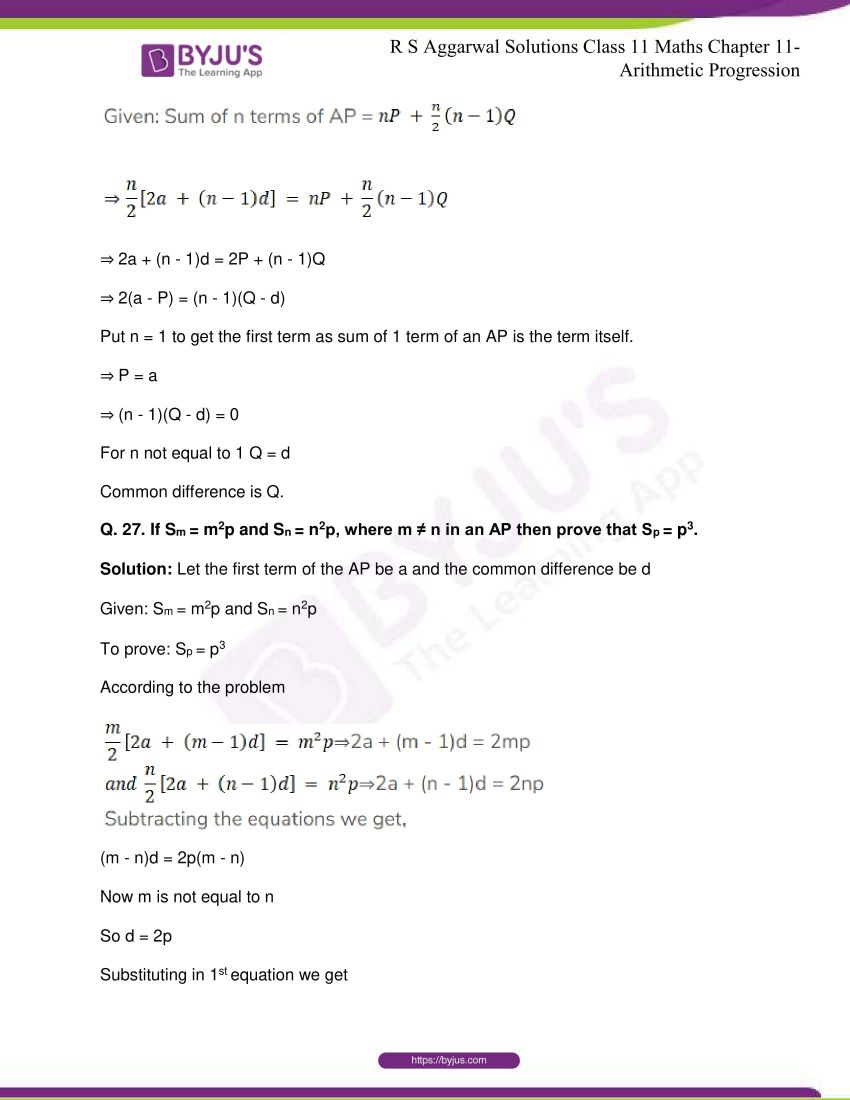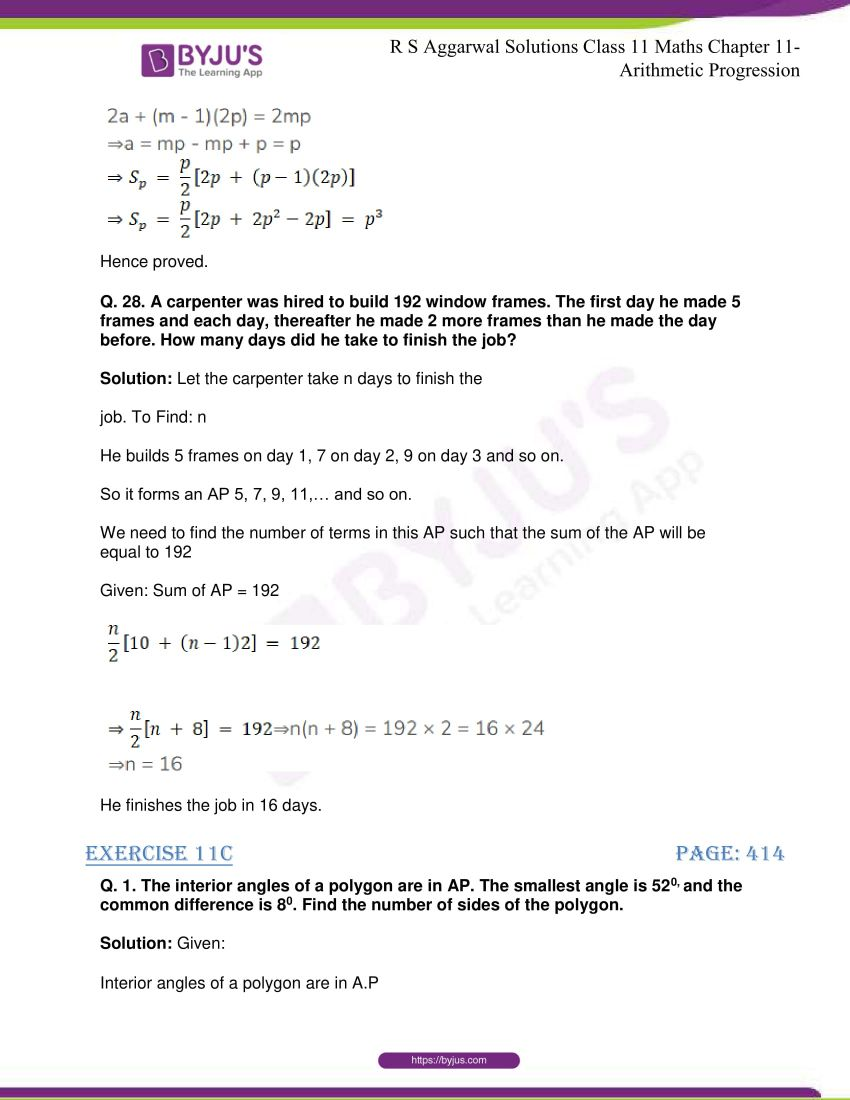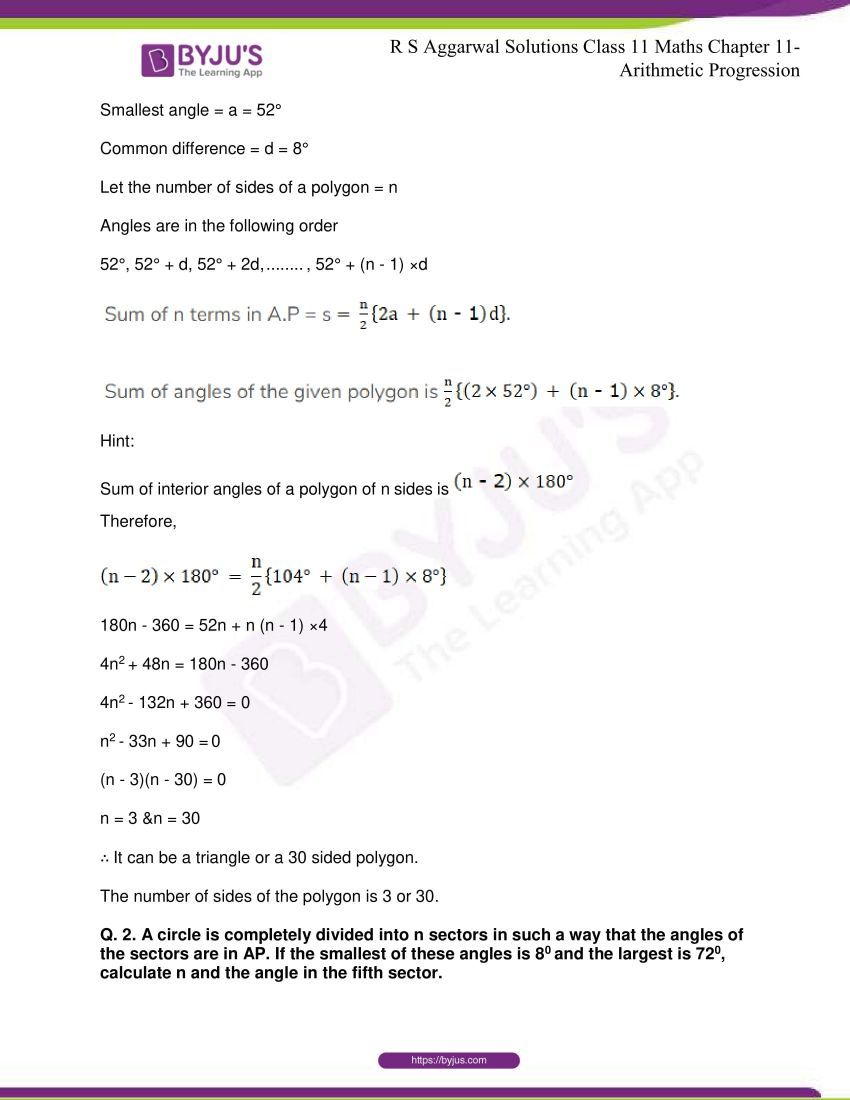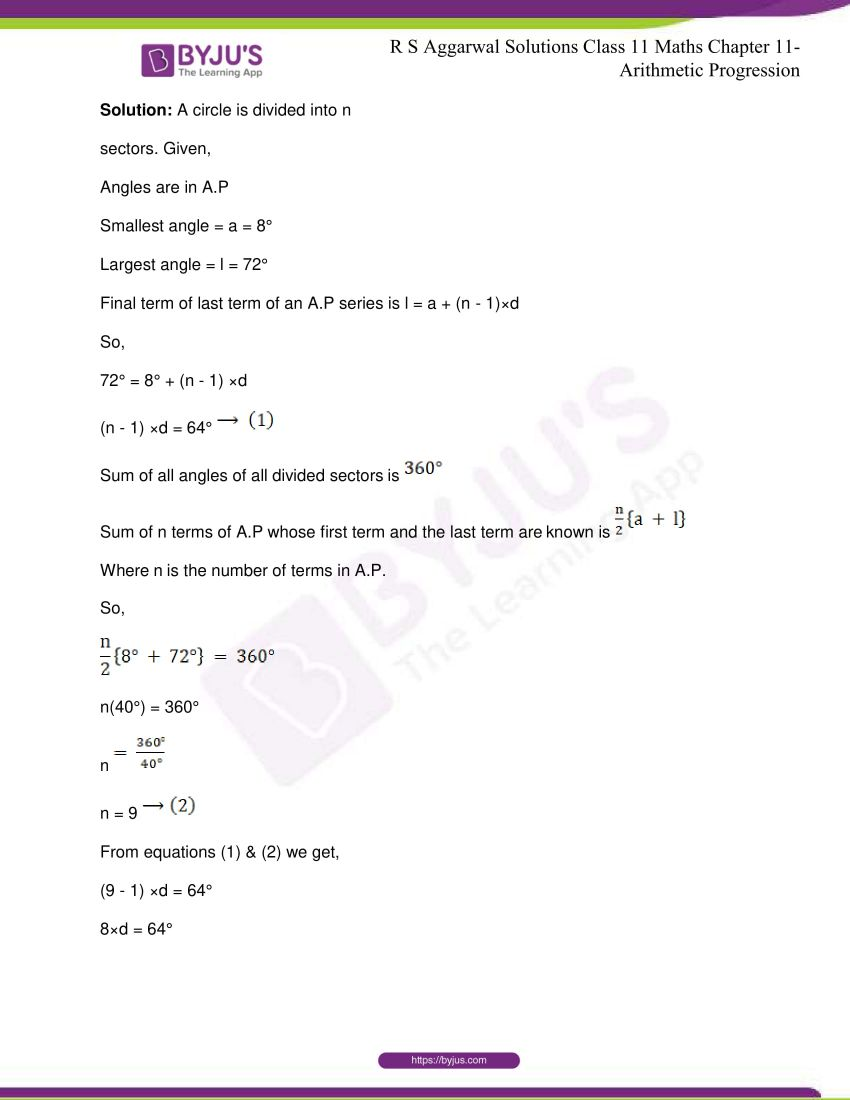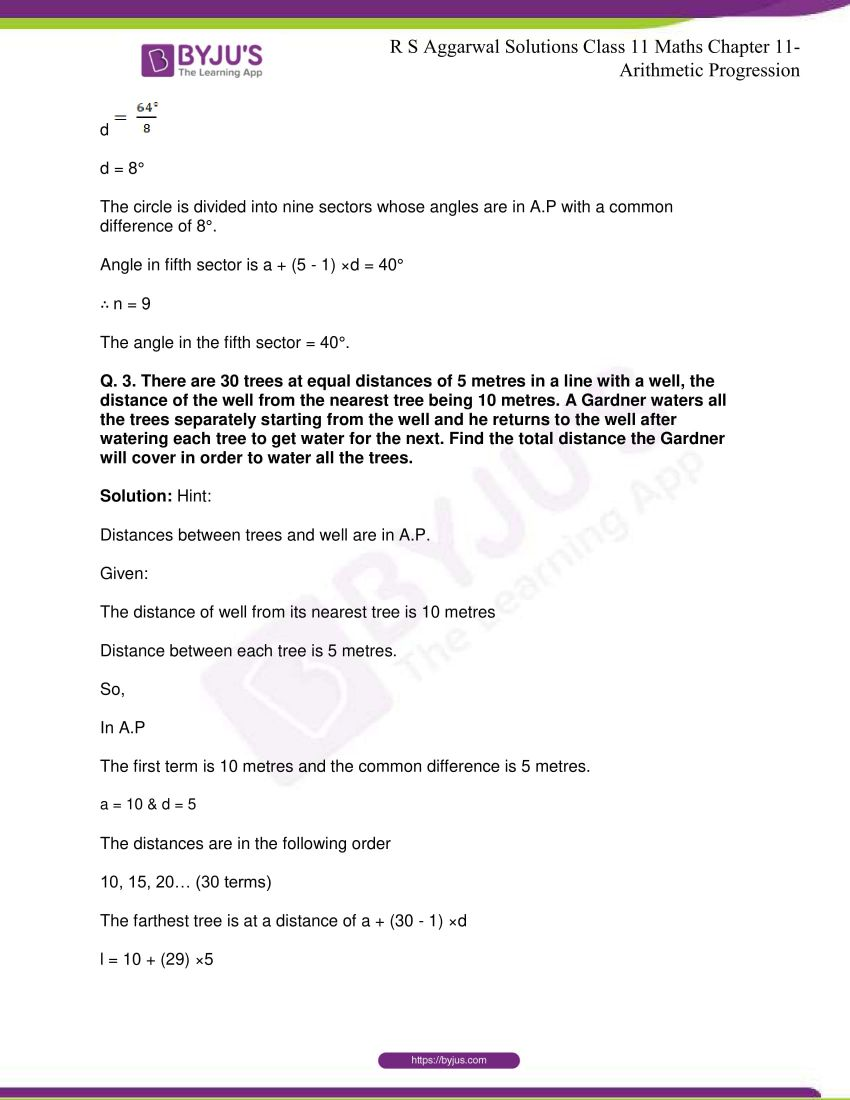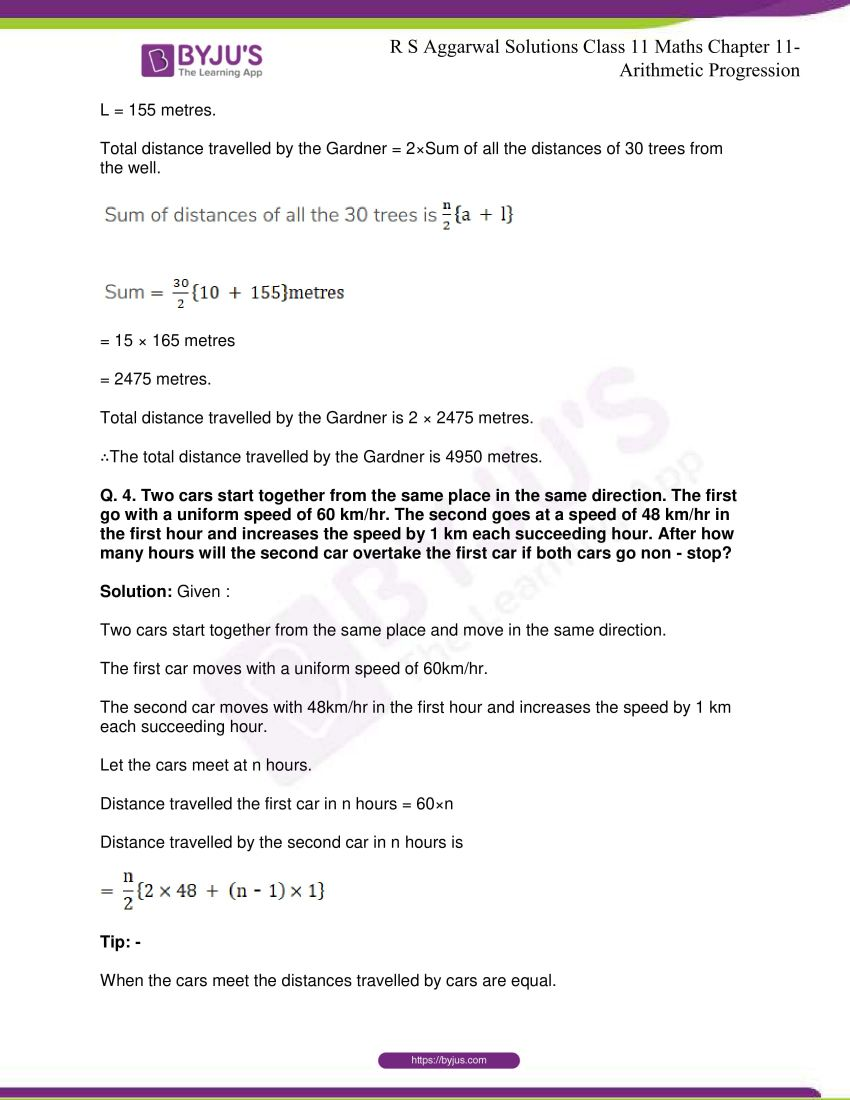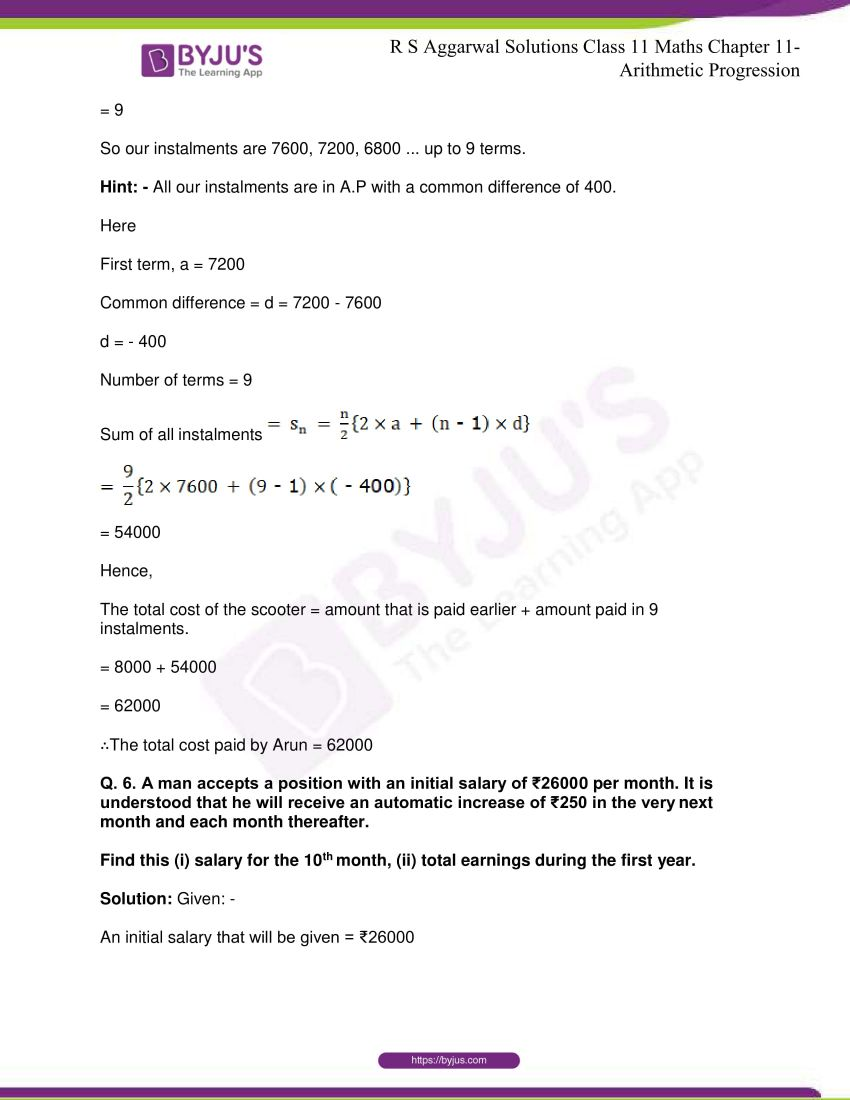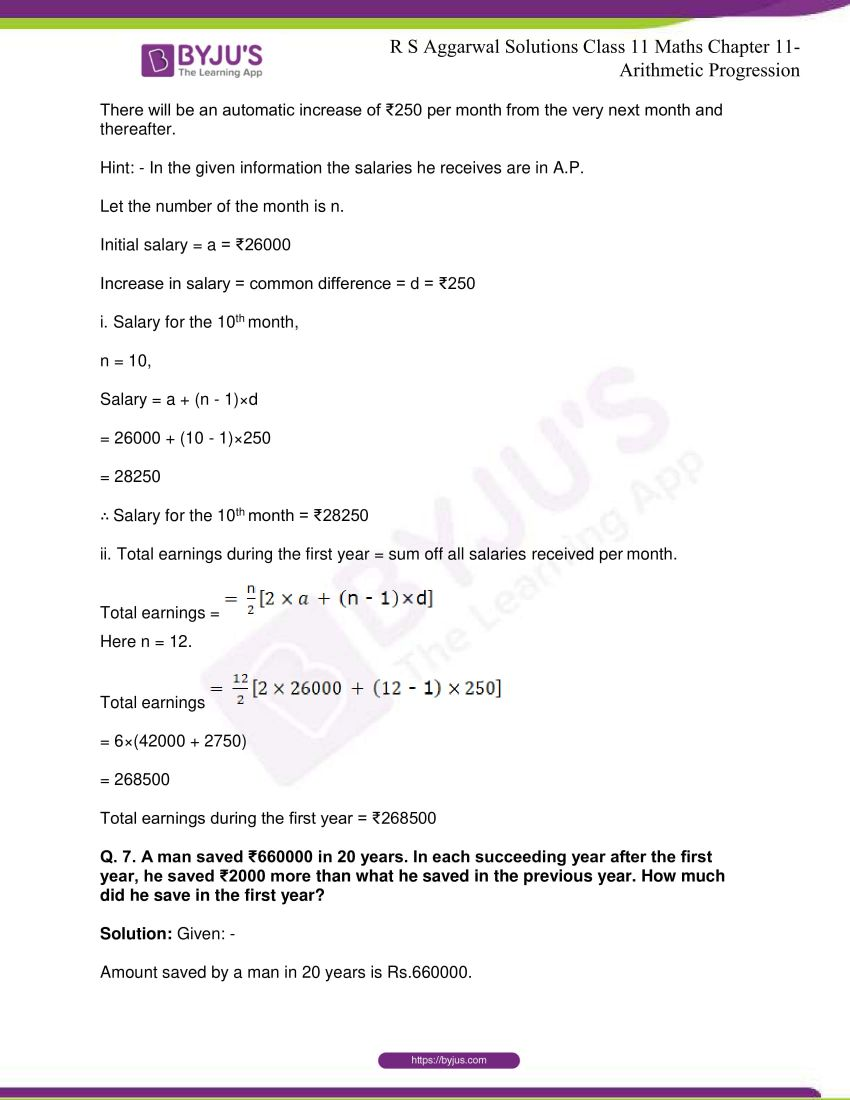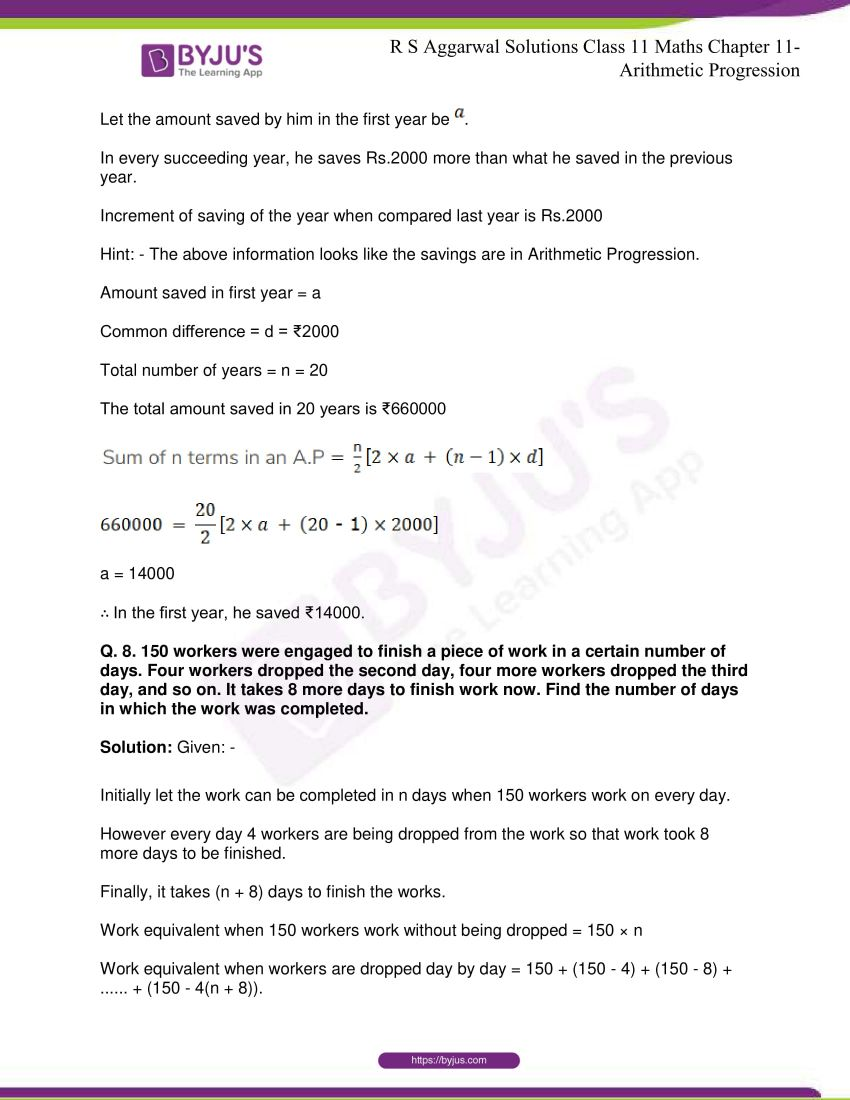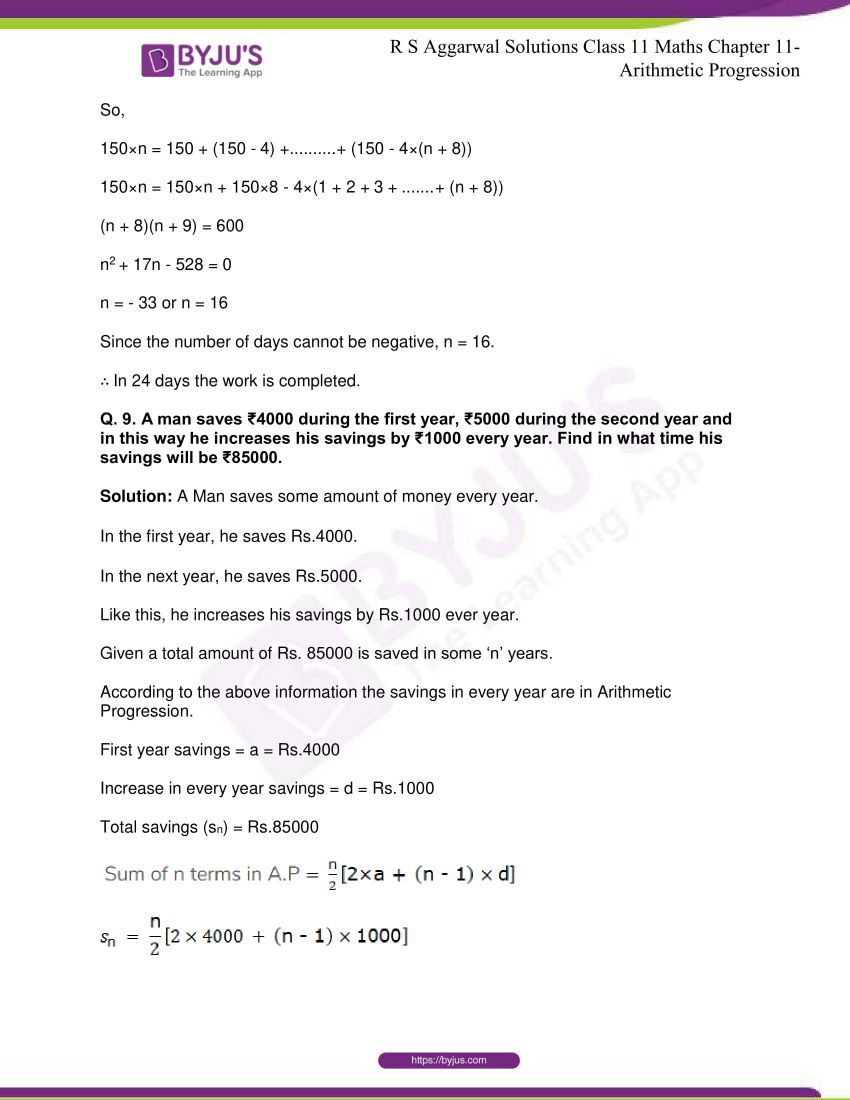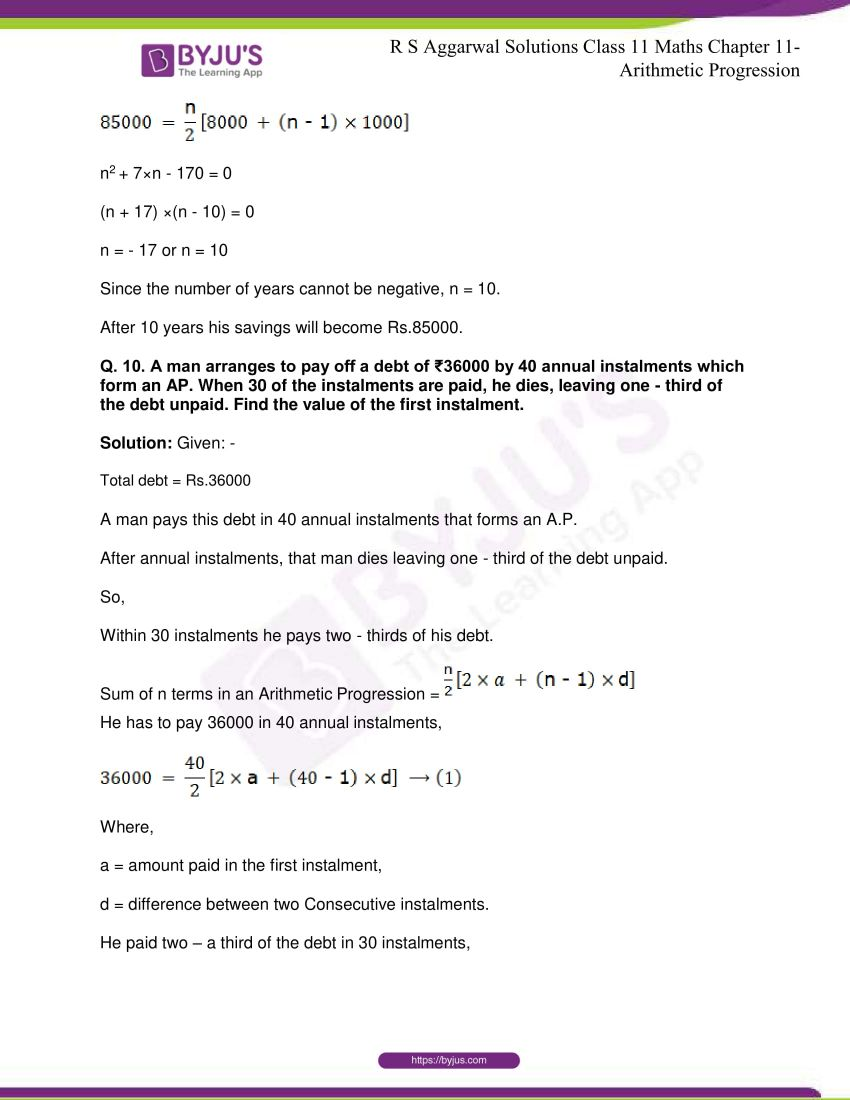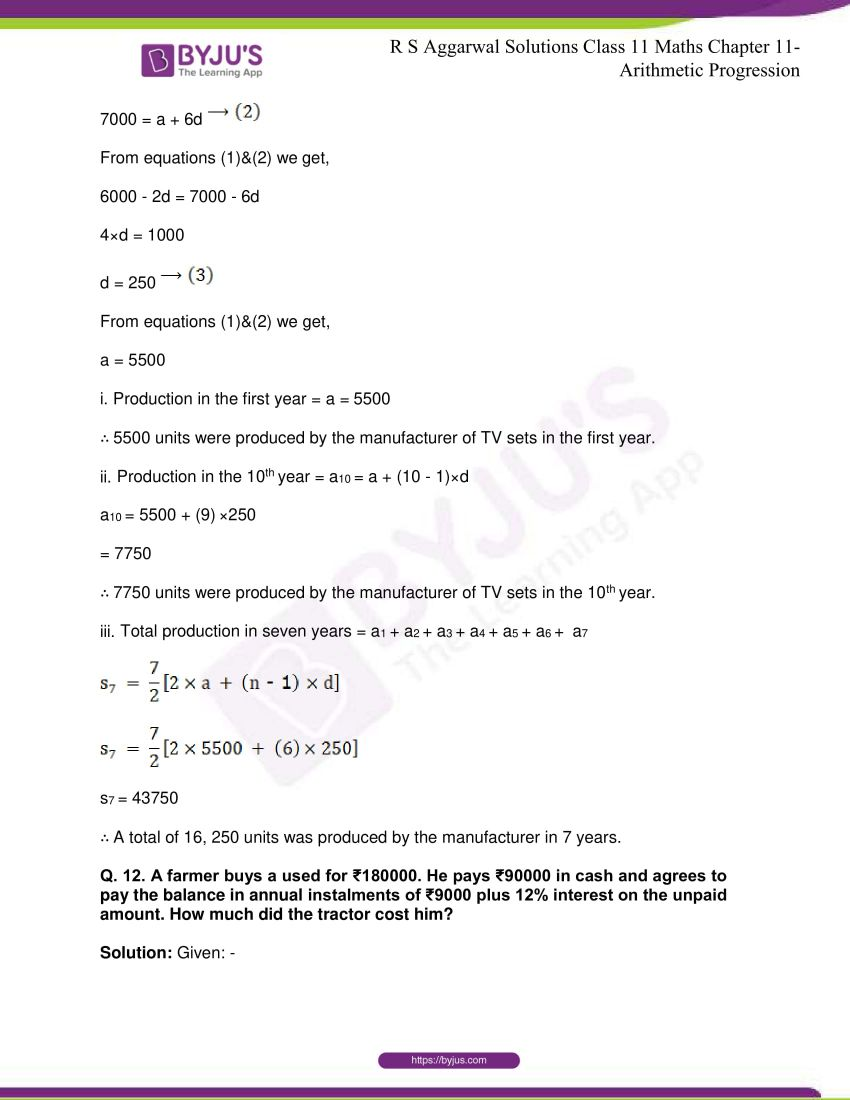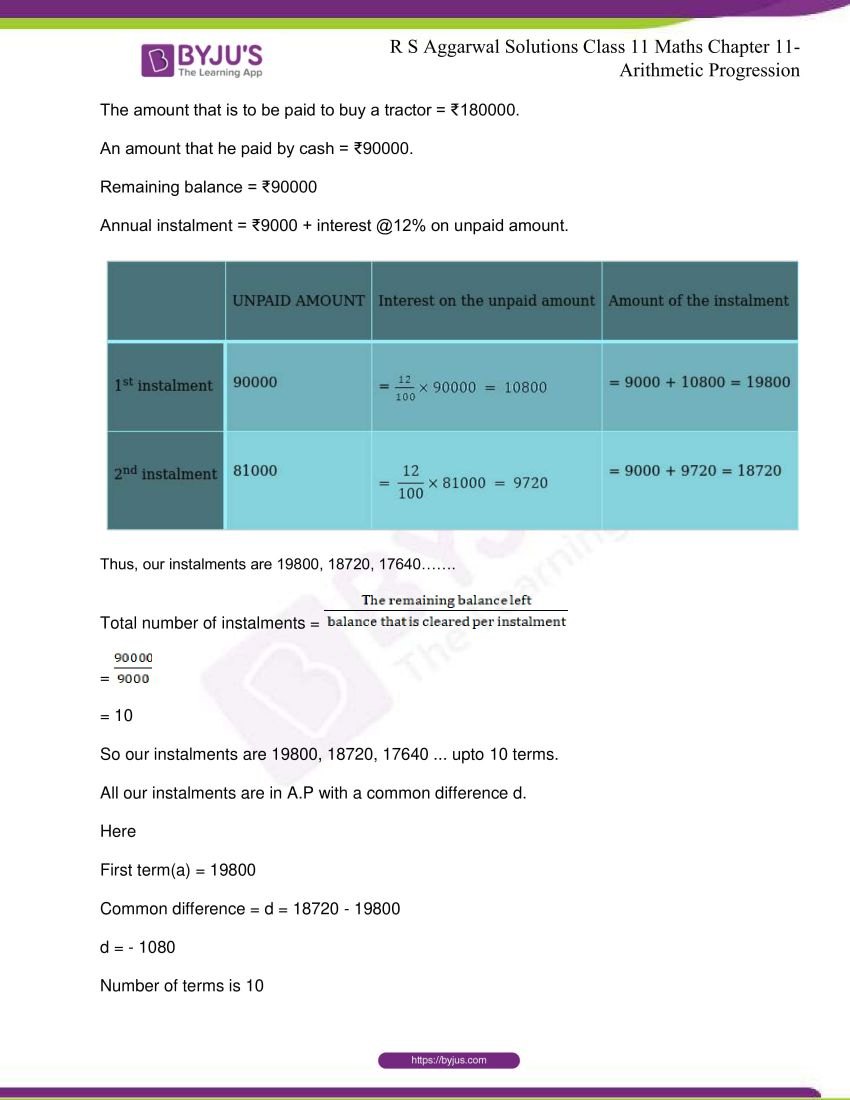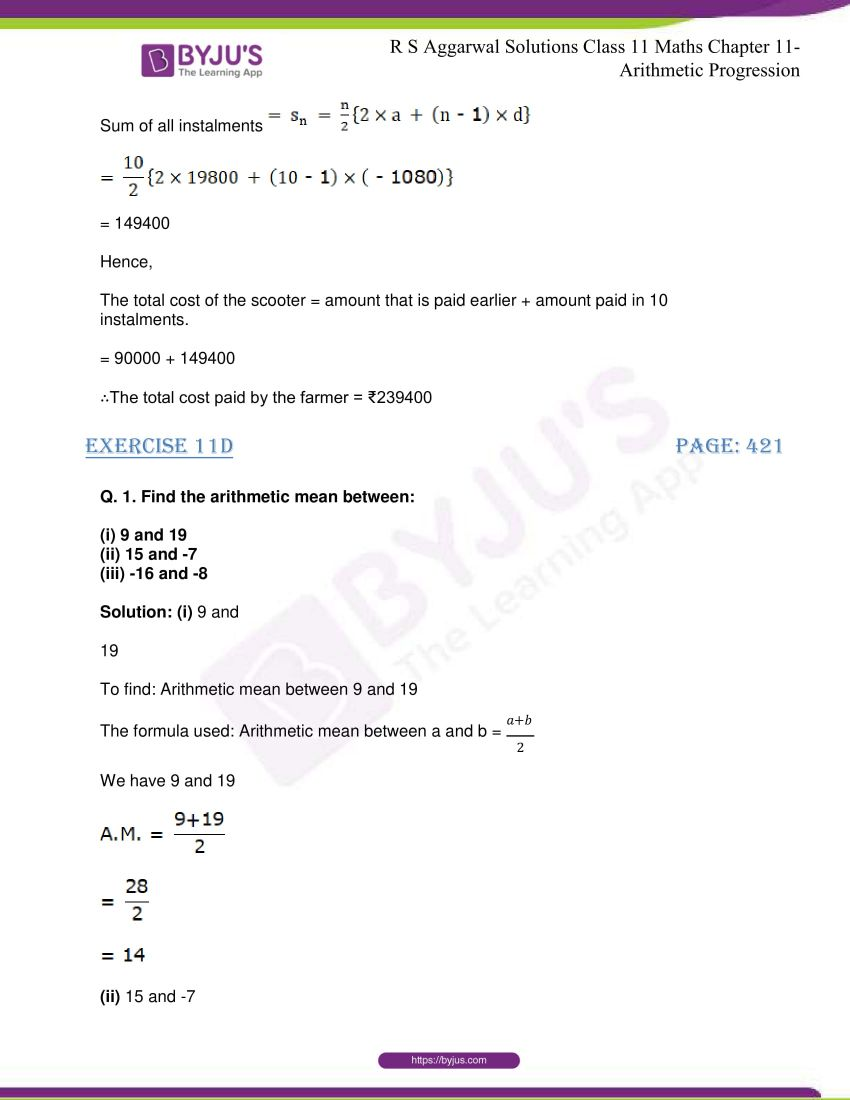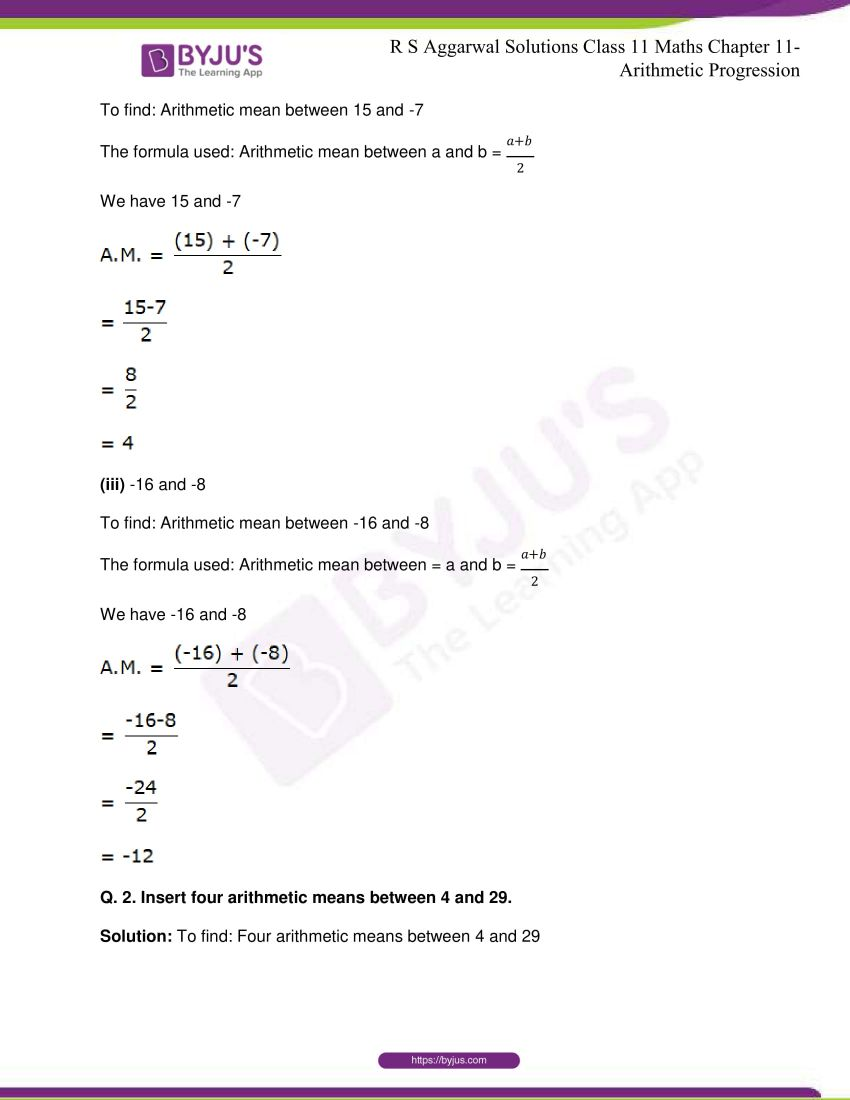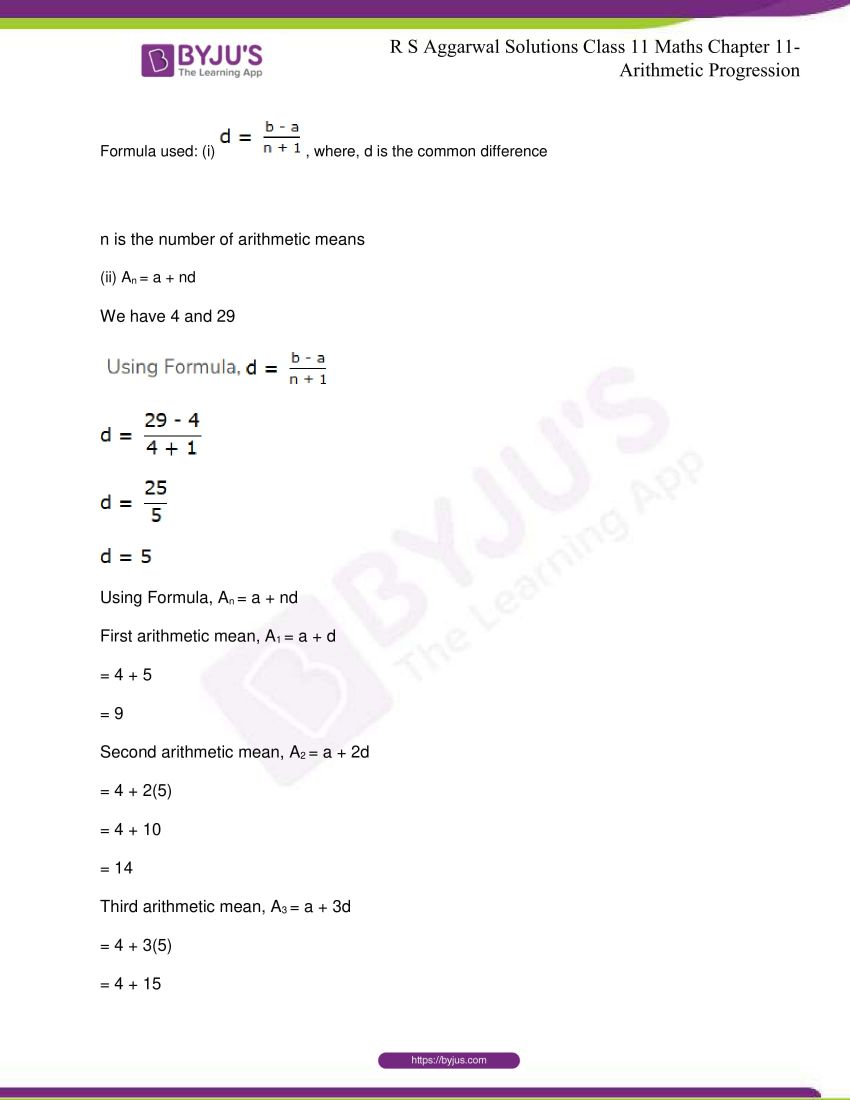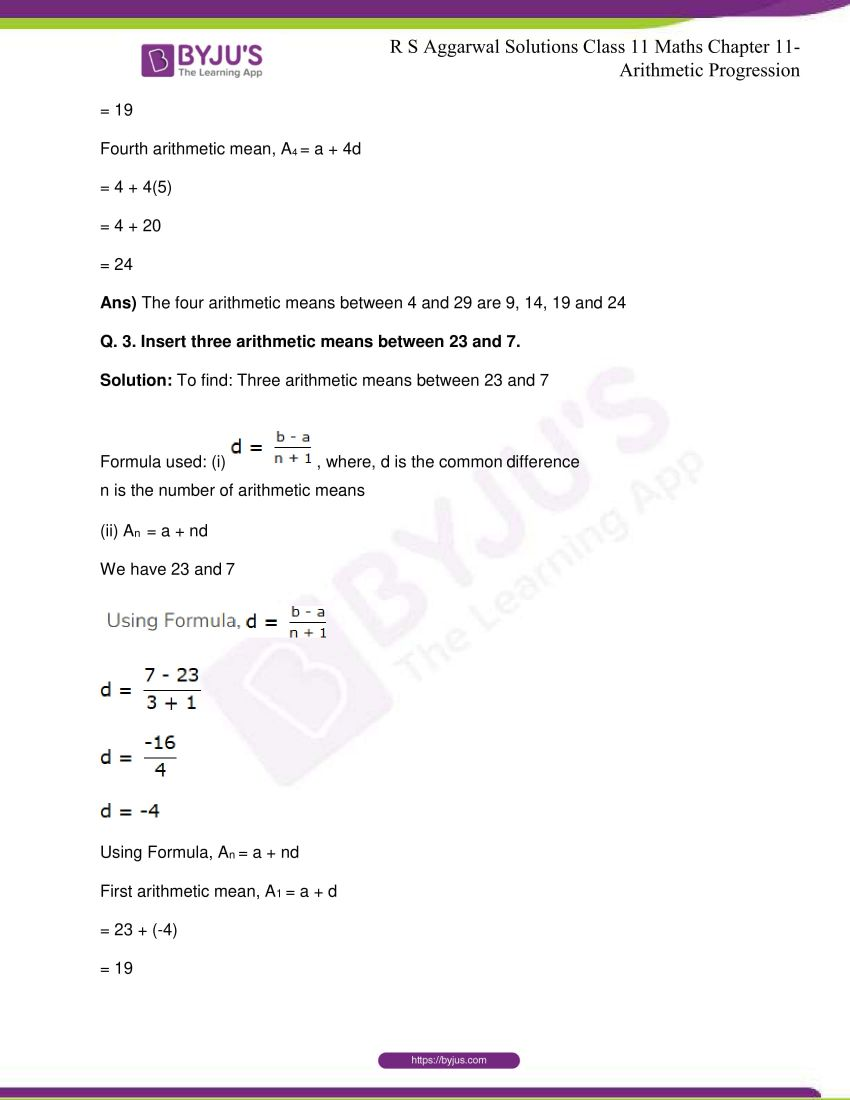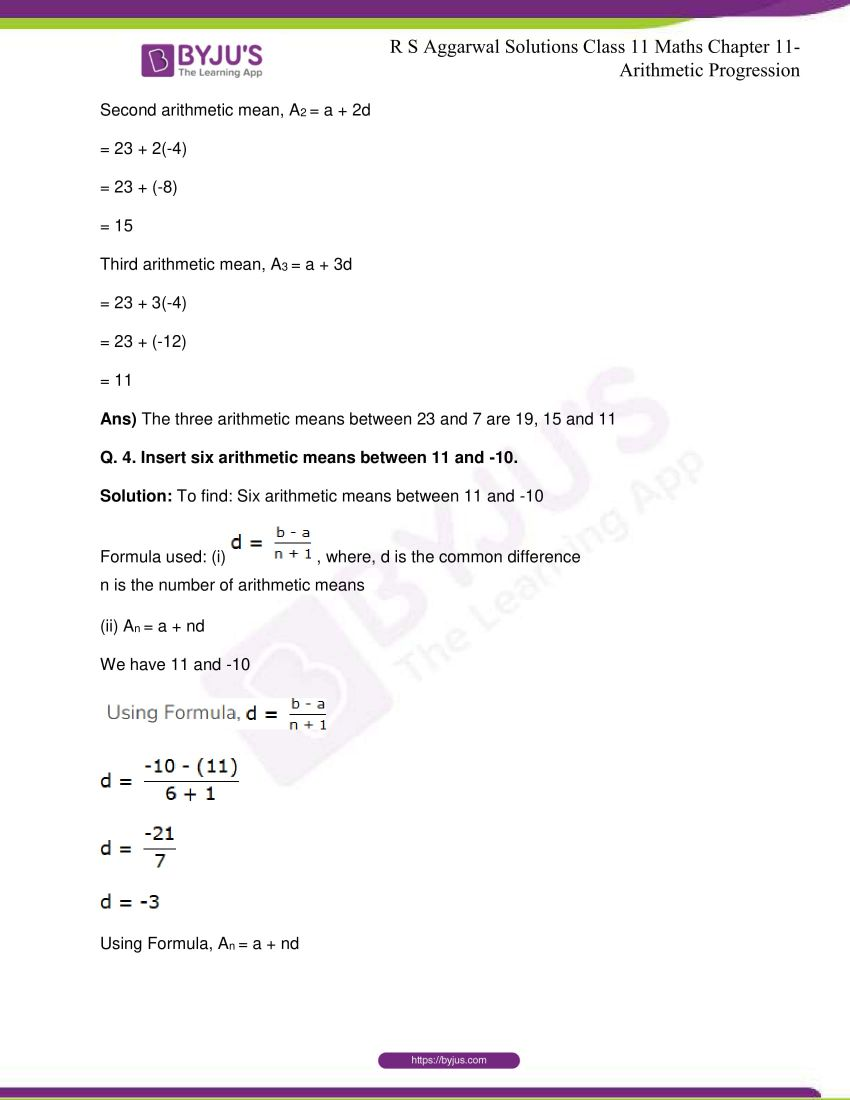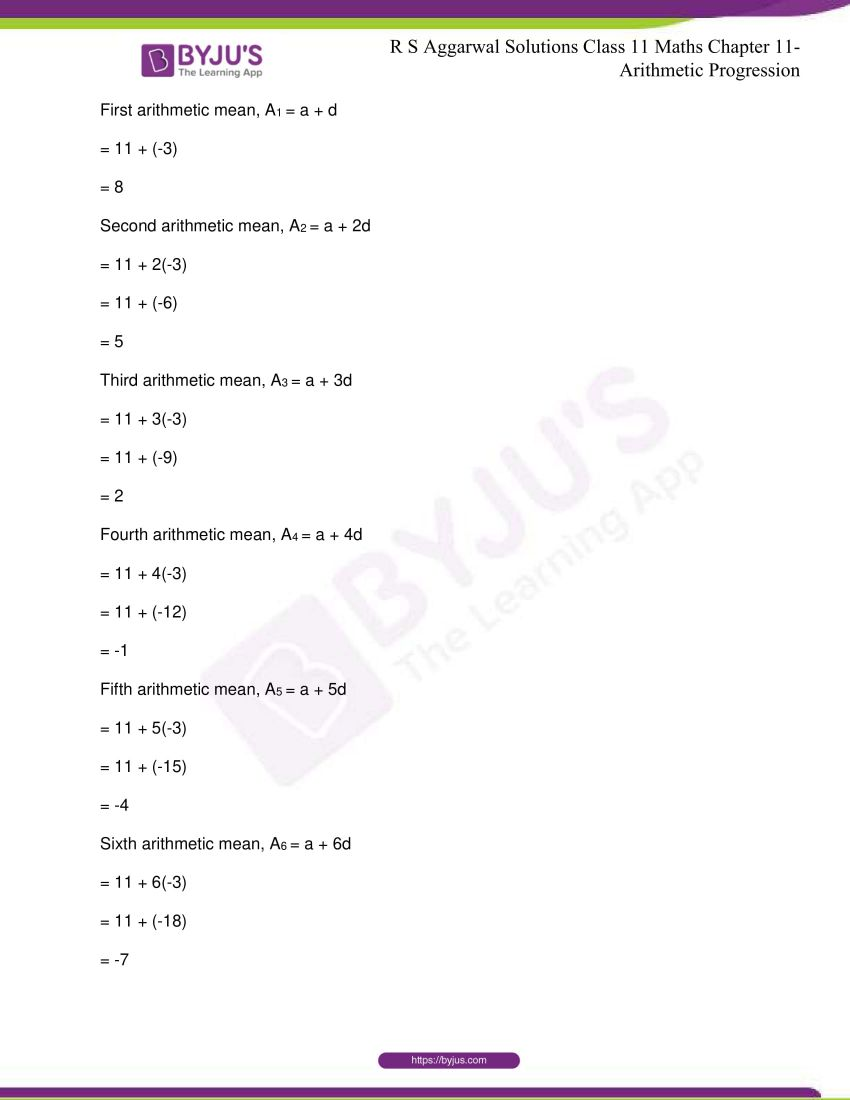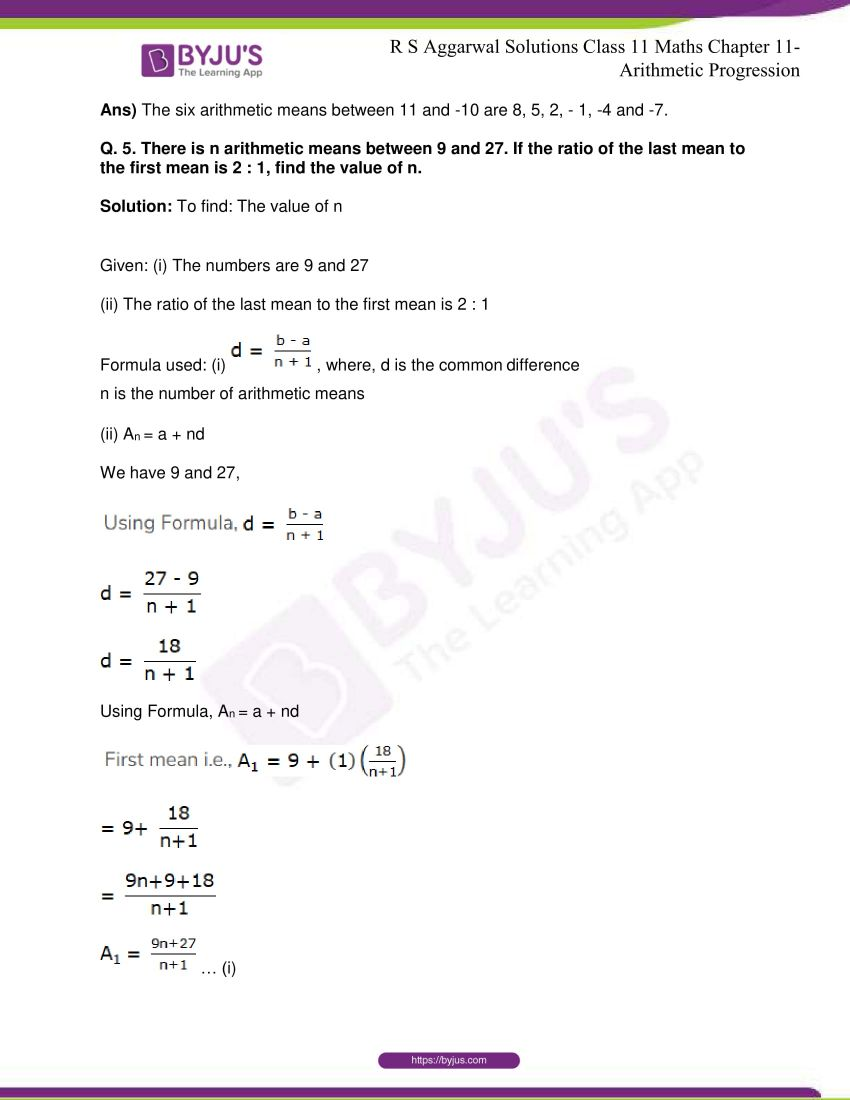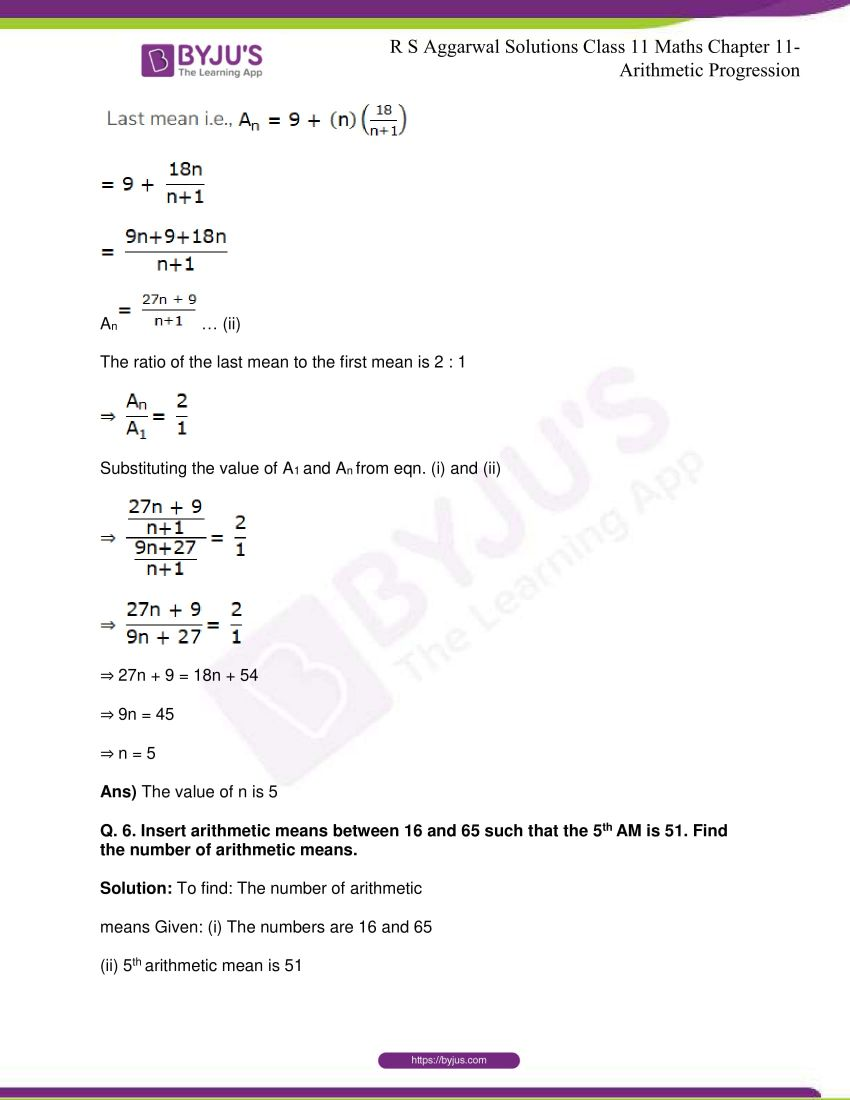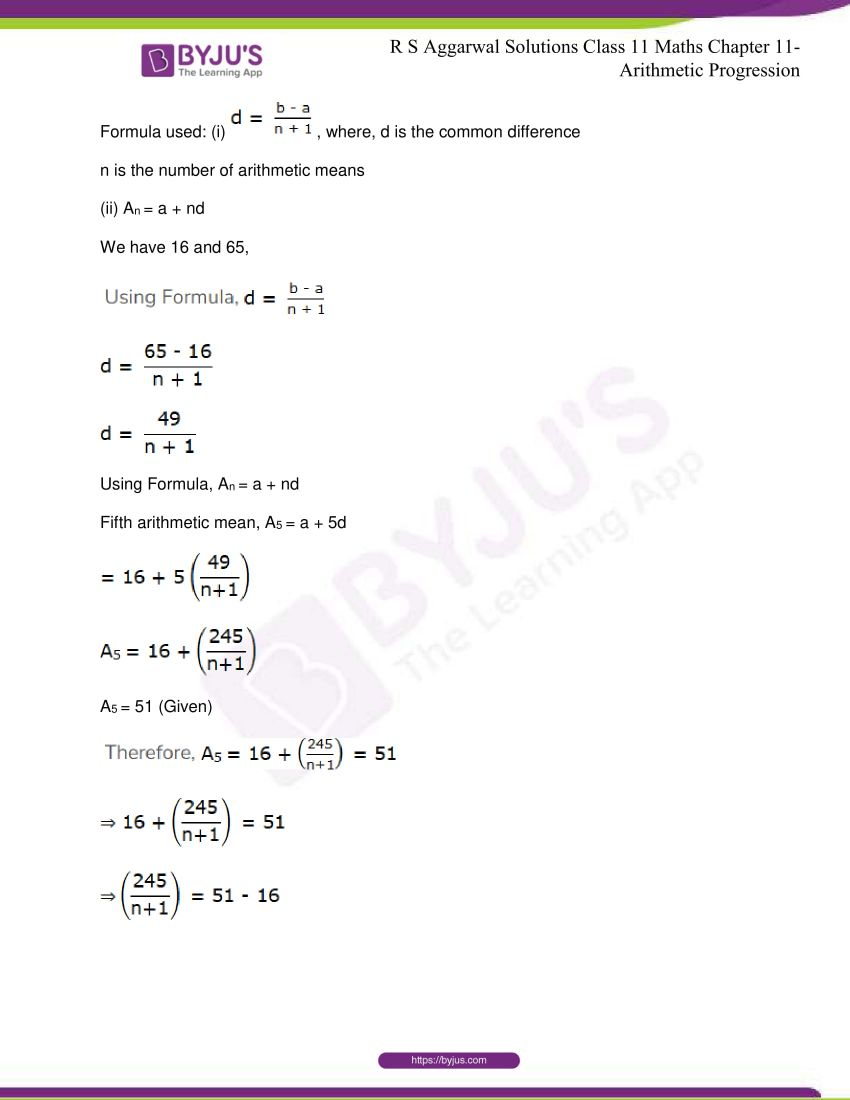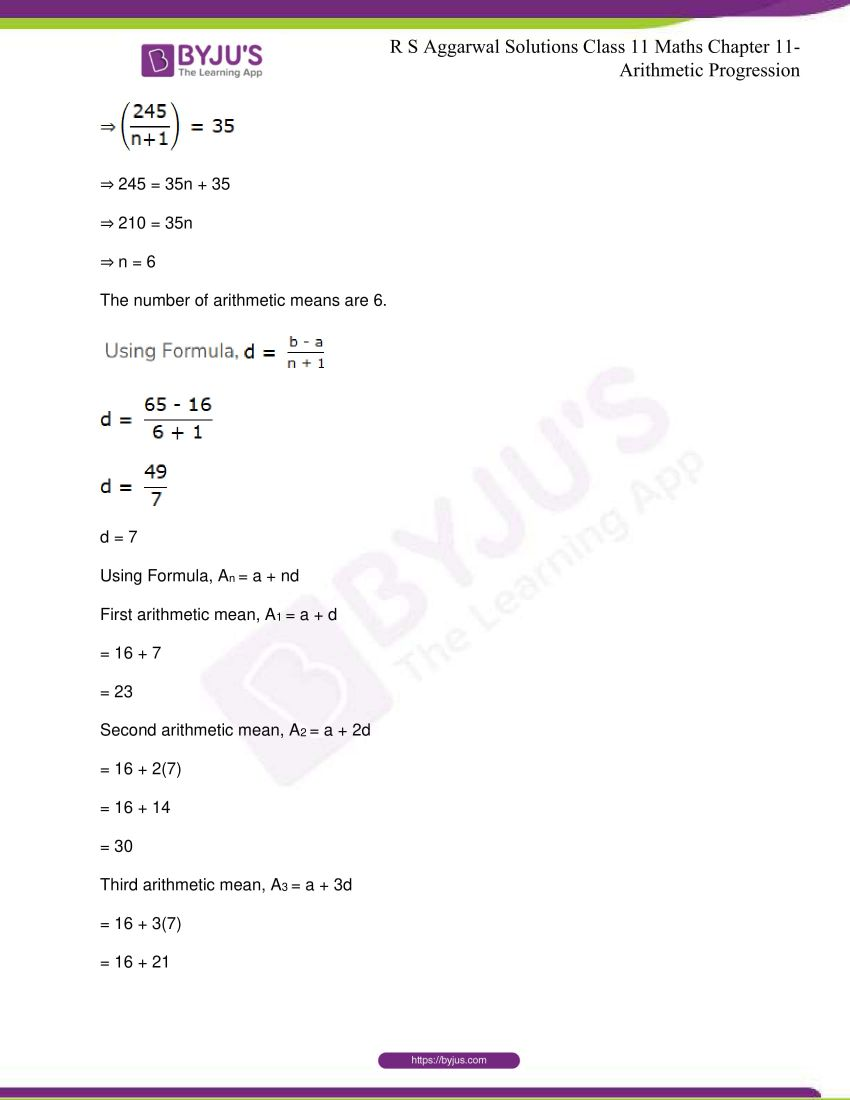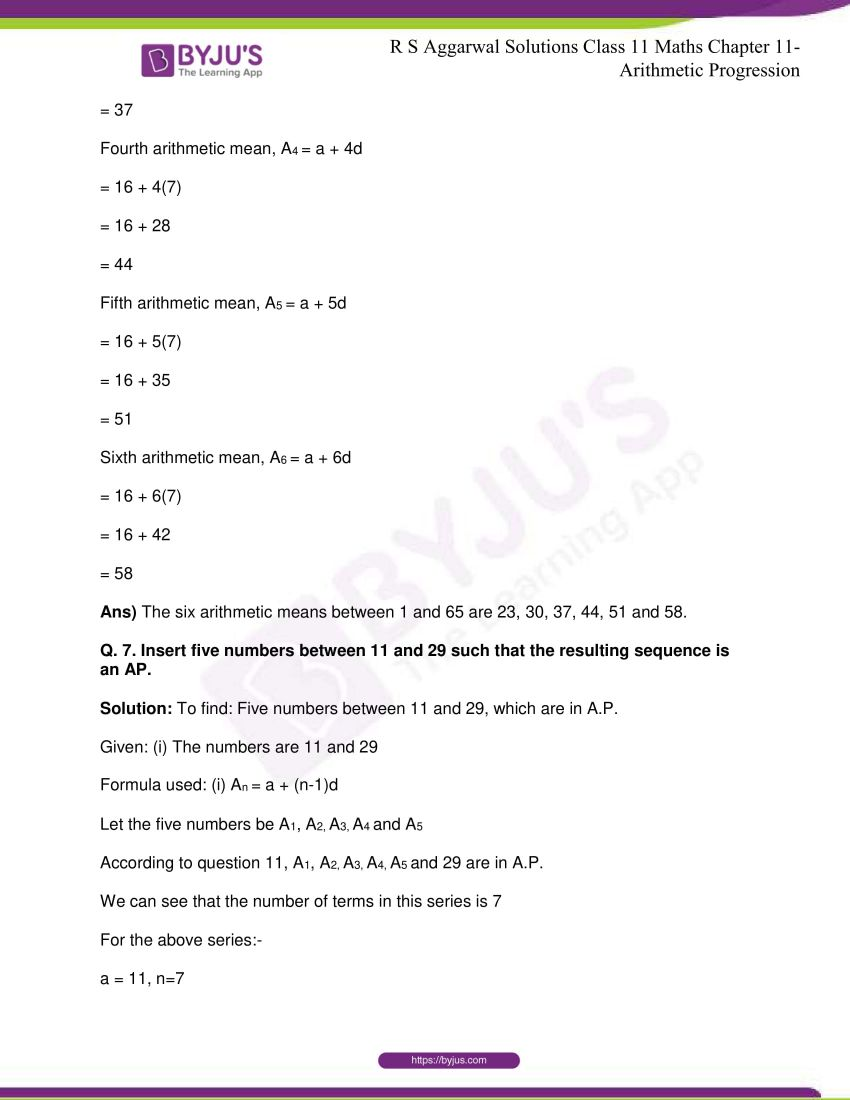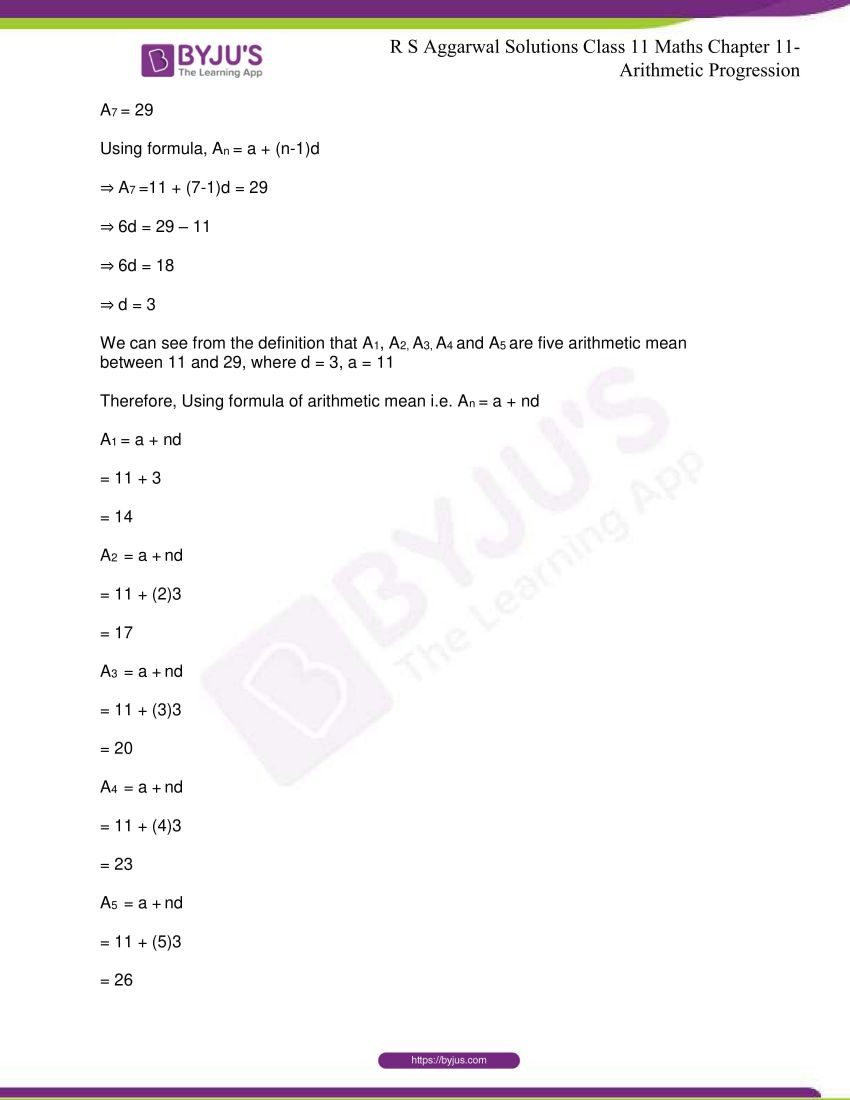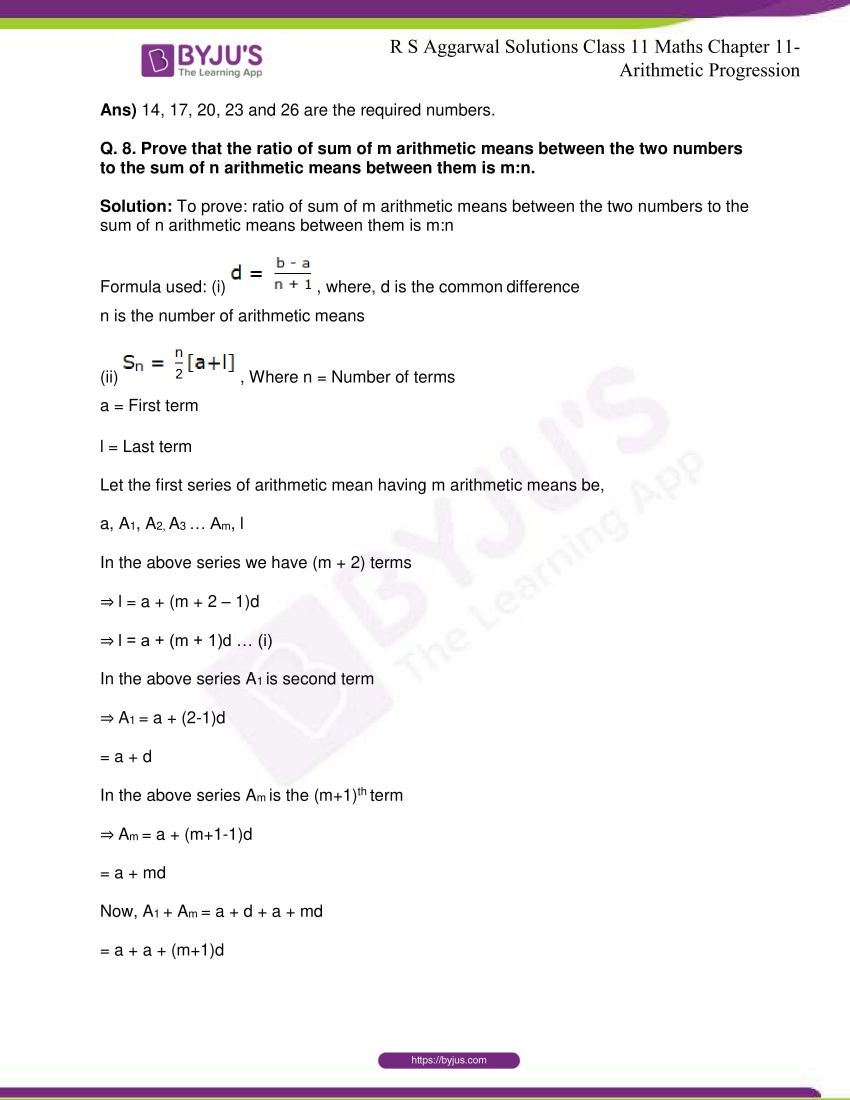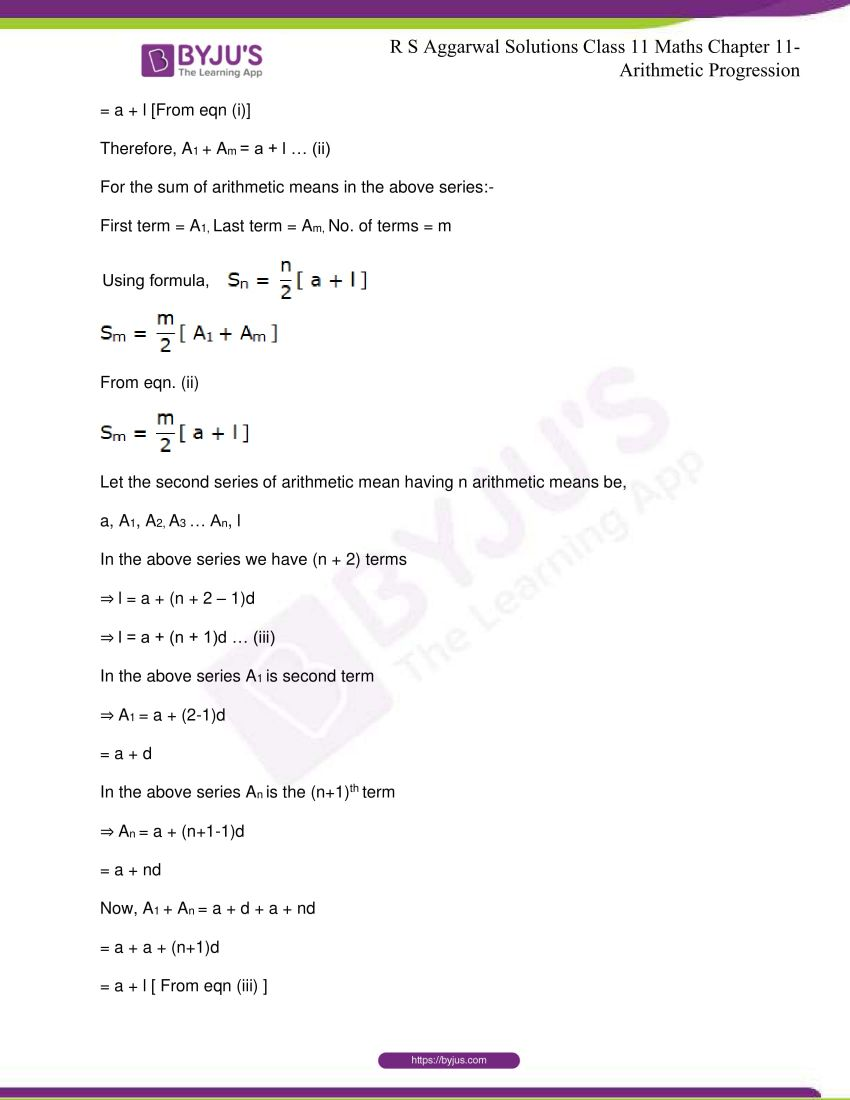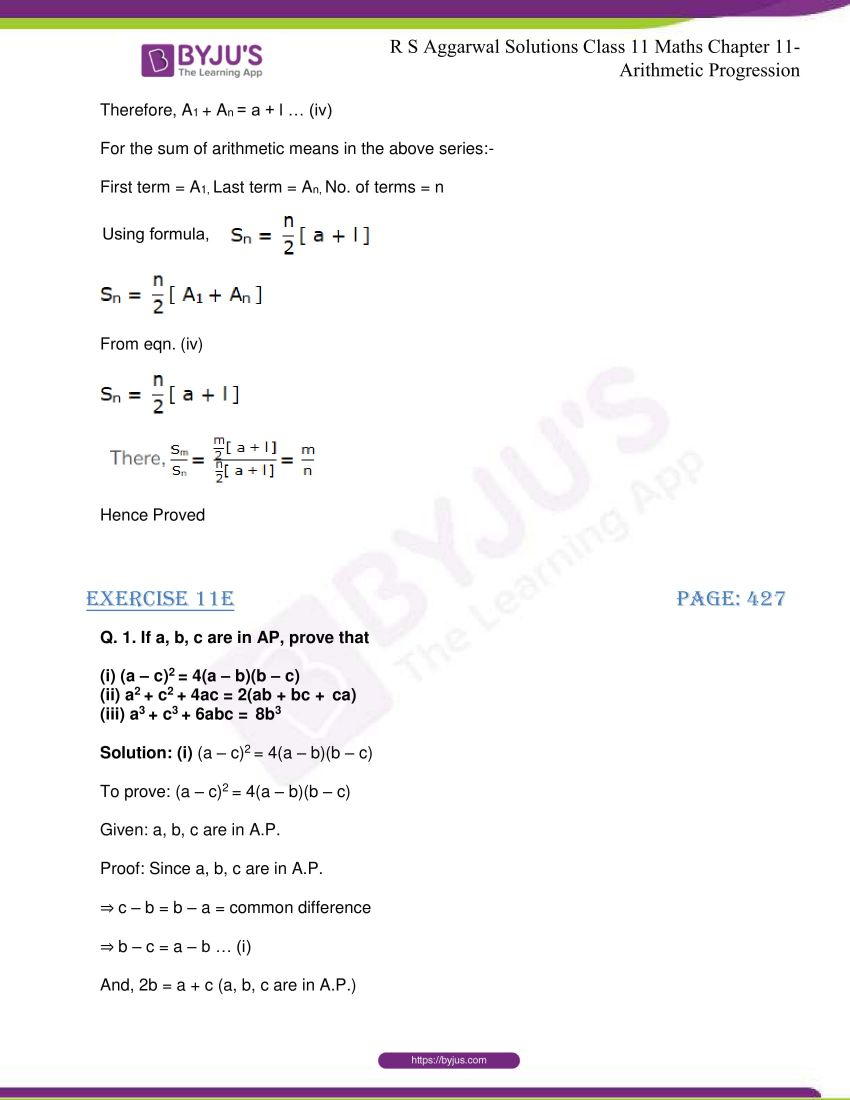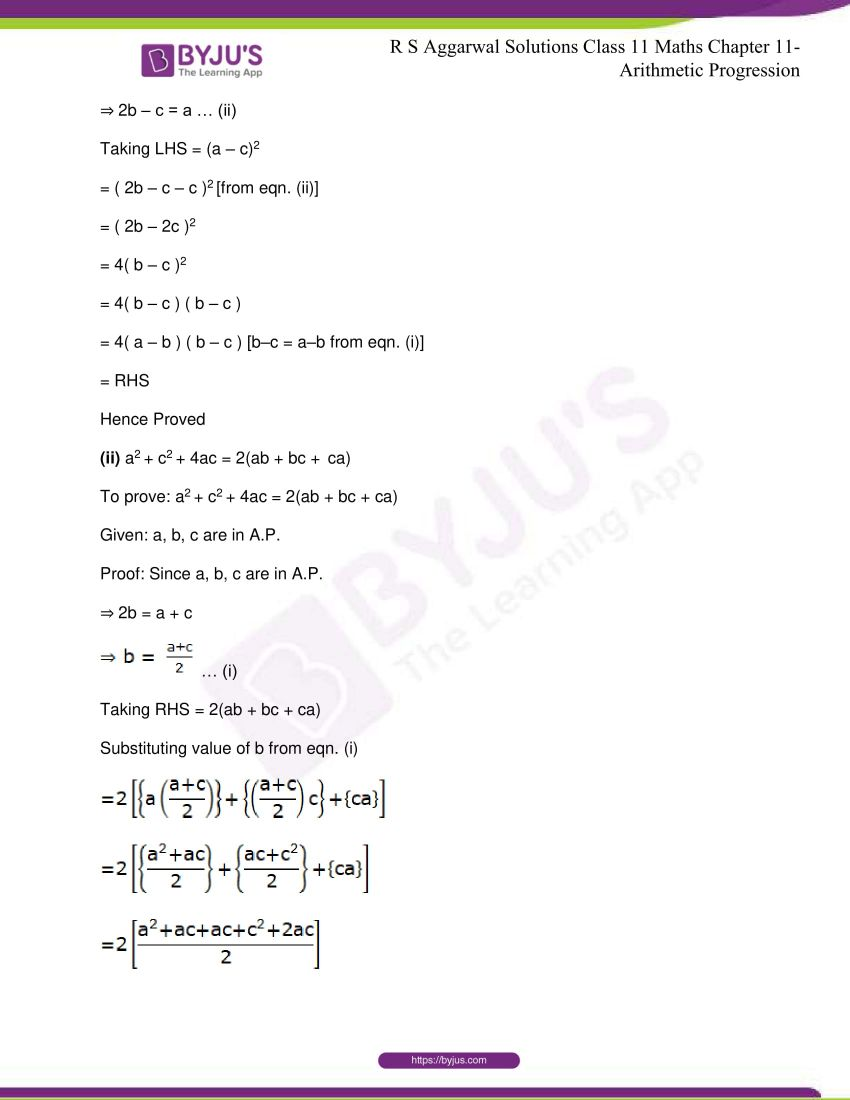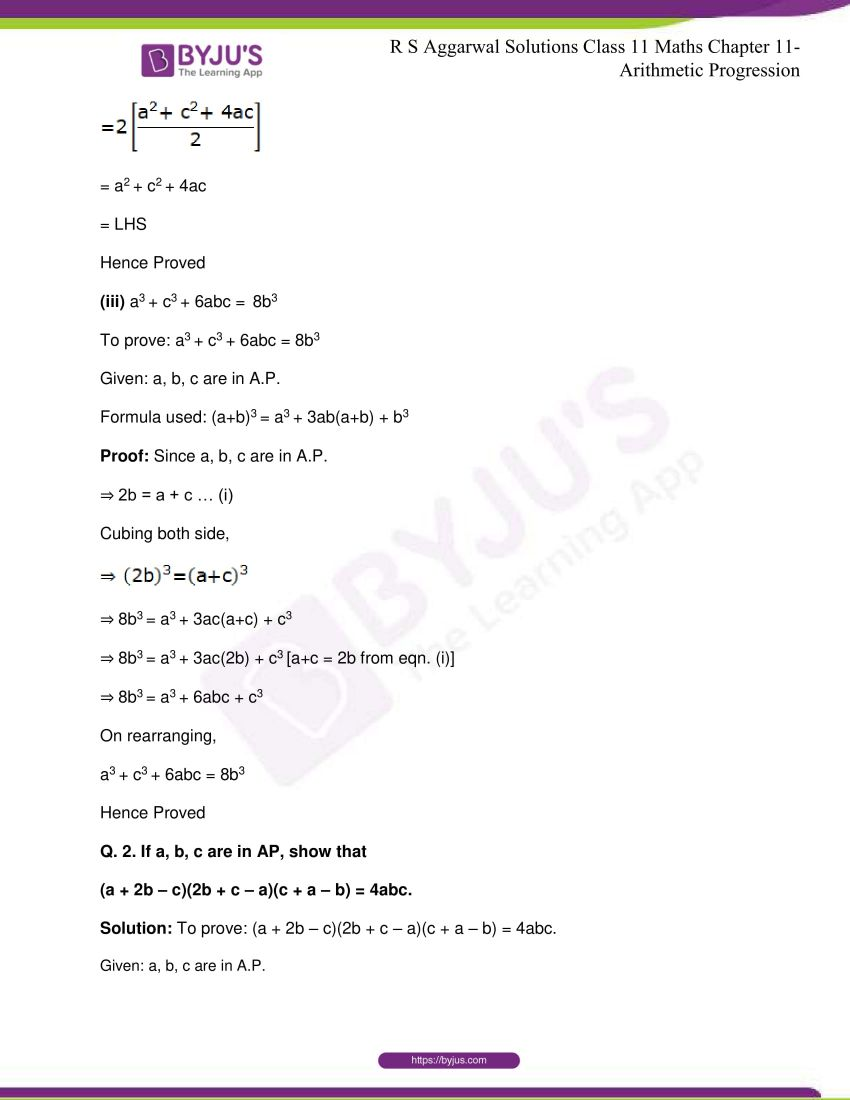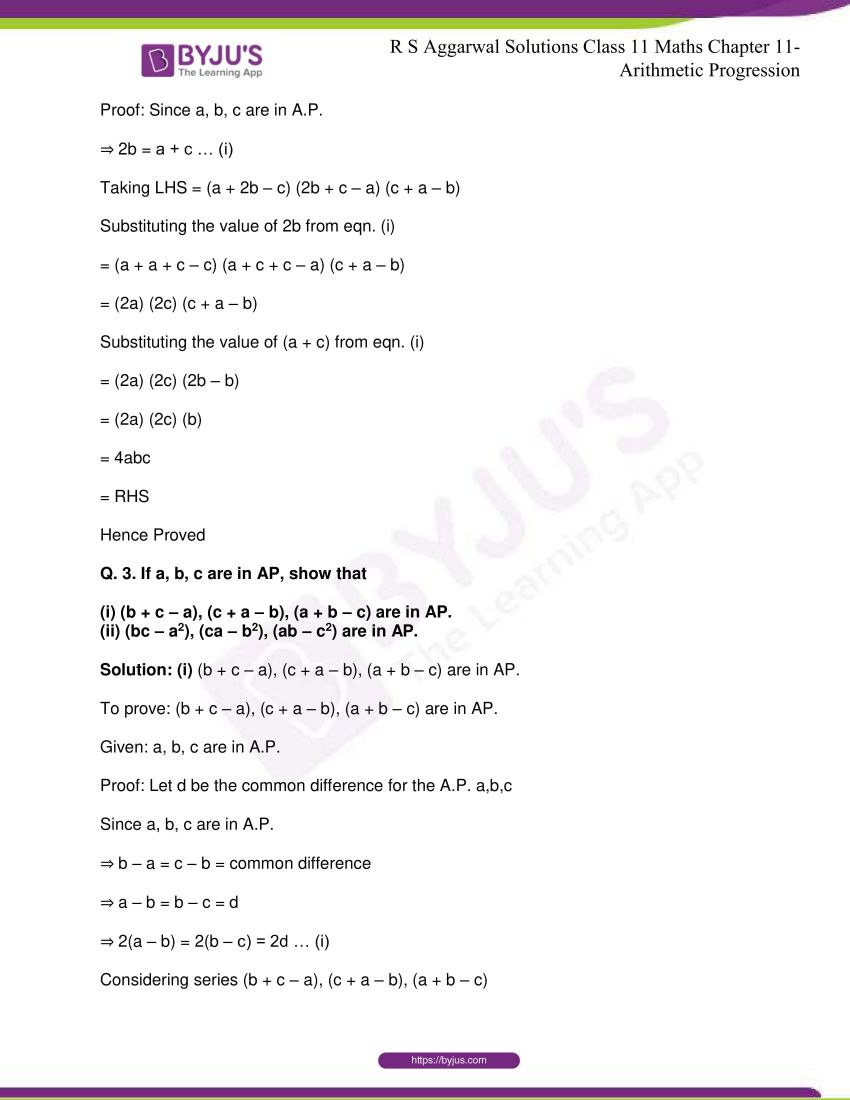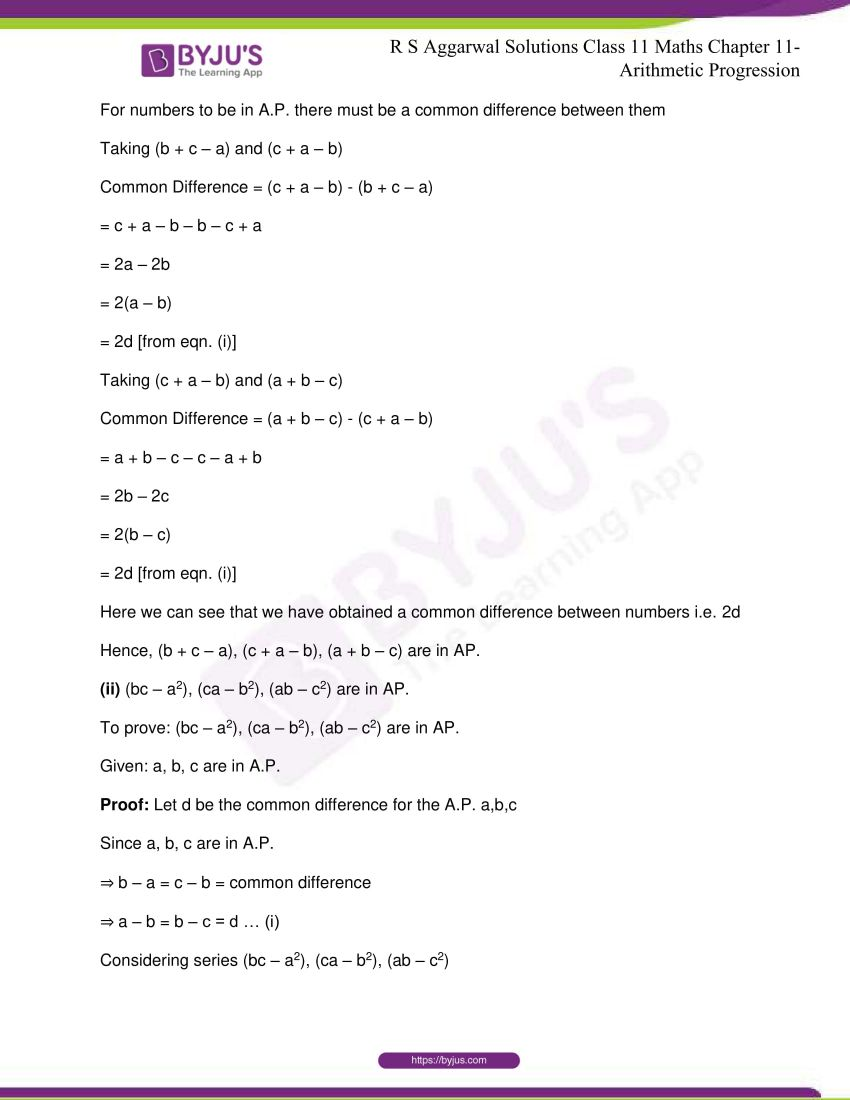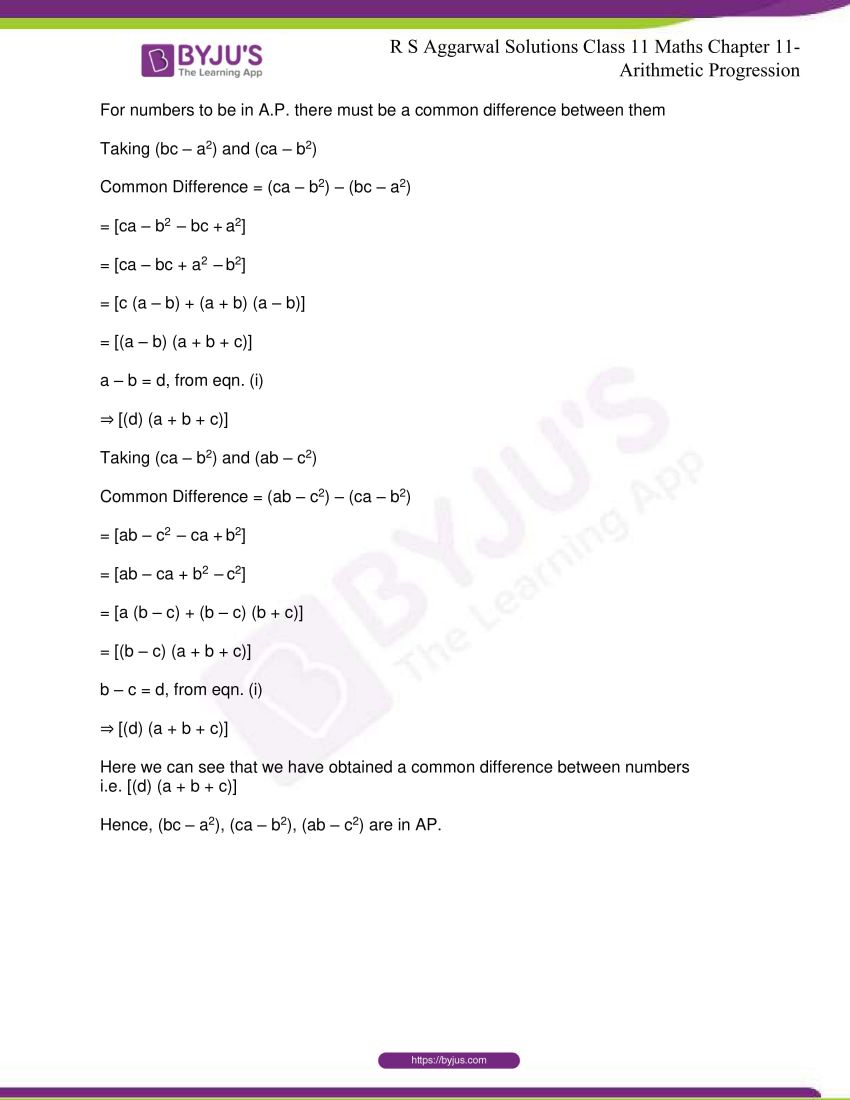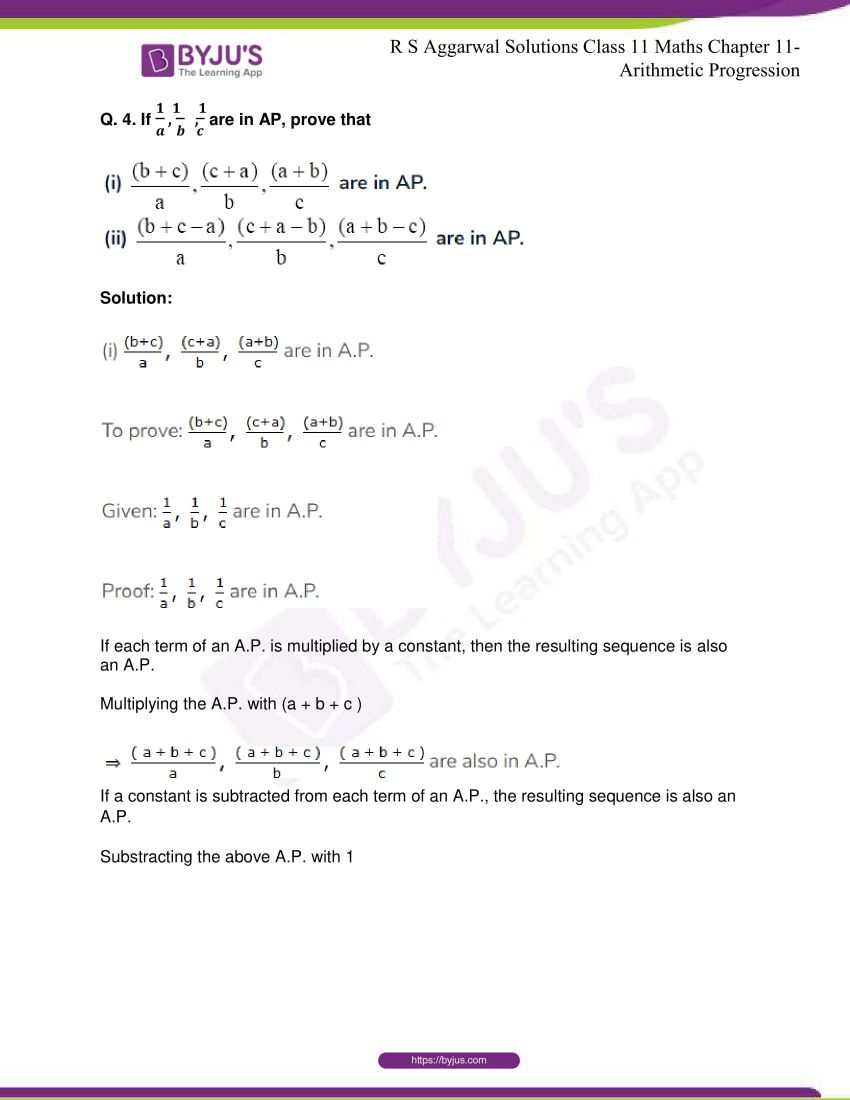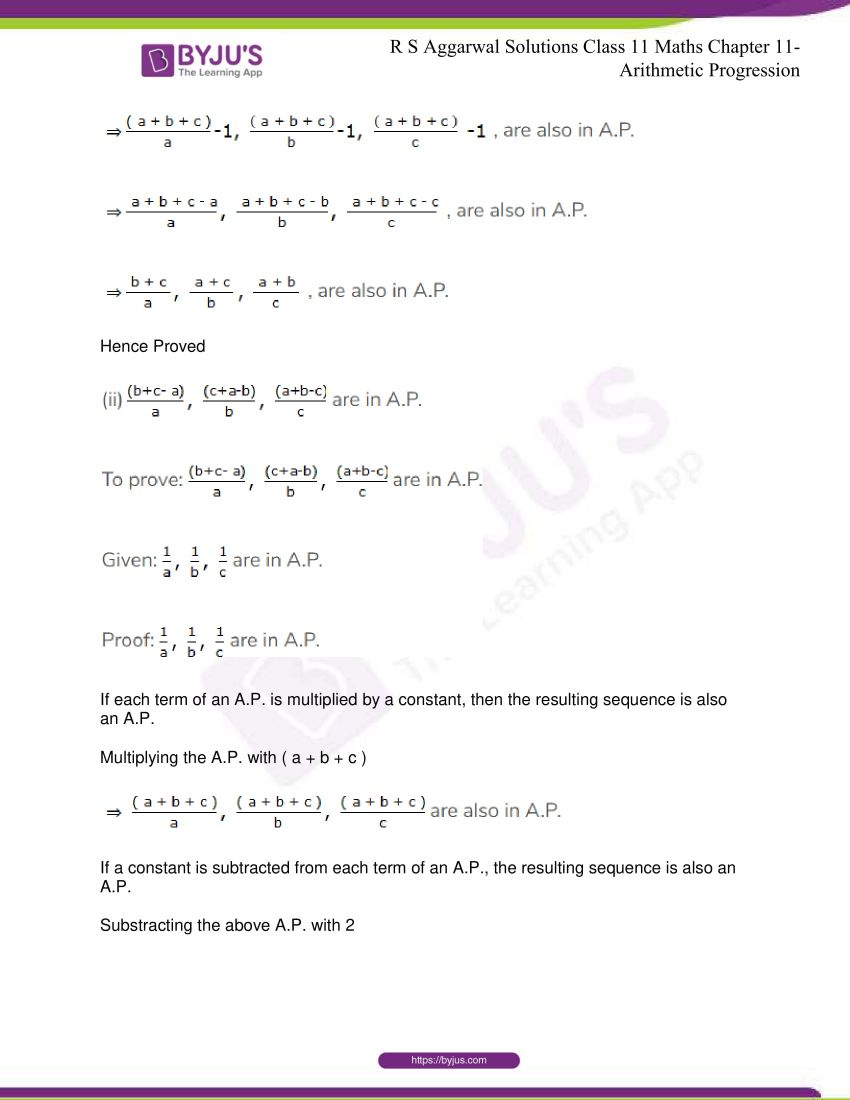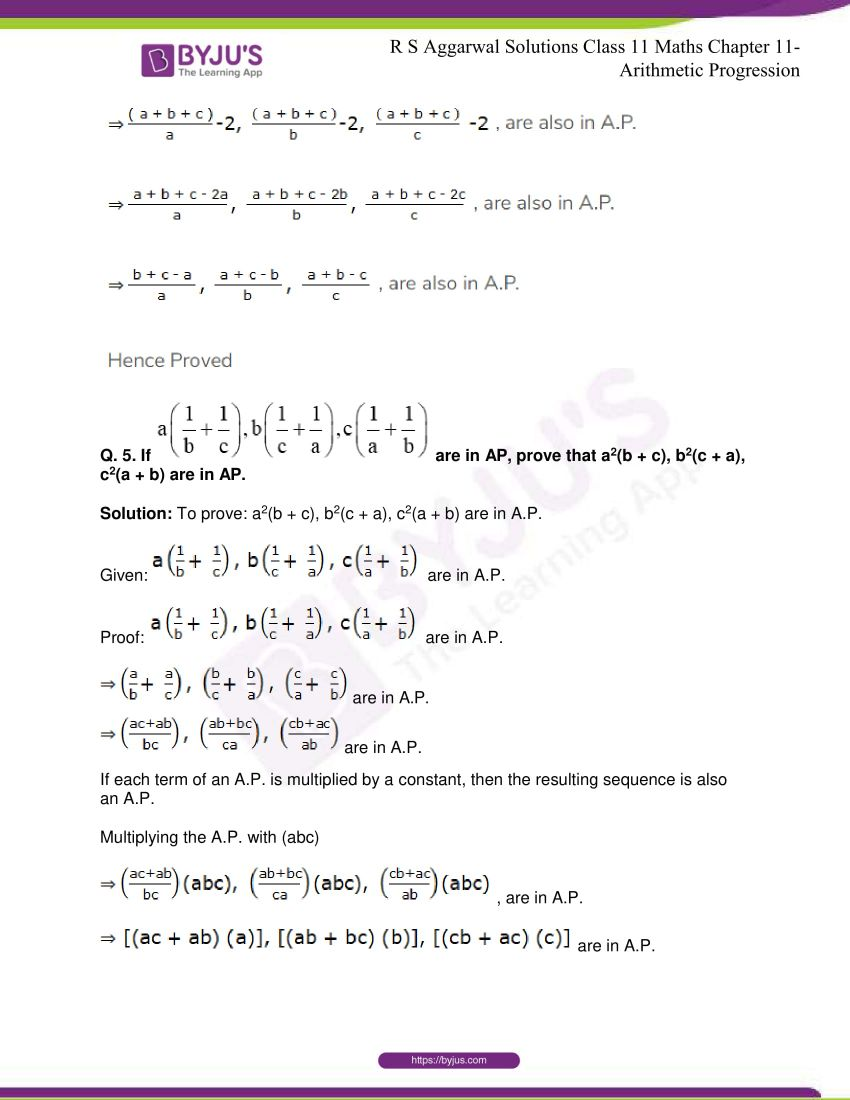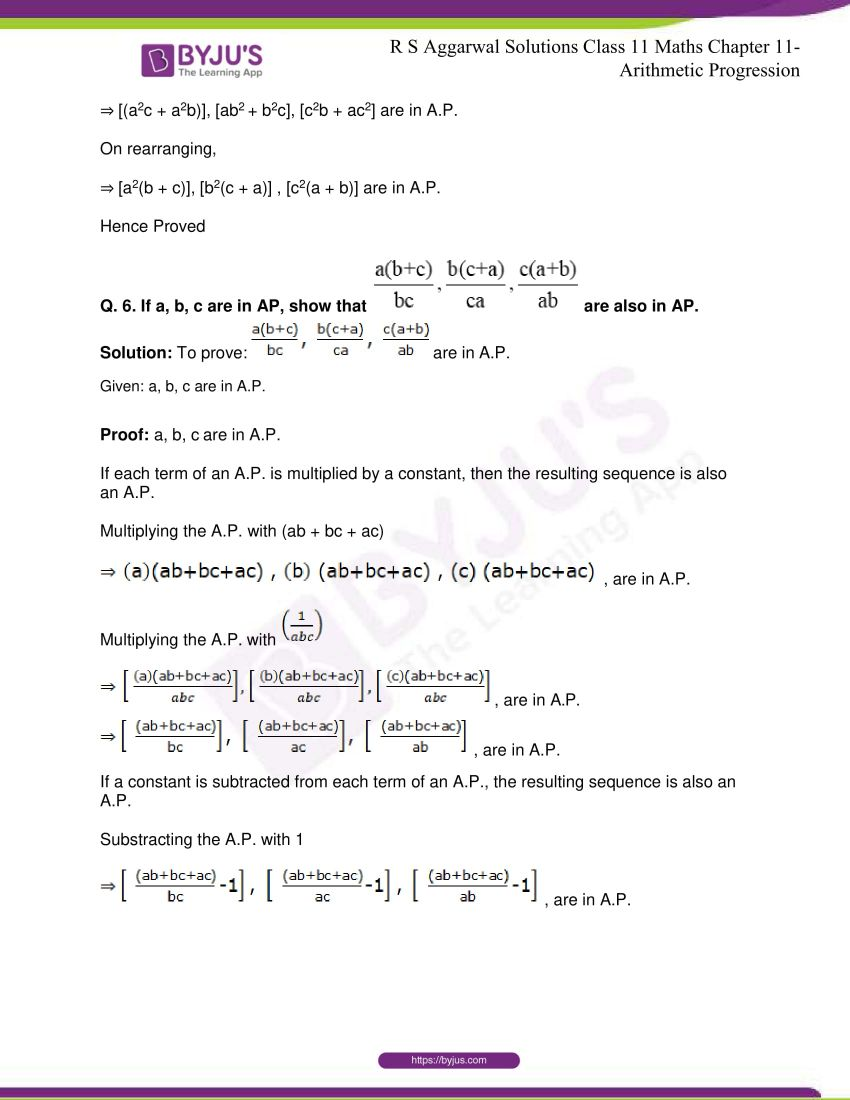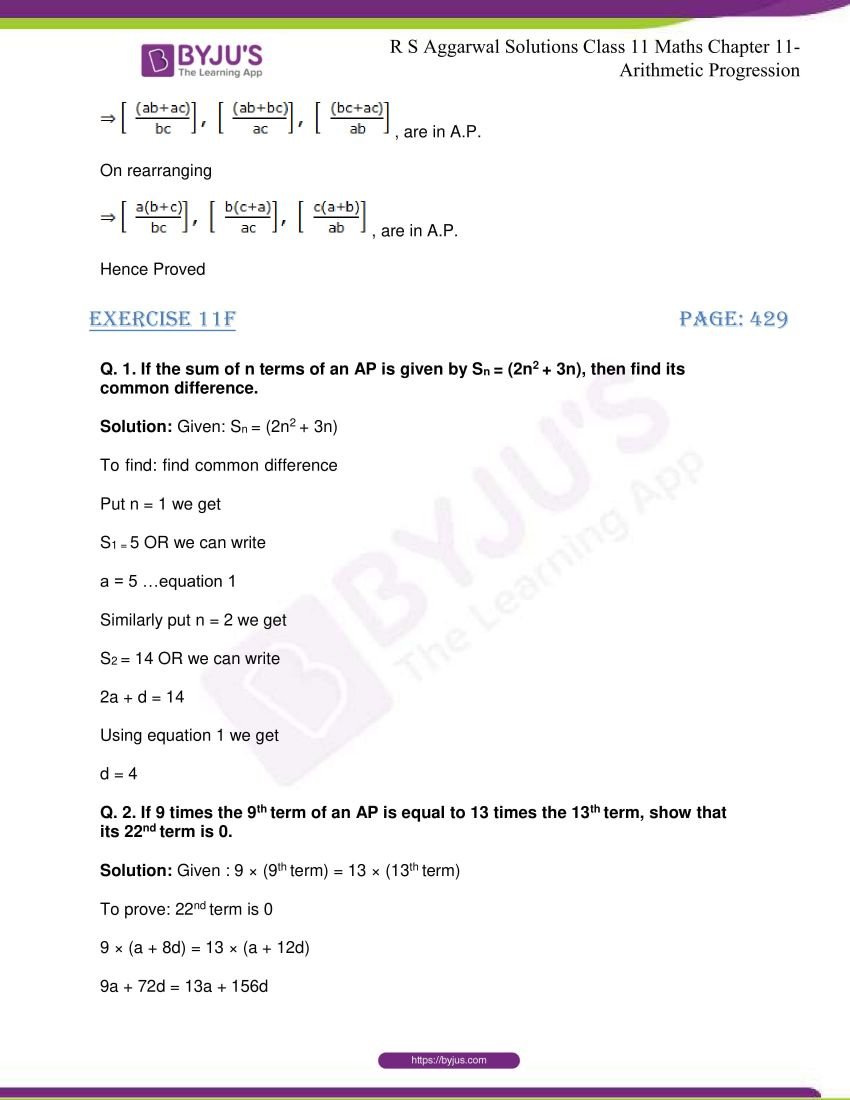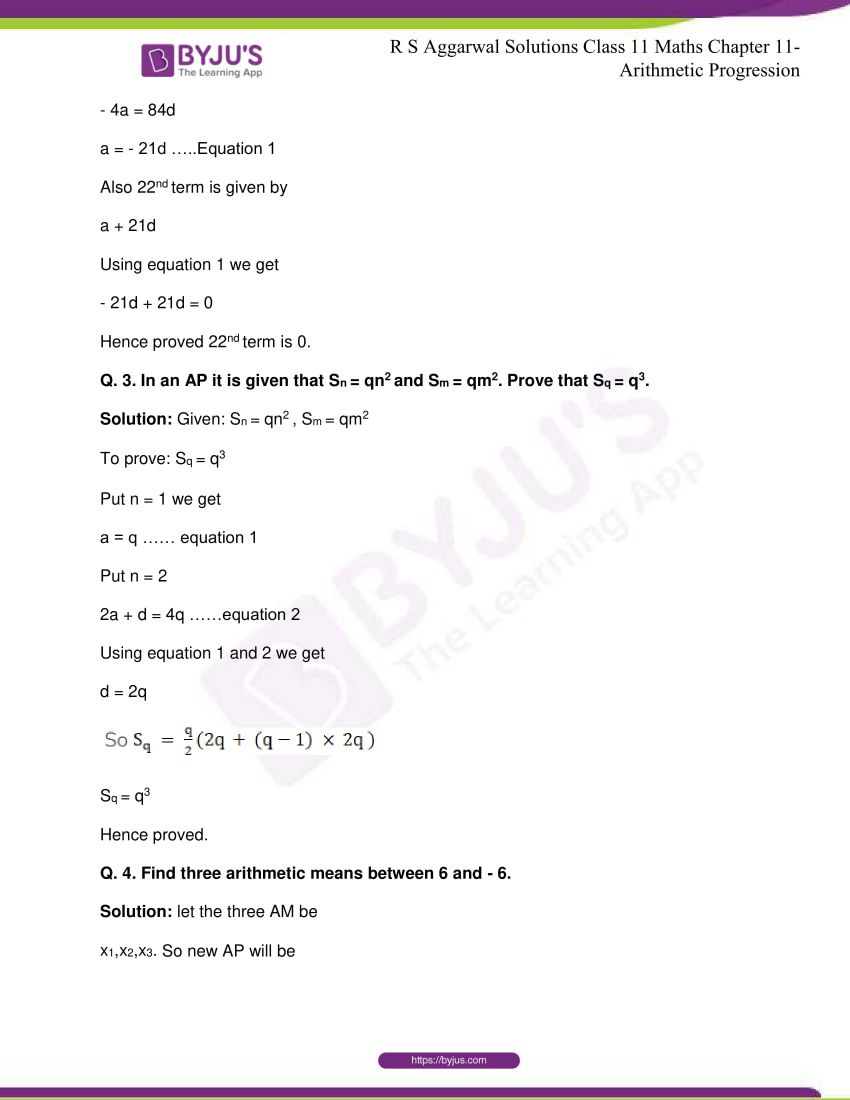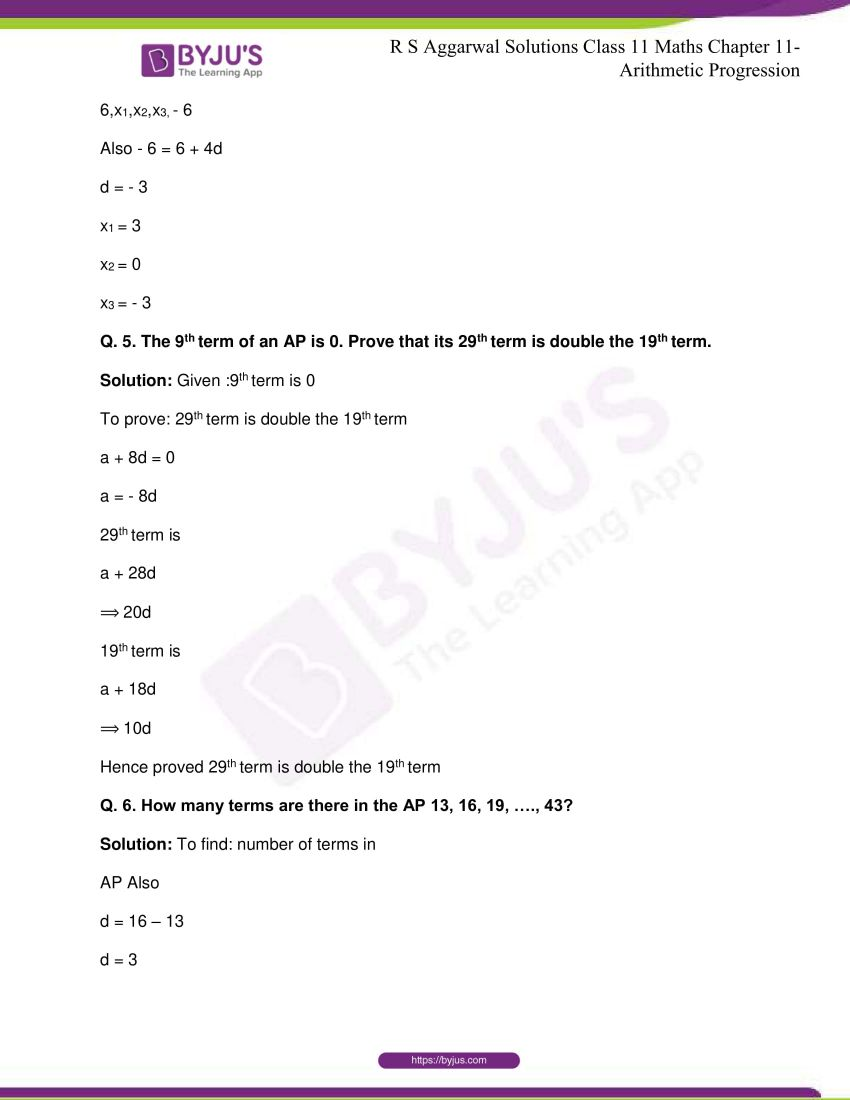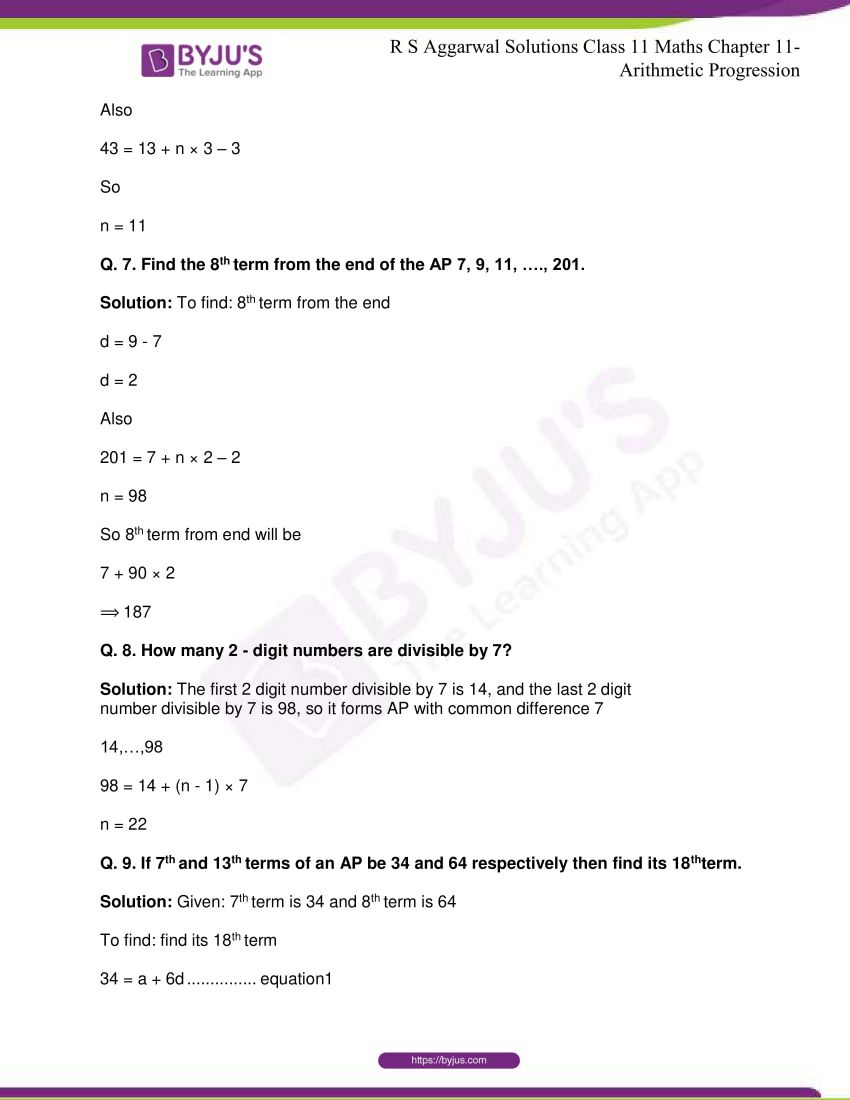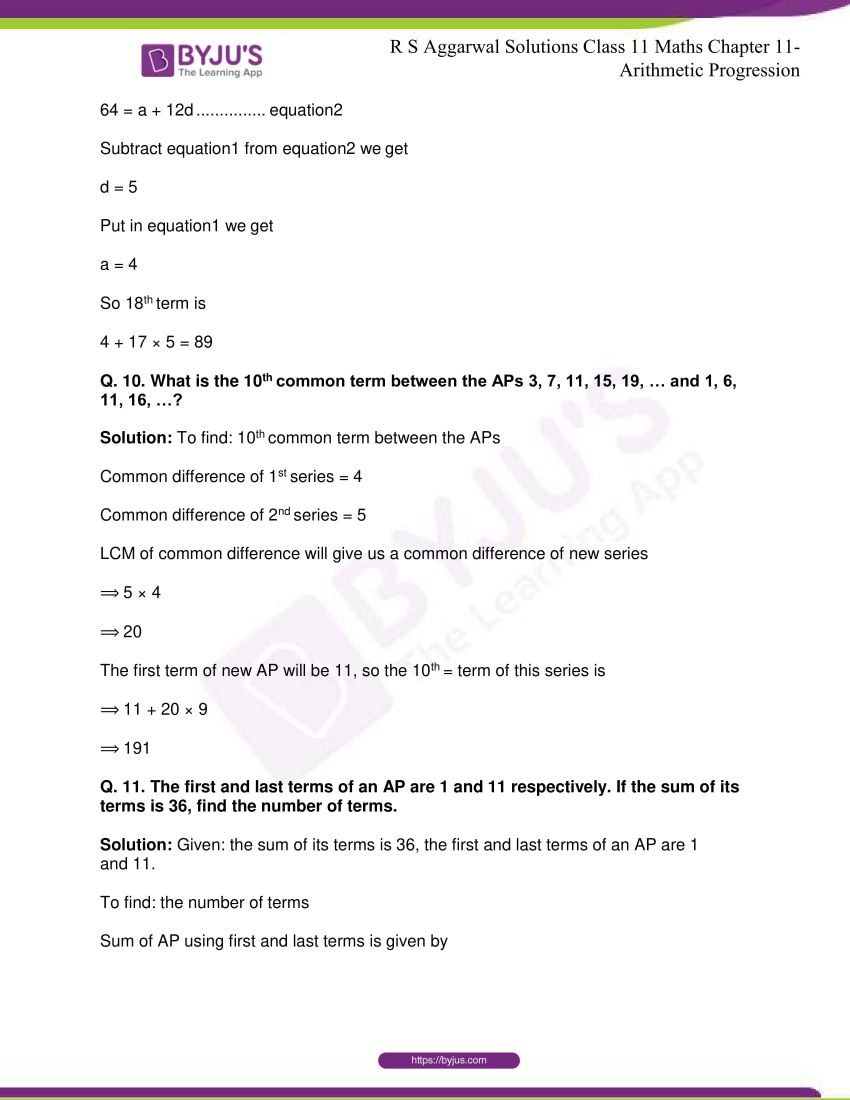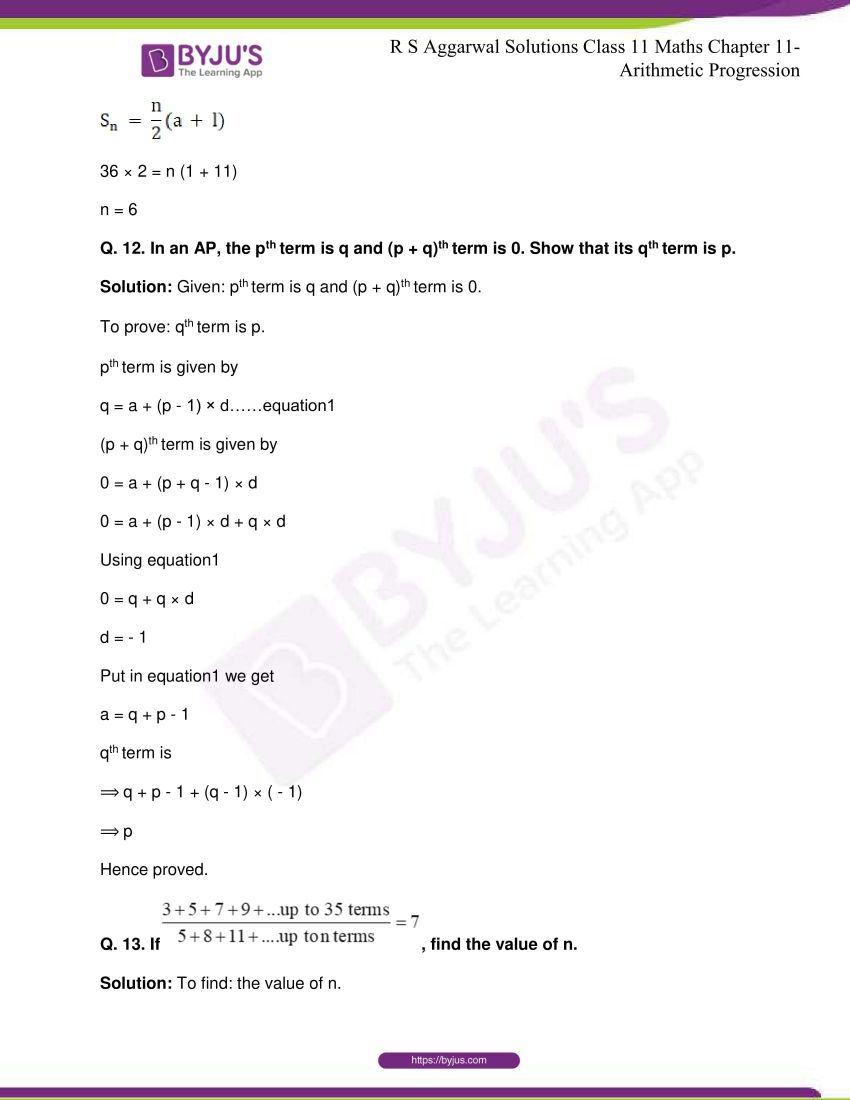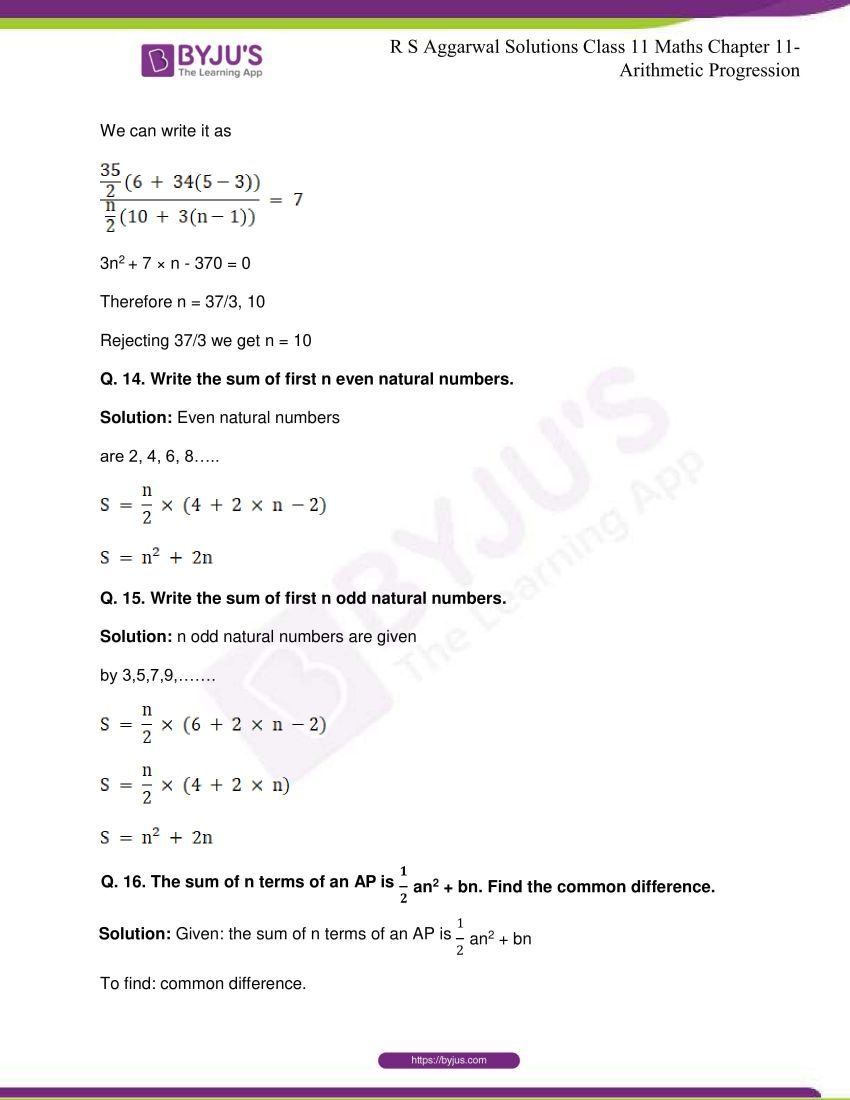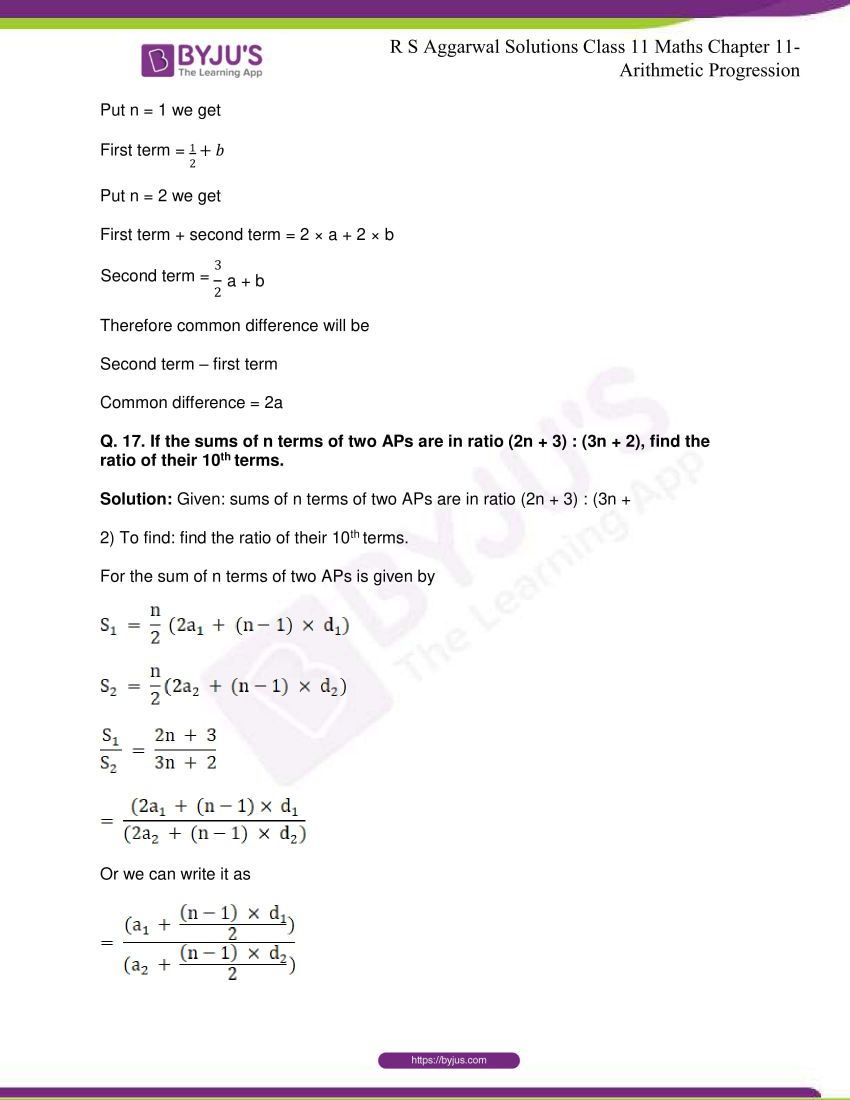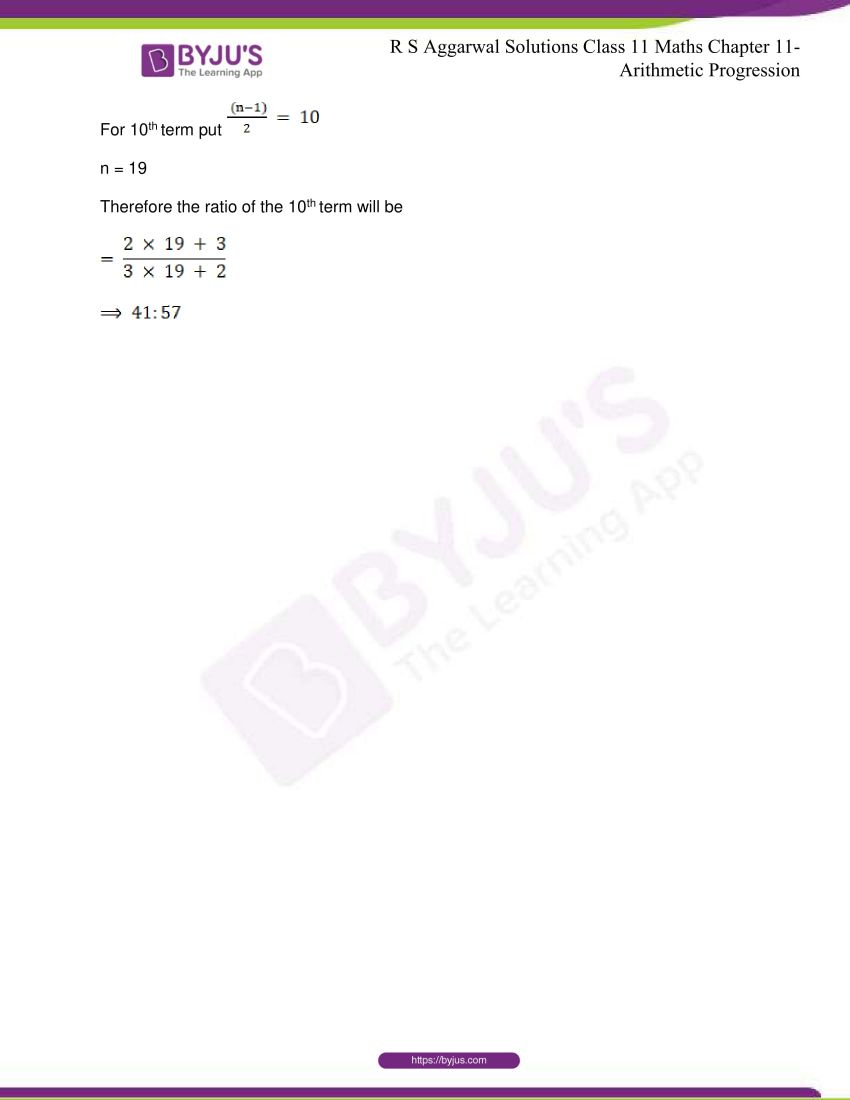## R S Aggarwal Solutions for Class 11 Maths Chapter 11- Arithmetic Progression

Chapter 11, Arithmetic Progression is composed of 6 exercises and the solutions given here contain answers to all the questions present in these exercises. Let us have a look at some of the topics that are being discussed in this chapter.

11.1 Arithmetic Progression

11.2 Properties of Arithmetic Progression

11.3 Problems Based on AP

11.4 Sum of n terms of an AP

## R S Aggarwal Solutions for Class 11 Maths Chapter 11- Arithmetic Progression

In Chapter 11 of Class 11, the students are taught about the topic, Arithmetic Progression. The chapter includes problems related to different concepts that fall under the topic of A P. Study Chapter 11 of R S Aggarwal textbook to understand more about Arithmetic Progression. Learn the R S Aggarwal Solutions for Class 11 effectively to come out with flying colours in the examinations.